Jekyll2023-09-26T11:08:24+00:00https://portfoliooptimizer.io/feed.xmlPortfolio OptimizerPortfolio Optimizer is a Web API democratizing the access to the Nobel Prize-winning science of portfolio optimization.Roman R.Range-Based Volatility Estimators: Overview and Examples of Usage2023-09-20T00:00:00+00:002023-09-20T00:00:00+00:00https://portfoliooptimizer.io/blog/range-based-volatility-estimators-overview-and-examples-of-usageVolatility estimation and forecasting plays a crucial role in many areas of finance.

For example, standard risk-based portfolio allocation methods (minimum variance, equal risk contributions, hierarchical risk parity…) critically depend on the ability to build accurate volatility forecasts1.

Multiple methods for estimating volatility have been proposed over the past several decades, and in this blog post I will focus on range-based volatility estimators.

These estimators, the first of which introduced by Parkinson2 as a way to compute the true variance of the rate of return of a common stock2, rely on the highest and lowest prices of an asset over a given time period to estimate its volatility, hence their name3.

After describing the four most well known range-based volatility estimators, I will reproduce the analysis of Arthur Sepp in his presentation Volatility Modelling and Trading4 made at Global Derivatives Conference 2016 and test the predictive power of the naive volatility forecasts produced by these estimators for various ETFs.

Notes:
A very accessible series of papers about range-based volatility estimators has recently5 been released by people at Lombard Odier, c.f. here, here and here.

## Mathematical preliminaries

### Volatility modelling

One of the main6 assumptions made when working with range-based volatility estimators7 is that the price movements $S_t$ of the asset under consideration follow a geometric Brownian motion with unknown volatility coefficient8 $\sigma$ and unknown drift coefficient $\mu$, that is

$d S_t = \mu S_t dt + \sigma S_t dW_t$

, where $W_t$ is a standard Brownian motion.

Under this working assumption, $\sigma$ represents the volatility of the asset.

### Volatility and variance estimators

Although anyone can empirically observe the impact of “volatility” on the prices of a given asset, the volatility coefficient $\sigma$ of this asset is not directly observable9 and must be estimated using stock market information.

A statistical estimator of $\sigma$ is then called a volatility estimator, and a statistical estimator of $\sigma^2$ is called a variance estimator.

### Efficiency of a volatility estimator

In order to determine the quality of a volatility estimator, two measures are commonly used:

• Bias

The bias of a volatility estimator measures whether this estimator produces, on average, too high or too low volatility estimates.

More formally, a volatility estimator $\sigma_A$ is said to be unbiased when $\mathbb{E}[\sigma_A] = \sigma$ and biased otherwise.

• Efficiency

The efficiency of a volatility estimator measures the uncertainty of the volatility estimates produced by this estimator, with the greater the efficiency of the estimator, the more accurate the volatility estimates.

More formally, the relative efficiency $Eff \left( \sigma_A \right)$ of a volatility estimator $\sigma_A$ compared to a reference volatility estimator $\sigma_B$ is defined as the ratio of the variance of the estimator $\sigma_B^2$ over the variance of the estimator $\sigma_A^2$, that is,

$Eff \left( \sigma_A \right) = \frac{Var \left( \sigma_B^2 \right)}{Var \left( \sigma_A^2 \right)}$

To be noted that bias and efficiency are sometimes conflicting, which is more generally known in statistics as the bias-variance tradeoff.

### Close-to-close volatility estimators

Let $C_1,…,C_T$ be the closing prices of an asset for $T$ time periods $t=1..T$10.

Then,

$\sigma_{cc,0} \left( T \right) = \sqrt{ \frac{1}{T-1} \sum_{i=2}^T \ln{\frac{C_i}{C_{i-1}}}^2 }$

is a biased11 estimator of the asset volatility $\sigma$ over the $T$ time periods, assuming zero drift (i.e., $\mu = 0$), c.f. Parkinson2.

$\sigma_{cc} \left( T \right) = \sqrt{ \frac{1}{T-2} \sum_{i=2}^T \left( \ln \frac{C_i}{C_{i-1}} - \mu_{cc} \right)^2 }$

, with $\mu_{cc} = \frac{1}{T-1} \sum_{i=2}^T \ln \frac{C_i}{C_{i-1}}$, is a biased11 estimator of the asset volatility $\sigma$ over the $T$ time periods, assuming non-zero drift (i.e., $\mu \ne 0$), c.f. Yang and Zhang12.

These two estimators are known as close-to-close volatility estimators.

## Range-based volatility estimators

Let be:

• $t=1..T$, $T$ time periods10
• $\left( O_1,H_1,L_1,C_1 \right), …, \left( O_T,H_T,L_T,C_T \right)$, the opening, highest, lowest and closing prices of an asset for time periods $t=1..T$

As mentioned in the introduction, a volatility estimator fully or partially relying on the highest prices $H_t, t=1..T$ and on the lowest prices $L_t, t=1..T$ is called a range-based volatility estimator.

The underlying idea behind such estimators is that information contained in the asset high-low price ranges $H_t - L_t, t=1..T$ should allow to build volatility estimators that are more efficient than the close-to-close volatility estimators, which use only one price inside this range13.

This quest for efficiency is important because, contrary to one of the working assumptions6, the volatility of an asset is known to be time-varying14, so that the less the number of time periods required to estimate its volatility, the more chances that its volatility is constant(ish) over the time periods under consideration.

As Rogers et al.15 put it:

[…] volatility may change over long periods of time; a highly efficient procedure will allow researchers to estimate volatility with a small number of observations.

### Parkinson volatility estimator

Parkinson2 introduces an estimator for the diffusion coefficient of a Brownian motion without drift that relies on the highest and lowest observed values of this Brownian motion over a given time period.

When applied to the estimation of an asset volatility, this gives the Parkinson volatility estimator $\sigma_{P} \left( T \right)$ defined over $T$ time periods by

$\sigma_{P} \left( T \right) = \sqrt{\frac{1}{T}} \sqrt{\frac{1}{4 \ln 2} \sum_{i=1}^T \left( \ln \frac{H_i}{L_i} \right) ^2}$

Intuitively, the Parkinson estimator should be “better” than the close-to-close estimators because large price movements impacting the high-low price range $H_t - L_t$ but leaving the closing price $C_t$ unchanged might occur within any time period $t$.

This is confirmed by the efficiency of this estimator, up to 5.2 times higher than the efficiency of the close-to-close estimators16.

### Garman-Klass volatility estimator

Garman and Klass17 propose to improve the Parkinson estimator by taking into account the opening prices $O_t, t=1..T$ and the closing prices $C_t, t=1..T$.

This leads to the Garman-Klass volatility estimator $\sigma_{GK} \left( T \right)$, defined over $T$ time periods by

$\sigma_{GK} \left( T \right) = \sqrt{\frac{1}{T}} \sqrt{ \sum_{i=1}^T \frac{1}{2} \left( \ln\frac{H_i}{L_i} \right) ^2 - \left( 2 \ln2 - 1 \right) \left( \ln\frac{C_i}{O_i} \right )^2 }$

For the historical comment, Garman and Klass17 establish in their paper that $\sigma_{GK}$ is the “best reasonable”18 volatility estimator that depends only on the high-open price range $H_t - O_t$, the low-open price range $L_t - O_t$ and the close-open price range $C_t - O_t$, $t=1..T$.

The Garman-Klass estimator is up to 7.4 times more efficient than the close-to-close estimators16.

### Rogers-Satchell volatility estimator

The Parkinson and the Garman-Klass estimators have both been derived under a zero drift assumption.

When this assumption is not verified for an asset, for example because of a strong upward or downward trend in the asset prices or because of the usage of large time periods (monthly, yearly…), these estimators should in theory not be used because the quality of their volatility estimates is negatively impacted by the presence of a non-zero drift1915.

In order to solve this problem, Rogers and Satchell19 devise the Rogers-Satchell volatility estimator $\sigma_{RS} \left( T \right)$, defined over $T$ time periods by

$\sigma_{RS} \left( T \right) = \sqrt{\frac{1}{T}} \sqrt{ \sum_{i=1}^T \ln\frac{H_i}{C_i} \ln\frac{H_i}{O_i} - \ln\frac{L_i}{C_i} \ln\frac{L_i}{O_i} }$

The Rogers-Satchell estimator is up to 6 times more efficient than the close-to-close estimators19, which is less than the Garman-Klass estimator20.

### Yang-Zhang volatility estimator

The range-based volatility estimators discussed so far do not take into account opening jumps in an asset prices21, that is, the potential difference between an asset opening price $O_t$ and its closing price $C_{t-1}$ for a time period $t$22.

This limitation causes a systematic underestimation of the true volatility12.

When trying to integrate opening jumps into the Parkinson, the Garman-Klass and the Rogers-Satchell estimators, Yang and Zhang12 discover that it is unfortunately not possible for any “reasonable” single-period23 volatility estimator to properly handle both a non-zero drift and opening jumps.

This leads them to introduce the multi-period23 Yang-Zhang volatility estimator $\sigma_{YZ} \left( T \right)$, defined over $T$ time periods by

$\sigma_{YZ} \left( T \right) = \sqrt{ \sigma_{ov}^2+ k \sigma_{oc}^2 + (1-k ) \sigma_{RS}^2 ) }$

, where:

• $\sigma_{co} \left( T \right)$ is the close-to-open volatility, defined as

$\sigma_{co} = \sqrt{\frac{1}{T-2} \sum_{i=2}^T \left( \ln \frac{O_i}{C_{i-1}} - \mu_{co} \right)^2}$

, with $\mu_{co} = \frac{1}{T-1} \sum_{i=2}^T \ln \frac{O_i}{C_{i-1}}$

• $\sigma_{oc}$ is the open-to-close volatility, defined as

$\sigma_{oc} \left( T \right) = \sqrt{\frac{1}{T-2} \sum_{i=2}^T \left( \ln \frac{O_i}{C_{i}} - \mu_{oc} \right)^2}$

, with $\mu_{oc} = \frac{1}{T-1} \sum_{i=2}^T \ln \frac{C_i}{O_{i}}$

• $\sigma_{RS}$ is the Rogers-Satchell volatility estimator over the time periods $t=2..T$

• $k = \frac{0.34}{1.34 + \frac{T}{T-2}}$

In addition to the new estimator $\sigma_{YZ}$, Yang and Zhang12 also provide multi-period versions of the Parkinson, the Garman-Klass and the Rogers-Satchell estimators that support opening jumps24.

The Yang-Zhang estimator is up to 14 times more efficient than the close-to-close estimators12, a result that Yang and Zhang12 comment as follows

The improvement of accuracy over the classical close-to-close estimator is dramatic for real-life time series

### Other estimators

The family of range-based volatility estimators has many other members:

• The Kunitomo25 volatility estimator
• The Meilijson27 volatility estimator

Still, the Parkinson, the Garman-Klass, the Rogers-Satchell and the Yang-Zhang volatility estimators are representative of this family, so that I will not detail any other range-based volatility estimator in this blog post.

## From volatility estimation to volatility forecasting

Range-based volatility estimators are based on the assumption of independent sample and observations within the sample4, so that the corresponding volatility forecasts are simply naive forecasts under a random walk model.

In other words, with such volatility estimators, the “natural” forecast of an asset volatility over the next $T$ time periods is the (past) estimate of the asset volatility over the last $T$ time periods.

That being said, it is perfectly possible to use range-based volatility estimates together with any volatility forecasting model such as:

• A time series forecasting model (simple moving average, exponentially weighted moving average…), as detailed for example in Jacob and Vipul28
• An econometric forecasting model (GARCH model…), c.f. Mapa29
• A specific range-based forecasting model (Chou’s30 Conditional AutoRegressive Range model, Harris and Yilmaz’s31 hybrid multivariate exponentially weighted moving average model…)

## Performance of range-based volatility estimators

Theoretical and practical performances of range-based volatility estimators are studied in several papers, for example Shu and Zhang32, Jacob and Vipul28 and Brandt and Kinlay33, among others.

Most of these studies agree that range-based volatility estimators are biased11, but other conclusions differ depending on the exact methodology used.

In particular, as highlighted by Brandt and Kinlay33, the results from empirical research differ significantly from those seen in simulation studies in a number of respects33.

One perfect example of these differences is Shu and Zhang32 concluding, using a Monte Carlo simulation, that

If the drift term is large, the Parkinson estimator and the [Garman-Klass] estimator will significantly overestimate the true variance […]

, while Jacob and Vipul28 concluding, using real stock market data, that

Overall, the [Garman-Klass] estimator, which indirectly adjusts for the drift, performs better for the high-drift stocks.

Motivated by such inconsistencies, Lyocsa et al.34, building on Patton and Sheppard35, introduced what I will call the Lyocsa-Plihal-Vyrost volatility estimator $\sigma_{LPV}$, defined as the arithmetic average of the Parkinson, the Garman-Klass and the Rogers-Satchell volatility estimators36

$\sigma_{LPV} = \frac{\sigma_{P} + \sigma_{GK} + \sigma_{RS}}{3}$

As Lyocsa et al.34 explain, the motivation behind using the (naive) equally weighted average is based on the assumption that we have no prior information on which estimator might be more accurate34.

I personally like the idea of an averaged estimator, but at this point, I think it is safe to highlight that there is no “best” range-based volatility estimator…

## Implementation in Portfolio Optimizer

Portfolio Optimizer implements all the volatility estimators discussed in this blog post:

, as well as their jump-adjusted variations, whenever applicable.

## Examples of usage

To illustrate possible uses of range-based volatility estimators, I propose to reproduce a couple of results from Sepp4:

• The estimation and the forecast of the SPY ETF monthly volatility
• The forecast of the monthly volatility of misc. ETFs representative of different asset classes (U.S. treasuries, international stock market, gold…)

Such examples will allow to compare the empirical behavior of the different volatility estimators and maybe reach a conclusion as to their relative performance in this specific setting.

### Estimating SPY ETF volatility

I will estimate the SPY ETF monthly volatility using all the daily open/high/low/close prices37 observed during that month38.

Figure 1, limited to 5 volatility estimators for readability purposes, illustrates the results obtained over the period 31 January 2005 - 29 February 201639.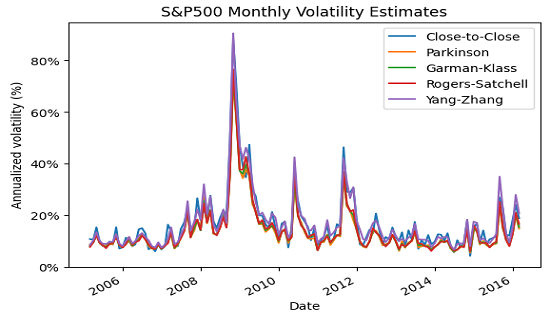Figure 1. SPY ETF monthly volatility estimates, using daily returns over the period 31 January 2005 - 29 February 2016.

Figure 1 is mostly identical to the figure on slide 22 from Sepp4, on which it seems in particular that the close-to-close and the Yang-Zhang volatility estimators provide higher estimates of volatility when the overall level of volatility is high4.

Overall, though, the behavior of the different volatility estimators is essentially the same on this specific example, which is confirmed by their correlations displayed in Figure 2.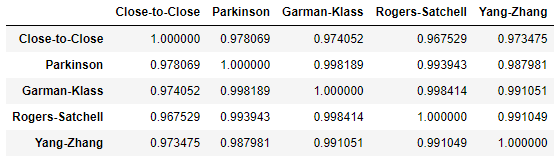Figure 2. Correlations of SPY ETF monthly volatility estimates, using daily returns over the period 31 January 2005 - 29 February 2016.

### Forecasting misc. ETFs volatility

Using the same methodology as in Sepp4, I will now evaluate the quality of the naive forecasts produced by all the range-based volatility estimators implemented in Portfolio Optimizer against the next month’s close-to-close observed volatility40, for 10 ETFs representative of misc. asset classes:

• U.S. stocks (SPY ETF)
• European stocks (EZU ETF)
• Japanese stocks (EWJ ETF)
• Emerging markets stocks (EEM ETF)
• U.S. REITs (VNQ ETF)
• International REITs (RWX ETF)
• U.S. 7-10 year Treasuries (IEF ETF)
• U.S. 20+ year Treasuries (TLT ETF)
• Commodities (DBC ETF)
• Gold (GLD ETF)

These ETFs are used in the Adaptative Asset Allocation strategy from ReSolve Asset Management, described in the paper Adaptive Asset Allocation: A Primer41.

For each ETF, Sepps’s methodology is as follows:

• At each month’s end, compute the volatility estimates $\sigma_{cc, t}$, $\sigma_{P, t}$, … using all the ETF daily open/high/low/close prices37 observed during that month38

Under a random walk volatility model, each of these estimates represents the next month’s volatility forecast $\hat{\sigma}_{t+1}$

• At each month’s end, also compute the next month’s close-to-close volatility estimate $\sigma_{cc, t+1}$ using all the ETF daily close prices37 observed during that month38

This estimate is the volatility benchmark, which represents how the ETF “volatility” is perceived by an investor monitoring her portfolio daily.

• Once all months have been processed that way, regress the volatility forecasts on the volatility benchmarks by applying the Mincer-Zarnowitz42 regression model:

$\hat{\sigma}_{t+1} = \alpha + \beta \sigma_{cc, t+1} + \epsilon_{t+1}$

, where $\epsilon_{t+1}$ is an error term.

Then, the estimator producing [the best] volatility forecast is indicated by [a] high explanatory power R^2, [a] small intercept $\alpha$ and [a] $\beta$ coefficient close to one4.

#### Forecasting SPY ETF volatility

In the case of the SPY ETF, Figure 3 illustrates Sepps’s methodology for the Lyocsa-Plihal-Vyrost volatility estimator $\sigma_{LPV}$ over the period 31 January 2005 - 29 February 2016.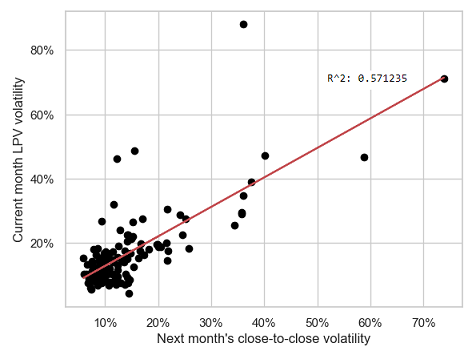Figure 3. SPY ETF Lyocsa-Plihal-Vyrost naive monthly volatility forecasts v.s. next month's close-to-close volatility estimates, using daily returns over the period 31 January 2005 - 29 February 2016.

Detailed results for all regression models over the period 31 January 2005 - 29 February 2016:

Volatility estimator $\alpha$ $\beta$ $R^2$
Close-to-close 4.1% 0.75 57%
Close-to-close (zero drift) 3.9% 0.77 57%
Parkinson 3.5% 0.95 58%
Garman-Klass 3.7% 0.92 57%
Garman-Klass (original) 3.7% 0.92 57%
Garman-Klass (original, jump-adjusted) 3.6% 0.77 58%
Rogers-Satchell 4.0% 0.88 56%
Yang-Zhang 3.8% 0.75 58%
Lyocsa-Plihal-Vyrost 3.7% 0.92 57%

While these figures are far43 from those on slide 42 from Sepp4, with for example nearly no variation in terms of $R^2$ among the different volatility estimators, two observations are similar:

• All volatility estimators have comparable $\alpha$
• The Parkinson, the Garman-Klass and the Rogers-Satchell volatility estimators have a $\beta$ much closer to 1 than the close-to-close volatility estimator

#### Forecasting the other ETFs volatility

Going beyond the SPY ETF, averaged results for all ETFs/regression models over each ETF price history44 are the following:

Volatility estimator $\bar{\alpha}$ $\bar{\beta}$ $\bar{R^2}$
Close-to-close 5.8% 0.66 44%
Close-to-close (zero drift) 5.6% 0.67 45%
Parkinson 5.6% 0.94 44%
Garman-Klass 5.7% 0.93 43%
Garman-Klass (original) 5.7% 0.93 43%
Garman-Klass (original, jump-adjusted) 5.0% 0.70 44%
Rogers-Satchell 6.1% 0.88 42%
Yang-Zhang 5.1% 0.69 44%
Lyocsa-Plihal-Vyrost 5.7% 0.92 43%

A couple of remarks:

• Forecasts produced by all the volatility estimators explain on average only ~45% of the variability of the ETFs monthly volatility
• Forecasts produced by the jump-adjusted volatility estimators seem to offer no improvement on average over the forecasts produced by the close-to-close volatility estimator
• Forecasts produced by the Parkinson, the Garman-Klass and the Rogers-Satchell volatility estimators seem to be much less biased on average than the forecasts produced by the close-to-close volatility estimator, a property inherited by the Lyocsa-Plihal-Vyrost volatility estimator

As an empirical conclusion, it is disappointing that the naive monthly volatility forecasts produced by range-based volatility estimators have about the same predictive power as the forecasts produced by the close-to-close volatility estimator. Nevertheless, because these forecasts are much less biased than their close-to-close counterparts, they still represent an improvement for the many investors who currently rely on close prices only45.

To also be noted, similar to one of the conclusions of Lyocsa et al.34, that the Lyocsa-Plihal-Vyrost volatility estimator should probably be preferred to the Parkinson, the Garman-Klass or the Rogers-Satchell volatility estimators because using only one range-based estimators has occasionally led to very inaccurate forecasts, which could successfully be avoided by using the average of the three range-based estimators34.

## Conclusion

One aspect of range-based volatility estimators not discussed in this blog post is their capability to capture important stylized facts about asset returns46.

This, together with possible ways to incorporate them in more predictive volatility models than the random walk model, will be the subject of future blog posts.

Meanwhile, for more volatile discussions, feel free to connect with me on LinkedIn or to follow me on Twitter.

1. As well as correlation forecasts.

2. See Parkinson, Michael H., The Extreme Value Method for Estimating the Variance of the Rate of Return, The Journal of Business 53 (1980), 61-65, which is the final version of the working paper The random walk problem: extreme value method for estimating the variance of the displacement (diffusion constant) started 4 years before.  2 3 4

3. Because the range of prices of an asset over a given time period is contained, by definition, within its highest and its lowest price.

4. At the date of publication of this post.

5. Other working assumptions are also commonly made, like assuming that the asset does not pay dividends, assuming that the volatility coefficient $\sigma$ remains constant, assuming that the geometric Brownian motion model also applies during time periods with no trading activity (e.g., stock market closure), etc.  2

6. In details, the geometric Brownian motion assumption slightly differs between authors; for example, Garman and Klass17 assume that asset prices follow a more generic diffusion process, which includes the geometric Brownian motion as a specific case.

7. $\sigma$ is also called the diffusion coefficient of the geometric Brownian motion, but in the context of this blog post, I think it is clearer to explicitly call it the volatility coefficient.

8. In practice, a time period $t$ usually corresponds to a trading day, a week or a month, so that the closing prices $C_t, t=1..T$ are simply the daily, weekly or monthly closing prices of the asset.  2

9. These estimators are biased, due to Jensen’s inequality; c.f. also Molnar46 2 3

10. The asset closing price $C_t, t=1..T$.

11. More precisely, Garman and Klass17 establish that a variation of $\sigma_{GK}$ is the “best” reasonable estimator but note that $\sigma_{GK}$ is 1) more practical and 2) as efficient as this variation, which I will call the original Garman-Klass volatility estimator $\sigma_{GKo}$.  2

12. Such a decrease in efficiency cannot be avoided because the Rogers-Satchell estimator belongs to class of estimators studied in Garman and Klass17, so that its efficiency is necessarily smaller than the efficiency of the Garman-Klass estimator (maximal by definition).

13. Garman and Klass17 provide a volatility estimator that takes into account opening jumps, but this estimator has a dependency on an unknown $f$ parameter which makes it unusable in practice; Yang and Zhang12 show that this dependency is actually spurious and provide a usable form of this estimator.

14. When the time periods $t$ are measured in trading days, opening jumps are called overnight jumps.

15. A single-period volatility estimator is a volatility estimator that can be used to estimate the volatility of an asset over a single time period $t$ using price data for this time period only; for example, the Parkinson, the Garman-Klass and the Rogers-Satchell estimators are single-period estimators while the close-to-close estimators are multi-period estimators.  2

16. C.f. also Molnar46 on this subject.

17. The Yang-Zhang volatility estimator is excluded to avoid mixing jump-adjusted volatility estimators with non-jump-adjusted ones.

18. (Adjusted) prices have have been retrieved using Tiingo 2 3

19. The jump-adjusted Yang-Zhang volatility estimator, as well as the close-to-close volatility estimators, require the closing price of the last day of the previous month as an additional price.  2 3

20. This period more or less matches with the period used in Sepp4

21. The next month’s close-to-close volatility is then taken as a proxy for the next month’s realized volatility; this choice is important, because different proxies might result in different conclusions as to the out-of-sample forecast performances.

22. This is due to slight differences in methodology, with mainly 1) the definition of “monthly volatility” in Sepp4 taken to be the volatility from the 3rd Friday of a month to the 3rd Friday of the next month and 2) the usage in Sepp4 of a linear regression model robust to outliers.

23. The common ending price history of all the ETFs is 31 August 2023, but there is no common starting price history, as all ETFs started trading on different dates.

24. For example, for all investors running some kind of monthly tactical asset allocation strategy.

]]>
Roman R.
Correlation Matrix Stress Testing: Random Perturbations of a Correlation Matrix2023-08-23T00:00:00+00:002023-08-23T00:00:00+00:00https://portfoliooptimizer.io/blog/correlation-matrix-stress-testing-random-perturbations-of-a-correlation-matrixIn the previous posts of this series, I detailed a methodology to perform stress tests on a correlation matrix by linearly shrinking a baseline correlation matrix toward an equicorrelation matrix or, more generally, toward the lower and upper bounds of its coefficients.

This methodology allows to easily model known unknowns when designing stress testing scenarios, but falls short with unknown unknows, that is, completely unanticipated correlation breakdowns. Indeed, by definition, these cannot be represented by an a-priori correlation matrix toward which a baseline correlation matrix could be shrunk1

In this blog post, I will describe another approach that can be used instead in this case, based on random perturbations of a baseline correlation matrix.

As an example of application, I will show how to identify extreme correlation stress scenarios through direct and reverse correlation stress testing.

Notes:
The main reference for this post is a presentation from Opdyke2 at the QuantMinds International 2020 event.

## Mathematical preliminaries

As a general reminder, a square matrix $C \in \mathcal{M} \left( \mathbb{R}^{n \times n} \right)$ is a (valid) correlation matrix if and only if

• $C$ is symmetric: $C {}^t = C$
• $C$ is unit diagonal: $C_{i,i} = 1$, $i=1..n$
• $C$ is positive semi-definite: $C \geqslant 0$

### Eigenvalue decomposition of a correlation matrix

A correlation matrix is a real symmetric matrix.

Thus, from standard linear algebra, any correlation matrix $C$ is diagonalizable by an orthogonal matrix and can be decomposed as a product

$C = P \Lambda P^{-1}$

, where:

• $P \in \mathcal{M} \left( \mathbb{R}^{n \times n} \right)$ is an orthogonal matrix
• $\Lambda = Diag \left( \lambda_{1},…, \lambda_{n} \right)$ $\in \mathcal{M} \left( \mathbb{R}^{n \times n} \right)$ is a diagonal matrix made of the $n$ eigenvalues $\lambda_1 \geq \lambda_2 … \geq \lambda_n \geq 0$ of $C$ which satisfy $\sum_{i=1}^{n} \lambda_i = n$

This decomposition is called the eigendecomposition of the correlation matrix $C$.

### Hypersphere decomposition of a correlation matrix

Rapisarda et al.3 establish that any correlation matrix $C \in \mathcal{M}(\mathbb{R}^{n \times n})$ can be decomposed as a product

$C = B B {}^t$

, where $B \in \mathcal{M}(\mathbb{R}^{n \times n})$ is a lower triangular matrix defined by

$b_{i,j} = \begin{cases} \cos \theta_{i,1}, \textrm{for } j = 1 \newline \cos \theta_{i,j} \prod_{k=1}^{j-1} \sin \theta_{i,k}, \textrm{for } 2 \leq j \leq i-1 \newline \prod_{k=1}^{i-1} \sin \theta_{i,k}, \textrm{for } j = i \newline 0, \textrm{for } i+1 \leq j \leq n \end{cases}$

with:

• $\theta_{1,1} = 0$, by convention
• $\theta_{i,j}$, $i = 2..n, j = 1..i-1$ $\frac{n (n-1)}{2}$ correlative angles belonging to the interval $[0, \pi]$

This decomposition is called the hypersphere decomposition, or the triangular angles parametrization4, of the correlation matrix $C$ and is detailed in the previous post of this series.

## Random perturbations of a correlation matrix

A random perturbation of a baseline correlation matrix $C$ can be loosely defined as a correlation matrix $\widetilde{C}$ generated “at random” whose correlation coefficients are more or less “close” to those of $C$.

From Opdyke’s2 extensive literature review, there are three main5 families of methods to generate random perturbations of a correlation matrix:

• Methods based on random perturbations of its correlation coefficients
• Methods based on random perturbations of its eigenvalues
• Methods based on random perturbations of its correlative angles

### Random perturbations of the coefficients of a correlation matrix

The first family of methods to randomly perturb a correlation matrix is based on random perturbations of its coefficients.

#### Naive method

The most natural method to randomly perturb the coefficients of a correlation matrix simply consists in … randomly perturbing these coefficients!

Unfortunately, this method does not work in general, because the resulting randomly perturbed correlation matrix is almost never a valid correlation matrix due to the lack of positive semi-definiteness.

To illustrate this problem, let’s take an Harry Browne’s permanent portfolio à la ReSolve, equally invested in:

• U.S. stocks, represented by the SPY ETF
• U.S. treasuries, represented by the IEF ETF
• Gold, represented by the GLD ETF
• Cash, represented by the SHY ETF

The correlations of these assets over the period 18 November 2004 - 11 August 2023 are displayed in Figure 1, adapted from Portfolio Visualizer.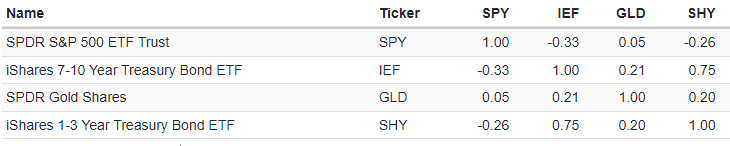Figure 1. SPY, IEF, GLD, SHY correlations over the period 18 November 2004 - 11 August 2023, based on daily returns. Source: Portfolio Visualizer.

Before thinking about perturbing all these correlations, let’s assume that we would merely like to perturb the U.S. stock-bond correlation so as to bring it to a level representative of the pre-2000 period, like 0.5 or above, c.f. Figure 2 reproduced from Brixton et al.6.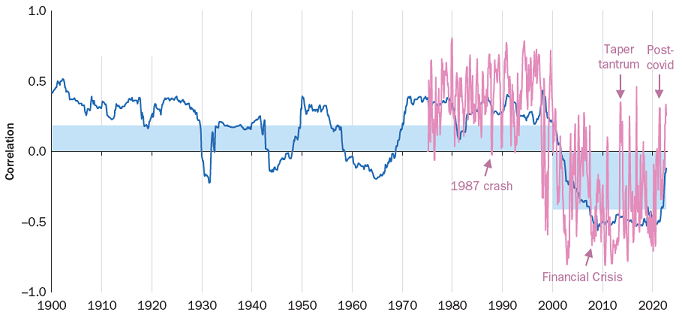Figure 2. Rolling Correlation between US Equity and US Treasury Returns, 01 January 1900 – 30 September 2022. Source: Brixton et al.

It turns out that this single perturbation already results in an invalid correlation matrix7!

As a consequence, trying to perturb the coefficients of a correlation matrix both simultaneously and at random has little chance to produce a valid correlation matrix in general, especially as the number of assets increases.

One solution to this issue is to replace the randomly perturbed correlation matrix by its nearest valid correlation matrix8, c.f. the post When a Correlation Matrix is not a Correlation Matrix: the Nearest Correlation Matrix Problem.

This leads to the following naive method to generate random perturbations of a correlation matrix $C \in \mathcal{M} \left( \mathbb{R}^{n \times n} \right)$:

1. Generate $\frac{n (n-1)}{2}$ randomly perturbed correlation coefficients $\widehat{C}_{i,j} \in [-1, 1]$ around the baseline correlation coefficients $C_{i,j}$, $i=1..n, j=i+1..n$
2. Compute the (potentially invalid) associated randomly perturbed correlation matrix $\widehat{C}$
3. Compute the randomly perturbed correlation matrix $\widetilde{C}$ as the nearest valid correlation matrix to $\widehat{C}$

While straightforward to implement9, this method has several limitations:

• It requires the computation of the nearest correlation matrix to every randomly perturbed correlation matrix generated

Such systematic computation is expensive.

• It usually10 generates randomly perturbed correlation matrices that are singular

This is because standard algorithms to compute the nearest correlation matrix, like Higham’s alternating projections algorithm8, output a singular correlation matrix11.

• It provides no guarantee on the magnitude or on the distribution of the perturbations

Due to the nearest correlation matrix step #3, it seems actually rather difficult to control either the magnitude or the probability distribution of the perturbations $\left | C_{i,j} - \widehat{C}_{i,j} \right |$, $i=1..n$, $j=i+1..n$.

#### Hardin et al.’s method

Hardin et al.12 introduce another method to randomly perturb the coefficients of a correlation matrix, relying on the dot product of normalized [independent gaussian random vectors]12 as random perturbations.

One of the many advantages of this method compared to the naive method previously described is that the resulting randomly perturbed correlation matrix is a valid correlation matrix by construction, which allows to bypass the nearest correlation matrix step #3.

In details, given $C \in \mathcal{M} \left( \mathbb{R}^{n \times n} \right)$ a baseline correlation matrix, Hardin et al.’s method to generate random perturbations of $C$ works as follows:

1. Select a maximum noise level $\epsilon_{max}$ such that $0 < \epsilon_{max} < \lambda_{n}$, where $\lambda_{n}$ is the smallest eigenvalue of $C$

$\epsilon_{max}$ controls the magnitude of the generated perturbations.

2. Select the dimension $m \geq 1$ of what is called the noise space in Hardin et al.12

$m$ influences the distributional characteristics of the random perturbations, as depicted in Figure 3 adapted from Hardin et al.12 on which it is visible that:

• $m = 3$ produces uniform-like perturbations (S3)
• $m = 25$ produces Gaussian-like perturbations (S25)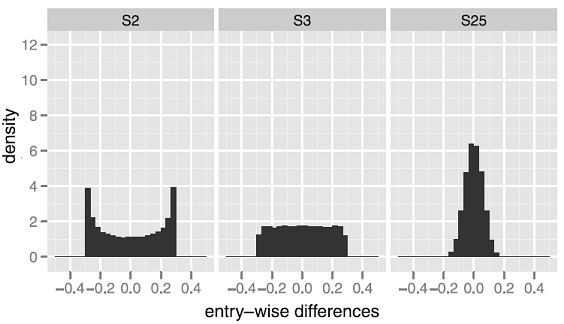Figure 3. Impact of the maximum noise level $\epsilon_{max}$ on the distribution of the perturbations (entry-wise differences). Source: Hardin et al.
3. Generate $n$ unit vectors $u_1,…,u_n$ belonging to $\mathbb{R}^{n}$ and construct the matrix $U \in \mathcal{M} \left( \mathbb{R}^{m \times n} \right)$ whose columns are the vectors $u_i, i=1..n$
4. Compute the randomly perturbed correlation matrix $\widetilde{C}$ as $\widetilde{C} = C + \epsilon_{max} \left( U{}^t U - I_n \right)$, where $I_n \in \mathcal{M} \left( \mathbb{R}^{n \times n} \right)$ is the identity matrix of order $n$

The definition of $\widetilde{C}$ ensures that the perturbations are bounded by the maximum noise level $\epsilon_{max}$, i.e., $\left | C_{i,j} - \widetilde{C}_{i,j} \right | \leq \epsilon_{max}$, $i=1..n$, $j=i+1..n$.

Whenever possible, Hardin et al.’s method should be used (computationally cheap, possibility to control the perturbations in terms of magnitude and distribution…), although it suffers from two major limitations:

• It is not applicable to correlation matrices singular or close to singular

This is due to the condition on the maximum noise level $\epsilon_{max}$ in step #1 and is regrettably a problem for applications in finance13, because as highlighted in Opdyke2:

correlation matrices estimated on large portfolios often (perhaps usually) are not positive definite for a wide range of reasons, and once positive definiteness is enforced using reliable, proven methods […], the smallest eigenvalue of the resulting matrix is almost always virtually zero.

• It might not be applicable to a specific correlation matrix, even if not remotely close to singular

This is again due to the condition on the maximum noise level $\epsilon_{max}$ in step #1.

For instance, in the case of the Harry Browne’s permanent portfolio introduced in the previous sub-section, Hardin et al.’s method cannot be used to perturb the coefficients of the asset correlation matrix represented in Figure 1 by more than +/- 0.2514, and in particular, cannot be used to generate perturbed U.S. stock-bond correlations higher than -0.0815!

### Random perturbations of the eigenvalues

The second family of methods to randomly perturb a correlation matrix is based on random perturbations of its eigenvalues.

A representative member of this family is the following method, with $C \in \mathcal{M} \left( \mathbb{R}^{n \times n} \right)$ a baseline correlation matrix to be perturbed:

1. Compute the eigendecomposition of $C$, with $C = P \Lambda P^{-1}$
2. Generate $n$ randomly perturbed eigenvalues $\widetilde{\lambda}_i \geq 0$ satisfying $\sum_{i=1}^n \widetilde{\lambda}_i = n$ around the baseline eigenvalues $\lambda_i$, $i=1..n$

Galeeva et al.4 describe several algorithms and associated probability distributions that can be used in this step.

3. Compute the associated randomly perturbed diagonal matrix $\widetilde{\Lambda} = Diag \left( \widetilde{\lambda}_1,…, \widetilde{\lambda}_n \right)$
4. Compute the randomly perturbed correlation matrix $\widetilde{C}$ as $\widetilde{C} = P^{-1} \widetilde{\Lambda} P$

Any method from this family guarantees, in theory, the validity of the resulting randomly perturbed correlation matrix.

Nevertheless, in practice, Opdyke2 notes that:

perturbing eigenvalues fails under challenging empirical conditions, e.g. when the positive definiteness of the matrix has to be enforced algorithmically […] and eigenvalues are virtually zero (or at least unreliably estimated)

In addition, controlling the $\frac{n (n-1)}{2}$ perturbations $\left | C_{i,j} - \widetilde{C}_{i,j} \right |$, $i=1..n$, $j=i+1..n$, which is ultimately what matters, through the $n$ perturbations $\left| \lambda_i - \widetilde{\lambda}_i \right|$, $i=1..n$, sounds rather difficult.

For these reasons, this family of methods might not be the first choice to generate random perturbations of a correlation matrix.

### Random perturbations of the correlative angles

The third and last family of methods to randomly perturb a correlation matrix is based on random perturbations of its correlative angles.

Here, a representative member of this family is the following method, with $C \in \mathcal{M} \left( \mathbb{R}^{n \times n} \right)$ a baseline correlation matrix to be perturbed:

1. Compute the16 hypersphere decomposition of $C$, with $C = B B {}^t$
2. Generate $\frac{n (n-1)}{2}$ randomly perturbed correlative angles $\widetilde{\theta}_{i,j} \in [0, \pi]$ around the baseline correlative angles $\theta_{i,j}$, $i=1..n$, $j=1..i-1$
3. Compute the associated randomly perturbed lower triangular matrix $\widetilde{B}$
4. Compute the randomly perturbed correlation matrix $\widetilde{C}$ as $\widetilde{C} = \widetilde{B} \widetilde{B} {}^t$

Any method from this family again guarantees, in theory, the validity of the resulting randomly perturbed correlation matrix.

This time, though, theory seems to be confirmed in practice:

• Galeeva et al.4 highlight that perturbing the correlative angles is done via a robust and efficient procedure which makes the whole approach very attractive4
• Opdyke2 notes that perturbing the correlative angles appears to be more robust [in practice] than competing methods […] at least under challenging empirical conditions2

One important remark at this stage is that the exact algorithms and associated probability distributions used in step #2 greatly influence the behavior of this family of methods.

For reference, Opdyke2 proposes an algorithm called Cosecant, Cotangent, Cotangent (C3) able to generate a distribution of correlative angles median-centered on the baseline correlative angles and satisfying many other desirable properties17.

This algorithm generates a randomly perturbed correlative angle $\widetilde{\theta}_{i,j}$ around a baseline correlative angle $\theta_{i,j}$, $i=1..n$, $j=1..i-1$, as follows:

1. Generate a random variable $X$ of probability density function the p.d.f. of Makalic and Schmidt18 defined by $f_{X}(x) = c_k sin^k (x)$, $x \in (0, \pi)$, $k \geq 1$, where $c_k$ is a normalization constant and $k = n - j$
2. Compute the perturbed correlative angle $\widetilde{\theta}_{i,j}$ as $\widetilde{\theta}_{i,j} = \arctan \left( \tan \left( \theta_{i,j}-\frac{\pi}{2} \right) + \tan \left( X - \frac{\pi}{2} \right) \right) + \frac{\pi}{2}$

This family of methods is particularly well-suited to what is called generalized (correlation) stress testing in Opdyke2.

More on this later.

Still, like the family of methods based on random perturbations of the eigenvalues of a correlation matrix, one limitation of this family of methods is that controlling the $\frac{n (n-1)}{2}$ perturbations $\left | C_{i,j} - \widetilde{C}_{i,j} \right |$, $i=1..n$, $j=i+1..n$ sounds once again rather difficult.

## Implementation in Portfolio Optimizer

Portfolio Optimizer allows to generate random perturbations of a baseline correlation matrix with:

• The naive method of randomly perturbing the coefficients of a correlation matrix

Once a (potentially invalid) randomly perturbed correlation matrix is generated on client side, the endpoint /assets/correlation/matrix/nearest can be used to compute the nearest correlation matrix to this matrix.

• The method of randomly perturbing the correlative angles of a correlation matrix

• Together with Opdyke’s C3 algorithm2, through the endpoint /assets/correlation/matrix/perturbed

• Together with a proprietary algorithm able to control the magnitude of the perturbations of the correlation coefficients, again through the endpoint /assets/correlation/matrix/perturbed

In this case, the distribution of the randomly perturbed correlation matrices is asymptotically uniform over the space of positive definite correlation matrices whose distance in terms of max norm to the baseline correlation matrix is at most equal to (resp. exactly equal to) a given maximum noise level (resp. a given exact noise level), similar in spirit to the method of Hardin et al.12

## Example of application - Generalized stress testing

Suppose that we are managing the Harry Browne’s permanent portfolio introduced earlier.

Suppose also that on 18 February 2020, we feel something is off and would like to assess the impact of a potential correlation breakdown on this portfolio.

Because this potential correlation breakdown could manifest in many ways (increased correlations between certain ETFs, decreased correlation between other ETFs…), it would be a mistake to impose any prior on how correlations should behave or should not behave19.

So, what could we do?

### Direct correlation stress testing

Following the previous sections, one possibility is to generate random perturbations around the current correlation matrix of the ETFs in the portfolio, which will allow to simulate many potential correlation breakdowns in a prior-free way.

Once this is done, it will then be possible to evaluate the portfolio sensitivity to these random shocks.

Such a direct (correlation) stress testing procedure allows to catch difficult-to-anticipate and/or difficult-to quantify second and third order effects of a large, multivariate, impactful scenario (e.g. pandemic + economic upheaval)2.

In order to apply this procedure to the portfolio at hand, three prerequisites are necessary:

• Estimating the current correlation matrix $C_{PP}$ of the ETFs in the portfolio

I will estimate $C_{PP}$ as the correlation matrix of the four ETFs in the portfolio20 over the 24-day period 14 January 2020 - 18 February 202021, which gives

$C_{PP} \approx \begin{pmatrix} 1 & -0.81 & -0.82 & -0.65 & \\ -0.81 & 1 & 0.84 & 0.70 \\ -0.82 & 0.84 & 1 & 0.75 \\ -0.65 & 0.70 & 0.75 & 1 \end{pmatrix}$
• Selecting a method to randomly perturb the current correlation matrix $C_{PP}$

I will generate random perturbations of $C_{PP}$ thanks to Opdyke’s C3 algorithm2 as implemented through the Portfolio Optimizer endpoint /assets/correlation/matrix/perturbed.

• Determining how to evaluate the portfolio sensitivity to the random perturbations of the current correlation matrix $C_{PP}$

To keep things simple, I will evaluate the portfolio effective number of bets22 (ENB), using the Portfolio Optimizer endpoint /portfolio/analysis/effective-number-of-bets.

With these prerequisites met, it is possible to generate random perturbations around the current correlation matrix $C_{PP}$ and compute the corresponding ENB distribution.

An example of ENB distribution is provided in Figure 5, in the case of 10000 randomly perturbed correlation matrices.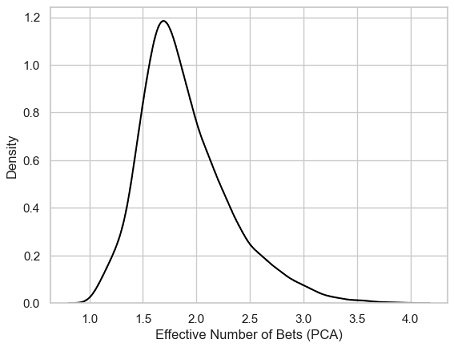Figure 5. Distribution of the Effective Number of Bets (ENB), 10000 randomly perturbed correlation matrices around the current correlation matrix $C_{PP}$.

Some associated summary statistics:

 Mean 1.89 Standard deviation 0.42 Minimum 1.01 5% percentile 1.33 25% percentile 1.6 Median 1.81 75% percentile 2.12 95% percentile 2.72 Maximum 3.98

And, for reference, the value of the current ENB of the portfolio, computed with the current correlation matrix $C_{PP}$: 1.87.

• More than half of the ENB are located very close23 to the current ENB (1.87)

These ENB are not representative of any real correlation breakdown.

• The 95% percentile of the ENB distribution (2.72) is much further apart from the current ENB (1.87) than the 5% percentile of the ENB distribution (1.33)

This means that a correlation breakdown with the biggest impact on the ENB would correspond, maybe counter-intuitively, to a scenario of de-correlation24 of the four ETFs in the portfolio.

As a side note, and again maybe counter-intuitively, the impact of such a correlation breakdown would then be rather harmless, because an increase in ENB is usually desirable from a portfolio diversification perspective.

• The minimum (1.01) and maximum (3.98) ENB both correspond to the theoretical minimum (1) and maximum (4) ENB

This shows that all possible (correlation) unknown unknowns have been covered by the stress testing procedure.

### Reverse correlation stress testing

In the previous sub-section, we have (empirically) established that the most impactful correlation breakdown scenario for the ENB of the portfolio corresponds to a de-correlation of the ETFs.

The next logical step is now to compute a correlation matrix that would somehow best illustrate this de-correlation scenario, a procedure known as reverse (correlation) stress testing.

For this, inspired by the concept of market states from Stepanov et al25, I propose to apply a k-means clustering algorithm26, with $k = 2$, to the randomly perturbed correlation matrices generated during the direct stress testing procedure.

One output of this algorithm is two “representative” correlation matrices27, which are, in the case of the 10000 randomly perturbed correlation matrices of the previous sub-section:

• A correlation matrix $\widetilde{C}_{PP,1}$ “representative” of all the randomly perturbed correlation matrices that are “maximally similar” to the current correlation matrix $C_{PP}$

$\widetilde{C}_{PP,1} \approx \begin{pmatrix} 1 & -0.76 & -0.78 & -0.67 & \\ -0.76 & 1 & 0.75 & 0.66 \\ -0.78 & 0.75 & 1 & 0.67 \\ -0.67 & 0.66 & 0.67 & 1 \end{pmatrix}$
• A correlation matrix $\widetilde{C}_{PP,2}$ “representative” of all the randomly perturbed correlation matrices that are “maximally dissimilar” from $\widetilde{C}_{PP,1}$

$\widetilde{C}_{PP,2} \approx \begin{pmatrix} 1 & -0.64 & -0.64 & -0.12 & \\ -0.64 & 1 & 0.53 & 0.07 \\ -0.64 & 0.53 & 1 & 0.06 \\ -0.12 & 0.07 & 0.06 & 1 \end{pmatrix}$

, with “representative”, “maximally similar” and “maximally dissimilar” loosely defined but usually corresponding to intuition28.

In terms of market states25:

• The correlation matrix $\widetilde{C}_{PP,1}$ embodies the current market state

Indeed, $\widetilde{C}_{PP,1}$ is very close to $C_{PP}$, as confirmed by the small Frobenius distance between these two matrices (0.21).

In the current market state, the ENB is concentrated29 around the current ENB of the portfolio (1.87).

• The correlation matrix $\widetilde{C}_{PP,2}$ embodies a market state maximally distinct from the current market state, which I will call the de-correlation market state

The rationale for this name is that a comparison between $C_{PP}$ and $\widetilde{C}_{PP,2}$ shows that this second market state corresponds to a de-correlation of the four ETFs in the portfolio30.

In the de-correlation market state, the ENB is much higher that in the current market state, with for example the ENB computed with the correlation matrix $\widetilde{C}_{PP,2}$ equal to 2.97, well above the 95% percentile of the ENB distribution (2.72).

Thanks to these observations, it is possible to conclude that $\widetilde{C}_{PP,2}$ is the correlation matrix that best illustrate the most impactful correlation breakdown scenario for the ENB of the portfolio.

### Reality check

I will conclude this example on generalized stress testing by a reality check on the results obtained in the previous sub-sections.

The correlation matrix $C_{PP, COVID}$ below is the correlation matrix of the four ETFs in the portfolio20 over the subsequent 24-day “full crisis” period 19 February 2020 - 23 March 202021.

$C_{PP, COVID} \approx \begin{pmatrix} 1 & -0.50 & -0.40 & 0.00 & \\ -0.50 & 1 & 0.71 & 0.25 \\ -0.40 & 0.71 & 1 & 0.19 \\ 0.00 & 0.25 & 0.19 & 1 \end{pmatrix}$

Of particular interest:

• The resemblance between $C_{PP, COVID}$ and $\widetilde{C}_{PP,2}$, confirmed by a relatively small Frobenius distance between these two matrices (0.58)
• The close match between the ENB computed with $C_{PP, COVID}$ (2.84) and the ENB computed with $\widetilde{C}_{PP,2}$ (2.97)

In other words:

• The most theoretically impactful correlation breakdown scenario for the ENB of the portfolio actually occurred in practice, with an associated asset correlation matrix relatively close to the forecast asset correlation matrix
• The forecast of the impact on the ENB of the portfolio of this theoretical correlation breakdown scenario was nearly spot on!

## Conclusion

The possibility to generate random perturbations of a correlation matrix has many other applications in risk management and even beyond.

As an example, in mean-variance optimization, the resampled efficient frontier is partially based on random perturbations of a baseline correlation matrix.

Also, as a last remark on Opdyke’s C3 algorithm2, a fully nonparametric version of it is described on Opdyke’s website.

This extended version, called Nonparametric Angles-based Correlation (NAbC), covers not only correlation matrices based on any underlying data distributions31 but also correlation matrices beyond the standard Pearson’s correlation matrix, like Spearman’s Rho correlation matrix or Kendall’s Tau correlation matrix.

For more random quantitative discussions, feel free to connect with me on LinkedIn or to follow me on Twitter.

1. Otherwise, these unknown unknows would become known unknows!

2. Of course, many other methods exist; for example, if the data generating process is known, it is possible to use a Monte-Carlo method to generate random samples from this process and compute their associated (sample) correlation matrix, which is then a perturbed version of the original correlation matrix.

3. I’ll skip the math, but the interested reader can for example compute the eigenvalues of the asset correlation matrix represented in Figure 1 with the U.S. stock-bond correlation altered from -0.33 to 0.5.

4. Assuming that an algorithm to compute the nearest correlation matrix is available; otherwise, this method becomes immediately less straightforward to implement…

5. Except if the initial randomly perturbed correlation matrices are actually valid, non-singular, correlation matrices.

6. It is sometimes possible, though, to integrate an additional constraint on the minimum eigenvalue of the computed nearest valid correlation matrix into these algorithms.

7. This is maybe less of a problem in other applications, like in biology.

8. Because the smallest eigenvalue of the asset correlation matrix represented in Figure 1 is 0.25.

9. Similarly, Hardin et al.’s method cannot be used to generate random pertubations of the U.S. stock-bond correlation that would bring this correlation to a level lower than -0.58.

10. Strictly speaking, when the correlation matrix $C$ is positive semi-definite, its hypersphere decomposition is not unique.

11. C.f. Opdyke2 for the complete list of goals of his proposed approach.

12. For example, assuming that all correlations would go to one in case of a correlation breakdown is a prior.

13. More specifically, of the daily arithmetic total returns of the four ETFs in the portfolio, whose prices have been retrieved using Tiingo 2

14. I used a 24-day period because the period 19 February 2020 - 23 March 2020, which corresponds to the peak of the COVID financial crisis - c.f. Wikipedia - is also a 24-day period.  2

15. Due to personal preferences, I will use the effective number of bets based on principal components analysis as the factors extraction method; in addition, I will use the asset correlation matrix as if it were the asset covariance matrix to not introduce any additional variables (volatilities).

16. More precisely, within a +/- 0.30 interval around 1.87.

17. Intuitively, the higher the ENB of an equally-weighted portfolio, the more uncorrelated its constituents.

18. I used the standard Scikit-Learn $k$-means algorithm.

19. The k-means algorithm does not guarantee that the cluster centroids are valid correlation matrices; if this is not the case, it is possible to use either the $k$-medoids instead, or to compute the nearest correlation matrices to the cluster centroid.

20. And more rigorously defined as per the $k$-means algorithm.

21. To be noted that the ENB computed with the correlation matrix $C^{‘}_{PP,1}$ is nearly identical to the ENB computed with the current correlation matrix $C_{PP}$ (1.87).

22. For example, U.S. stocks and Gold move from anti-correlated (-0.65) to nearly uncorrelated (-0.12).

23. That is, data distributions characterized by any degree of serial correlation, asymmetry, non-stationarity, and/or heavy-tailedness.

]]>
Roman R.
Managing Missing Asset Returns in Portfolio Analysis and Optimization: Backfilling through Residuals Recycling2023-07-26T00:00:00+00:002023-07-26T00:00:00+00:00https://portfoliooptimizer.io/blog/managing-missing-asset-returns-in-portfolio-analysis-and-optimization-backfilling-through-residuals-recyclingIn a multi-asset portfolio, it is usual that some assets have shorter return histories than others1.

Problem is, the presence of assets whose return histories differ in length makes it nearly impossible to use standard portfolio analysis and optimization methods…

Estimating the historical covariance matrix of a multi-asset portfolio, for example, is not possible when assets have unequal return histories, so that a typical workaround used in practice is to consider only the common returns history. Unfortunately, this workaround has the side effect of discarding information contained in the longer return histories, which might greatly impact the quality of the estimated covariance matrix2.

Sebastien Page proposes a solution to this problem in his paper How to Combine Long and Short Return Histories Efficiently3. It consists in simulating missing asset returns based on the relationships observed between all assets over their common returns history while accounting for the associated estimation error.

In this blog post, I will describe in detail Page’s method and analyze how it behaves empirically with a two-asset class portfolio made of U.S. and E.M. stocks.

Notes:

## Page’s method to backfill missing asset returns

### Single starting date

Let be two groups of assets $X$ and $Y$ such that:

• The “long” group of assets $X = \left( X_1,…,X_n \right)$ is made of $n \geq 1$ assets, all sharing together a common returns history of length $L$
• The “short” group of assets $Y = \left( Y_1,…,X_m \right)$ is made of $m \geq 1$ assets, all sharing together a common returns history of length $S < L$ as well as sharing a common (ending) returns history of length $L - S + 1$ with the group of assets $X$

In such a situation, illustrated in Figure 1 adapted from Page3, returns for the group of assets $Y$ are missing for the whole (beginning) returns history $t = 1..L - S$.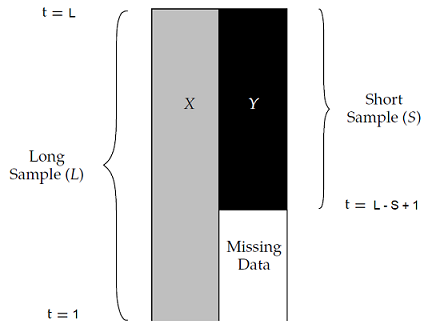Figure 1. Missing asset returns with a single starting date. Source: Page.

Building on the maximum likelihood procedure4 described in Stambaugh5, Page3 introduces a 3-step method in order to combine [these] long and short return histories efficiently3 and backfill the $m \times \left( L - S \right)$ missing asset returns.

#### Step 1 - Estimation of the asset “long” mean returns

The vector $\hat{\mu}_{Y,L} \in \mathbb{R}^{m}$ of the mean returns of the assets belonging to the group $Y$ is estimated over the long returns history by:

$\hat{\mu}_{Y,L} = \mu_{Y,S} + \beta \left( \mu_{X,L} - \mu_{X,S} \right)$

, with:

• $\mu_{Y,S} = \left( \mu_{Y_1,S}, …, \mu_{Y_m,S} \right) {}^t \in \mathbb{R}^{m}$ the vector of the mean returns of the assets belonging to the group $Y$, computed over the short returns history
• $\beta \in \mathcal{M}(\mathbb{R}^{m \times n})$, the vector of standard regression coefficients defined by $\beta = \Sigma_{XX,S}^{-1} \Sigma_{XY,S}$, with:
• $\Sigma_{XX,S} \in \mathcal{M}(\mathbb{R}^{n \times n})$ the covariance matrix of the assets belonging to the group $X$, computed over the short returns history
• $\Sigma_{XY,S} \in \mathcal{M}(\mathbb{R}^{m \times n})$ the covariance matrix between the assets belonging to the group $X$ and the assets belonging to the group $Y$, computed over the short returns history
• $\mu_{X,L} = \left( \mu_{X_1,L}, …, \mu_{X_n,L} \right) {}^t \in \mathbb{R}^{n}$, the vector of the mean returns of the assets belonging to the group $X$, computed over the long returns history
• $\mu_{X,S} = \left( \mu_{X_1,S}, …, \mu_{X_n,S} \right) {}^t\in \mathbb{R}^{n}$, the vector of the mean returns of the assets belonging to the group $X$, computed over the short returns history

#### Step 2: Estimation of the asset long covariance matrix

The covariance matrix $\hat{\Sigma}_{YY,L} \in \mathcal{M}(\mathbb{R}^{m \times m})$ of the assets belonging to the group $Y$ is estimated over the long returns history by:

$\hat{\Sigma}_{YY,L} = \Sigma_{YY,S} + \beta \left( \Sigma_{XX,L} - \Sigma_{XX,S} \right) \beta {}^t$

, with:

• $\Sigma_{YY,S} \in \mathcal{M}(\mathbb{R}^{m \times m})$ the covariance matrix of the assets belonging to the group $Y$, computed over the short returns history
• $\Sigma_{XX,L} \in \mathcal{M}(\mathbb{R}^{n \times n})$ the covariance matrix of the assets belonging to the group $X$, computed over the long returns history

Similarly, the covariance matrix $\hat{\Sigma}_{XY,L} \in \mathcal{M}(\mathbb{R}^{m \times n})$ between the assets belonging to the group $X$ and the assets belonging to the group $Y$ is estimated over the long returns history by:

$\hat{\Sigma}_{XY,L} = \Sigma_{XY,S} + \beta \left( \Sigma_{XX,L} - \Sigma_{XX,S} \right)$

#### Step 3: Backfilling of the missing long asset returns

Once the long mean vectors and covariance matrix have been estimated thanks to step 1 and step 2, it is possible to simulate the missing (multivariate) asset returns $Y_t = \left( Y_{1,t},…,Y_{m,t} \right) {}^t \in \mathbb{R}^{m}$ for $t = 1..L - S$.

Page3 mentions 3 backfilling procedures for doing so, all based on a transformation of the long (multivariate) asset returns $X_t = \left( X_{1,t},…,X_{n,t} \right) {}^t \in \mathbb{R}^{n}$:

The beta adjustment backfilling procedure is based on the deterministic transformation:

$Y_t = \mu_{b_t}$

, with $\mu_{b_t} = \hat{\mu}_{Y,L} + \hat{\Sigma}_{XY,L} \Sigma_{XX,L}^{-1} \left( X_t - \mu_{X,L} \right) \in \mathbb{R}^{m}$

The main problem with this procedure is that it gives a false sense of uniqueness for the backfilled asset returns.

Indeed, as Page puts it3:

[…] the solution will not be unique: Many sets of simulated missing returns correspond to a given covariance matrix. This feature of the backfilling process is intuitive because, after all, the missing returns are unknown and so the model must recognize the uncertainty around the estimates.

So, this backfilling procedure is probably best used only for bechmarking purposes.

• Conditional sampling

In order to take into account estimation error into the backfilled asset returns, the conditional sampling backfilling procedure models the missing asset returns as a (multivariate) Gaussian distribution:

$Y_t \sim \mathcal{N} \left(\mu_{b_t}, \Sigma_b \right)$

, with:

• $\mu_{b_t}$, defined in the beta adjustment backfilling procedure, the mean vector of the Gaussian distribution
• $\Sigma_b = \hat{\Sigma}_{YY,L} - \hat{\Sigma}_{XY,L} \Sigma_{XX,L}^{-1} \hat{\Sigma}_{XY,L} {}^t \in \mathcal{M}(\mathbb{R}^{m \times m})$ the covariance matrix of the Gaussian distribution6

Here, Page3 notes that at the null noise limit (i.e., $\Sigma_b = 0$), this backfilling procedure becomes equivalent to the beta adjustment backfilling procedure.

• Residuals recycling

Modeling the missing asset returns by a Gaussian distribution might be appropriate in some cases, depending on the assets and on the returns measurement frequency7, but generally speaking, financial assets exhibit skewed and fat-tailed return distributions.

So, it would make sense if backfilled asset returns were to take into account these characteristics.

This is the aim of the residuals recycling backfilling procedure, which works as follows:

• For $t = L - S + 1 .. T$, the difference $R_t$ between the (non-missing) asset returns $X_t$ and $\mu_{b_t}$, defined in the beta adjustment backfilling procedure, is computed
$R_t = X_t - \mu_{b_t}$
• For $t = 1..L - S$, the missing asset returns are backfilled as
$Y_t = \mu_{b_t} + R_{t'}$

, with $t’ \in [L - S + 1..T]$ chosen uniformly at random.

Page3 highlights that this backfilling procedure represents a hybrid between [maximum likelihood estimation] and bootstrapping3 and that it provides a simple, relatively assumption-free approach to account for fat tails and other features of the distribution beyond means and covariances in the backfilling process.3.

### Multiple starting dates

Page’s method as described in the previous paragraph assumes that all the assets belonging to the short group of assets $Y$ share a common returns history, and in particular a common returns history starting date.

In practice, though, most assets do not usually share a common returns history starting date, as illustrated in Figure 2 adapted from Gramacy et al.8.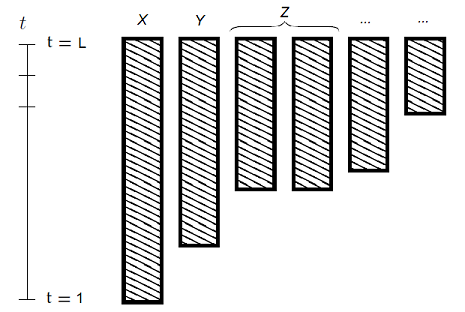Figure 2. Missing asset returns with several starting dates. Source: Gramacy et al.

In such a situation, a possible way to extend Page’s method is to apply it iteratively as proposed in Jiang and Martin9.

For this, let be $G_1,…,G_J, J \geq 1$ groups of assets whose length of returns history $L = L_1 > L_2 > … > L_J \geq 1$ differ, but which share a common returns history ending date, as illustrated in Figure 2.

Then, Page’s method can be extended as follows:

• Apply Page’s method to the long group of assets $X = G_1$ and to the short group of assets $Y = G_2$
• Once missing asset returns in the group $Y = G_2$ have been backfilled, apply Page’s method to the long group of assets $X = G_1 \cup G_2$ and to the short group of assets $Y = G_3$
• Once missing asset returns in the group $Y = G_{J-1}$ have been backfilled, apply Page’s method to the long group of assets $X = G_1 \cup G_2 \cup … \cup G_{J-1}$ and to the short group of assets $Y = G_J$

### Practical details

Some numerical subtelties need to be taken into account when implementing Page’s method, among which that:

• The covariance matrix $\Sigma_{XX,S}$ of the assets belonging to the group $X$, computed over the short returns history, might not be invertible, c.f. for example Gramacy et al.8
• The covariance matrix $\Sigma_b$ of the Gaussian distribution appearing in the conditional sampling backfilling method might not be positive semi-definite

### Caveats

Page3 highlights that his method does not magically transforms missing data into additional information3 and lists several of its limitations.

I think one of the most important of these is that3

The model assumes that betas between the existing [asset returns] and the missing [asset returns] do not change, which is not necessarily a realistic assumption.

To also be noted that even with this method, backfilling missing returns for a completely new asset class might unfortunately remain elusive.

For example, in their piece Risk Analysis of Crypto Assets, people at Two Sigma concludes that Bitcoin is not easily explained by the Two Sigma Factor Lens, nor is it substantially correlated to other currencies or any of the major commodities, so that no long returns history of any asset class seems to contain sufficient information to accurately backfill Bitcoin returns…

## Implementation in Porfolio Optimizer

Portfolio Optimizer implements the extension of Page’s method for multiple starting dates described in the previous section, together with specific care for the numerical subtelties also described in the previous section, through the endpoint /assets/returns/backfilled.

## Quality of backfilled returns

Page’s residuals recycling backfilling procedure has been designed to better account for non-normal distributions3.

To which extent is this goal reached in practice?

Let’s check.

### Theoretical asset returns distribution

Page3 uses a simulation framework in order to compare backfilled v.s. expected returns for a bivariate $t$-distribution and obtain the results displayed in Figure 3, taken from Page3.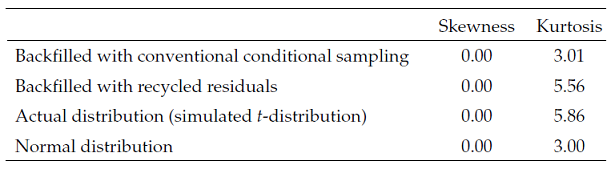Figure 3. Higher moments for backfilled simulated returns compared with known bivariate fat-tailed t-distribution. Source: Page.

Results from Figure 3 leads to the following conclusions:

• Missing returns backfilled with the conditional sampling backfilling procedure converge to a (univariate) Gaussian distribution
• Missing returns backfilled with the residuals recycling backfilling procedure seem to have a sample kurtosis very close to the sample kurtosis of the theoretical bivariate $t$-distribution

In other words, the residuals recycling backfilling procedure seems to reach its advertised goal, at least when applied to a known theoretical distribution.

### Empirical asset returns distribution

More empirically, Page3 uses monthly returns on:

in order to compare backfilled v.s. actual returns for E.M. stocks.

In more details:

• Returns on U.S. stocks from January 1988 to May 2011 (long returns history) and returns on E.M. stocks from February 1998 to May 2011 (short returns history) are used to backfill returns on E.M. stocks from January 1988 to January 199810

This process is repeated 10000 times to obtain 10000 different backfilled paths for emerging-market stocks3.

• Moments are computed on each backfilled path, and the grand average of these moments is computed over all backfilled paths

The moments of interest are the mean, the variance, the skewness and the kurtosis of backfilled returns.

• Moments are computed on E.M. stocks using actual returns data from January 1988 to January 199811

Using Portfolio Optimizer, this test can easily be reproduced12, c.f. the Jupyter notebook corresponding to this post, which gives for example the figures below:

Backfilling procedure Mean Variance Skewness Kurtosis
None (actual returns) 1.5% 0.0039 -0.25 3.83
Conditional sampling 2.2% 0.0036 -0.07 3.11
Recycled residuals 2.2% 0.0036 -0.20 3.24

These figures clearly show that the recycled residuals backfilling procedure generate asset returns that are closer, in terms of higher moments, to actual returns v.s. the conditional sampling backfilling procedure.

From this perspective, and even though the mean of backfilled returns is quite far off the mean of actual returns, the recycled residuals backfilling procedure can definitely be considered to properly recover fat tails in the missing [returns] data3.

## Conclusion

Page’s method provides a formal, plug-and-play solution3 to the problem of unequal return histories in portfolio analysis and optimization.

While other methods certainly exist, like methods based on risk factors, these other methods usually tend to be more complex, so that Page’s method is a very good choice for anyone requiring a simple way to manage missing asset returns.

For more quantitative methods with just the right level of complexity, feel free to connect with me on LinkedIn or to follow me on Twitter.

1. For instance, historical returns of Emerging Markets (E.M.) stocks are available from the late 1980s13 while historical returns of U.S. stocks are available from the late 1920s14 or even earlier.

2. Page3 note that in the context of his paper, asset returns series are not assumed to be multivariate Gaussian, so that the maximum likelihood procedure of Stambaugh actually becomes a quasi-maximum likelihood procedure.

3. To be noted that contrary to $\mu_{b_t}$ , $\Sigma_b$ is time-independant.

4. Asset returns have a tendency to follow a distribution closer and closer to a Gaussian distribution the more the time period over which they are computed increases; this empirical property is called aggregational Gaussianity, c.f. Cont15

5. To be noted that there is a typo in the heading of Table 3 in Page3, because known data is taken over January 1988 - January 1999, which is not 10 years but 20 years!

6. Returns on E.M. stocks are indeed available from the full period January 1988 to May 2011.

7. Results are not strictly identical to those of Page3, due to the random nature of the test; in addition, the skewness of actual E.M. returns is -0.26 in Page3 v.s. -0.25 here, probably due to some slight different in returns data.

8. C.f. the MSCI website

]]>
Roman R.
Simulation from a Multivariate Normal Distribution with Exact Sample Mean Vector and Sample Covariance Matrix2023-07-06T00:00:00+00:002023-07-06T00:00:00+00:00https://portfoliooptimizer.io/blog/simulation-from-a-multivariate-normal-distribution-with-exact-sample-mean-vector-and-sample-covariance-matrixIn the research report Random rotations and multivariate normal simulation1, Robert Wedderburn introduced an algorithm to simulate i.i.d. samples from a multivariate normal (Gaussian) distribution when the desired sample mean vector and sample covariance matrix are known in advance2.

Wedderburn unfortunately never had the opportunity to publish his report3 and his work was forgotten until Li4 rediscovered it nearly 20 years later.

In this short blog post, I will first describe the standard algorithm used to simulate i.i.d. samples from a multivariate normal distribution and I will then detail Wedderburn’s original algorithm as well as some of the modifications proposed by Li.

## Mathematical preliminaries

### Affine transformation of a multivariate normal distribution

A textbook result related to the multivariate normal distribution is that any linear combination of normally distributed random variables is also normally distributed.

More formally:

Property 1: Let $X$ be a n-dimensional random variable following a multivariate normal distribution $\mathcal{N} \left( \mu, \Sigma \right)$ of mean vector $\mu \in \mathbb{R}^{n}$ and of covariance matrix $\Sigma \in \mathcal{M}(\mathbb{R}^{n \times n})$. Then, any affine transformation $Z = AX + b$ with $A \in \mathcal{M}(\mathbb{R}^{n \times m})$ and $b \in \mathbb{R}^{m}$, $m \ge 1$, follows a m-dimensional multivariate normal distribution $\mathcal{N} \left( A \mu + b, A \Sigma A {}^t \right)$.

### Orthogonal matrices

An orthogonal matrix of order $n$ is a matrix $Q \in \mathcal{M}(\mathbb{R}^{n \times n})$ such that $Q {}^t Q = Q Q {}^t = \mathbb{I_n}$, with $\mathbb{I_n}$ the identity matrix of order $n$.

By extension, a rectangular orthogonal matrix is a matrix $Q \in \mathcal{M}(\mathbb{R}^{m \times n}), m \geq n$ such that $Q {}^t Q = \mathbb{I_n}$.

### Random orthogonal matrices

A random orthogonal matrix of order $n$ is a random matrix $Q \in \mathcal{M}(\mathbb{R}^{n \times n})$ distributed according to the Haar measure over the group of orthogonal matrices, c.f. Anderson et al.5.

By extension, a random rectangular orthogonal matrix is a matrix $Q \in \mathcal{M}(\mathbb{R}^{m \times n}) , m \geq n$, whose columns are, for example, the first $n$ columns of a random orthogonal matrix of order $m$, c.f. Li4.

### Helmert orthogonal matrices

An Helmert matrix of order $n$ is a square orthogonal matrix $H \in \mathcal{M}(\mathbb{R}^{n \times n})$ having a prescribed first row and a triangle of zeroes above the diagonal6.

For example, the matrix $H_n$ defined by

$H_n = \begin{pmatrix} \frac{1}{\sqrt n} &\frac{1}{\sqrt n} & \frac{1}{\sqrt n} & \dots & \frac{1}{\sqrt n} \\ \frac{1}{\sqrt 2} & -\frac{1}{\sqrt 2} & 0 & \dots & 0 \\ \frac{1}{\sqrt 6} & \frac{1}{\sqrt 6} & -\frac{2}{\sqrt 6} & \dots & 0 \\ \vdots & \vdots & \vdots & \vdots & \vdots\\ \frac{1}{\sqrt { n(n-1) }} & \frac{1}{\sqrt { n(n-1) }} & \frac{1}{\sqrt { n(n-1) }} &\dots & -\frac{n-1}{\sqrt { n(n-1) }} \end{pmatrix}$

is a Helmert matrix.

A generalized Helmert matrix of order $n$ is a square orthogonal matrix $G \in \mathcal{M}(\mathbb{R}^{n \times n})$ that can be transformed by permutations of its rows and columns and by transposition and by change of sign of rows, to a form of a [standard] Helmert matrix6.

For example, the matrix $G_n$ defined by

$G_n = \begin{pmatrix} \frac{1}{\sqrt n} &\frac{1}{\sqrt n} & \frac{1}{\sqrt n} & \dots & \frac{1}{\sqrt n} \\ -\frac{1}{\sqrt 2} & \frac{1}{\sqrt 2} & 0 & \dots & 0 \\ -\frac{1}{\sqrt 6} & -\frac{1}{\sqrt 6} & \frac{2}{\sqrt 6} & \dots & 0 \\ \vdots & \vdots & \vdots & \vdots & \vdots\\ -\frac{n-1}{\sqrt { n(n-1) }} & -\frac{1}{\sqrt { n(n-1) }} & -\frac{1}{\sqrt { n(n-1) }} &\dots & \frac{n-1}{\sqrt { n(n-1) }} \end{pmatrix}$

is a generalized Helmert matrix, obtained from the matrix $H_n$ by change of sign of rows $i=2..n$.

## Simulation from a multivariate normal distribution

Let be:

• $n$ a number of random variables7
• $\mu \in \mathbb{R}^{n}$ a vector
• $\Sigma \in \mathcal{M} \left( \mathbb{R}^{n \times n} \right)$ a positive semi-definite matrix

One of the most well known algorithm to generate $m \geq 1$ i.i.d. samples $X_1, …, X_m$ from the $n$-dimensional multivariate normal distribution $\mathcal{N}(\mu, \Sigma)$ relies on the Cholesky decomposition of the covariance matrix $\Sigma$.

### Algorithm

In details, this algorithm is as follows:

• Compute the8 Cholesky decomposition of $\Sigma$
• This gives $\Sigma = L L {}^t$, with $L \in \mathcal{M} \left( \mathbb{R}^{n \times n} \right)$ a lower triangular matrix
• Generate $m$ i.i.d. samples $Z_1,…, Z_m$ from the standard $n$-dimensional multivariate normal distribution $\mathcal{N}(0, \mathbb{I_n})$
• This is done by generating $m \times n$ i.i.d. samples $z_{11}, z_{21}, …, z_{n1}, z_{1m}, z_{2m}, …, z_{nm}$ from the standard univariate normal distribution $\mathcal{N}(0, 1)$ and re-organizing these samples in $m$ vectors of $n$ variables $Z_1 = \left( z_{11}, z_{21}, …, z_{n1} \right) {} ^t, …, Z_m = \left( z_{1m}, z_{2m}, …, z_{nm} \right) {} ^t$
• Transform the samples $Z_1,…, Z_m$ into the samples $X_1,…,X_m$ using the affine transformation $X_i = L Z_i + \mu, i = 1..m$
• From Property 1, $X_1,…,X_m$ are then $m$ i.i.d. samples from the multivariate normal distribution $\mathcal{N}(\mu, \Sigma)$

### Theoretical moments v.s. sample moments

When the previous algorithm is used to generate $m$ i.i.d. samples $X_1, …, X_m$ from the $n$-dimensional multivariate normal distribution $\mathcal{N}(\mu, \Sigma)$, the sample mean vector

$\bar{X} = \frac{1}{m} \sum_{i = 1}^m X_i$

and the (unbiased) sample covariance matrix

$Cov(X) = \frac{1}{m-1} \sum_{i = 1}^m \left(X_i - \bar{X} \right) \left(X_i - \bar{X} \right) {}^t$

will be different from their theoretical counterparts, as illustrated in Figure 1 with $\mu = \left( 0, 0 \right){}^t$, $\Sigma = \begin{bmatrix} 3 & 1 \newline 1 & 2 \end{bmatrix}$ and $m = 250$.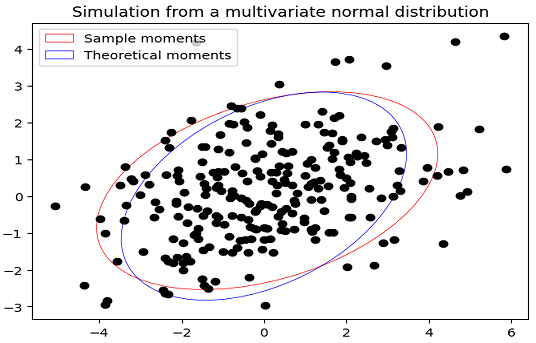Figure 1. Simulation from a multivariate normal distribution, first two sample moments v.s. first two theoretical moments.

While convergence of the first two sample moments toward the first two theoretical moments is guaranteed when $m \to +\infty$, their mismatch for finite $m$ is usually9 an issue in practical applications.

Indeed, a large number of samples is then usually required in order to reach a reasonable level of accuracy for whatever statistical estimator is being computed, and generating such a large number of samples is costly in computation time.

## Simulation from a multivariate normal distribution with exact sample mean vector and sample covariance matrix

Let be:

• $n$ a number of random variables7
• $\bar{\mu} \in \mathbb{R}^{n}$ a vector
• $\bar{\Sigma} \in \mathcal{M} \left( \mathbb{R}^{n \times n} \right)$ a positive definite matrix

Wedderburn’s algorithm1 is a conditional Monte Carlo algorithm to generate multivariate normal samples conditional on a given mean and dispersion matrix1.

In other words, given a desired sample mean vector $\bar{\mu}$ and a desired (unbiased) sample covariance matrix $\bar{\Sigma}$, Wedderburn’s algorithm allows to generate $m \geq n + 1$ i.i.d. samples $X_1, …, X_m$ from a $n$-dimensional multivariate normal distribution satisfying the two relationships

$\bar{X} = \frac{1}{m} \sum_{i = 1}^m X_i = \bar{\mu}$

and

$Cov(X) = \frac{1}{m-1} \sum_{i = 1}^m \left(X_i - \bar{X} \right) \left(X_i - \bar{X} \right) {}^t = \bar{\Sigma}$

By enforcing an exact match for finite $m$ between the first two sample moments and the first two theoretical moments of a multivariate normal distribution, Wedderburn’s algorithm allows to reduce the number of samples required in order to reach a reasonable level of accuracy for whatever statistical estimator is being computed, hence the total computation time10.

From this perspective, Wedderburn’s algorithm can be considered as a Monte Carlo variance reduction technique.

### Wedderburn’s algorithm

In details, Wedderburn’s algorithm is as follows14:

• Generate a random rectangular orthonormal matrix $P \in \mathcal{M} \left( \mathbb{R}^{(m-1) \times n} \right)$, with $m \geq n + 1$
• Compute the8 Cholesky decomposition of the matrix $\bar{\Sigma}$
• This gives $\bar{\Sigma} = L L {}^t$, with $L \in \mathcal{M} \left( \mathbb{R}^{n \times n} \right)$ a lower triangular matrix
• Define $X = \sqrt{m-1} T {}^t P L {}^t + \mathbb{1}_{m} \bar{\mu} {}^t$, with $T \in \mathcal{M} \left( \mathbb{R}^{(m-1) \times m} \right)$ made of the last $m-1$ rows of the $m \times m$ generalized Helmert matrix $G_n$ and $\mathbb{1}_{m} \in \mathbb{R}^{m}$ a vector made of ones
• The rows $X_1, …, X_m$ of $X \in \mathcal{M} \left( \mathbb{R}^{m \times n} \right)$ are then $m$ i.i.d. samples from a multivariate normal distribution whose sample mean vector is equal to $\bar{\mu}$ and whose (unbiased) sample covariance matrix is equal to $\bar{\Sigma}$

### Li’s modifications of Wedderburn’s algorithm

Li4 proposes several modifications to the original Wedderburn’s algorithm and show in particular how to manage a positive semi-definite covariance matrix $\bar{\Sigma}$.

In details, Wedderburn-Li’s algorithm is as follows4:

• Let $1 \leq r \leq n$ be the rank of the desired (unbiased) sample covariance matrix $\bar{\Sigma}$
• Generate a random rectangular orthonormal matrix $P \in \mathcal{M} \left( \mathbb{R}^{(m-1) \times r} \right)$, with $m \geq r + 1$
• Compute the8 reduced Cholesky decomposition of the matrix matrix $\bar{\Sigma}$
• This gives $\bar{\Sigma} = L L {}^t$, with $L \in \mathcal{M} \left( \mathbb{R}^{n \times r} \right)$ a “lower triangular” matrix
• Define $X = \sqrt{m-1} T {}^t P L {}^t + \mathbb{1}_{m} \bar{\mu}$, with $T \in \mathcal{M} \left( \mathbb{R}^{(m-1) \times m} \right)$ made of the last $m-1$ rows of the $m \times m$ generalized Helmert matrix $G_n$ and $\mathbb{1}_{m} \in \mathbb{R}^{m}$ a vector made of ones
• The rows $X_1, …, X_m$ of $X \in \mathcal{M} \left( \mathbb{R}^{m \times n} \right)$ are then $m$ i.i.d. samples from a multivariate normal distribution whose sample mean vector is equal to $\bar{\mu}$ and whose (unbiased) sample covariance matrix is equal to $\bar{\Sigma}$

### Misc. remarks

A couple of remarks:

• Contrary to the algorithm described in the previous section, it appears at first sight that no sample from the univariate standard normal distribution $\mathcal{N}(0, 1)$ needs to be generated when using Wedderburn’s algorithm.

This is actually not the case, because generating a random orthogonal matrix implicitely relies on the generation of such samples!

• Wedderburn1 uses the eigenvalue decomposition of the covariance matrix $\bar{\Sigma}$ instead of its Cholesky decomposition, but Li4 demonstrates that it is actually possible to use any decomposition of $\bar{\Sigma}$ such that $\bar{\Sigma} = A A {}^t$ with $A \in \mathcal{M} \left( \mathbb{R}^{n \times n} \right)$ and advocates for the usage of the Cholesky decomposition.

• As highlighted in Wedderburn1, the theoretical mean vector and covariance matrix of the multivariate normal distribution are irrelevant.

## Implementation in Portfolio Optimizer

Portfolio Optimizer implements both the standard algorithm and Wedderburn-Li’s algorithm to simulate from a multivariate normal distribution through the endpoint /assets/returns/simulation/monte-carlo/gaussian/multivariate.

To be noted, though, that for internal consistency reasons, the input covariance matrix when using Wedderburn-Li’s algorithm is assumed to be the desired biaised sample covariance matrix and not the desired unbiased sample covariance matrix.

## Conclusion

To conclude this post, a word about applications of Wedderburn’s algorithm.

These are of course numerous in finance, c.f. for example applications of similar Monte Carlo variance reduction techniques in asset pricing in Wang11 or in risk management in Meucci12.

But Wedderburn’s algorithm is more applicable in any context requiring the simulation from a multivariate normal distribution, which makes it a very interesting generic algorithm to have in one’s toolbox!

For more analysis of forgotten research reports and algorithms, feel free to connect with me on LinkedIn or to follow me on Twitter.

1. That is, the samples are simulated so that they have the desired sample mean and sample covariance matrix.

2. Because he died suddenly in 1975…

3. On this blog such variables are typically assets, but they can also be genes or species in a biology context, etc.  2

4. In case a matrix is positive definite, its Cholesky decomposition exists and is unique; in case a matrix is only positive semi-definite, its Cholesky decomposition exists but is not unique in general.  2 3

5. Not always, as variability in the sample mean and in the sample covariance matrix might be desired.

6. Under the assumption that the total time taken to generate this reduced number of samples + to compute the associated estimator is (much) lower than the total time taken to generate the initial larger number of samples + to compute the associated estimator.

]]>
Roman R.
The Bogle Model for Bonds: Predicting the Returns of Constant Maturity Government Bond ETFs2023-06-08T00:00:00+00:002023-06-08T00:00:00+00:00https://portfoliooptimizer.io/blog/the-bogle-model-for-bonds-predicting-the-returns-of-constant-maturity-government-bond-etfsIn his original 1991 article Investing in the 1990s1, John Bogle described a simple model to help investors setting reasonable expectations for long-term U.S. government bond returns.

This model relies on what Bogle describes as the single most important factor in forecasting future total returns [of a government bond], which is the the initial yield to maturity.

In this post, I will describe Bogle’s methodology and analyze its forecasting performances when applied to constant maturity U.S. government bonds, which is a category of U.S. government bonds representative of most U.S. government bond ETFs as detailled in a previous post.

## Bogle Sources of Return Model for Bonds (BSRM/B)

The Bogle Sources of Return Model for Bonds2 (BSRM/B) is a simple empirical model which states that there is but a single dominant source of decade-long returns on [government] bonds: the interest coupon2.

This model has initially been introduced by Bogle in the case of the 20-year U.S. Treasury bond1 and has then latter been shown to also be applicable in the case of the 10-year U.S. Treasury bond2.

Let’s dig into the details.

### Forecasting performances for the 20-year U.S. Treasury bond

Bogle1 examines the relationship between the initial yield to maturity of a 20-year U.S. Treasury bond and its subsequent 10-year annualized total3 return, over the period 1930 - 1980.

He notices that1:

[…] in bonds, [the initial interest rate] is the single most important factor in forecasting future total returns. The other two factors are the reinvestment rate (the rate at which the interest coupons compound), and the terminal (or end-of-period) yield.

As a matter of fact, he later shows in his paper The 1990s at the Halfway Mark4 that these three factors taken together have a correlation of 0.99 with the actual returns on bonds in each of the decades4.

Now, because the reinvestment rate and the terminal yield are by definition unknown quantities, they cannot be used to forecast future bond returns, which leaves the initial interest rate as the critical variable, [which] has a correlation of 0.709 with the returns subsequently earned by bonds.1.

In other words, the initial yield to maturity of a 20-year U.S. Treasury bond is actually sufficient to explain substantially [the bond] […] return […] over the subsequent decade4.

### Forecasting performances for the 10-year U.S. treasury bond

Bogle and Nolan2 examine the relationship between the initial yield to maturity of a 10-year U.S. Treasury bond and its subsequent 10-year annualized return over the period 1915–2014, and find that the initial interest rate explains ~90% of the variability of the subsequent 10-year annualized bond returns.

This finding is illustrated in Figure 1, directly reproduced from Bogle and Nolan2.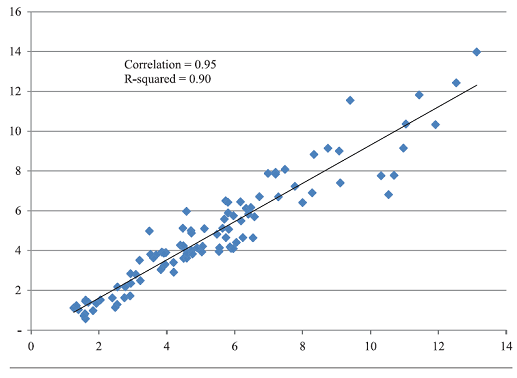Figure 1. Initial yield on the 10-year U.S. Treasury bond v.s. subsequent 10 years return, yearly data, 1915 - 2014. Source: Bogle and Nolan.

## Forecasting performances, revisited

I propose to analyze the forecasting performances of the BSRM/B model when applied to constant maturity U.S. government bonds over the out-of-sample period 31th October 1993 - 31th May 2013.

Due to the close relationship between this category of U.S. government bonds and most U.S. government bond ETFs, this will help to understand if Bogle’s model could be of any practical use to today’s investors for setting long-term capital assumptions for U.S. governement bonds.

### Out-of-sample forecasting performances for the 20-year constant maturity U.S. Treasury bond

Using the monthly 20-Year Treasury Constant Maturity Rates series from the Federal Reserve website, Figure 2 shows that the initial yield to maturity at the end of any given month over the period 31th October 1993 - 31th May 2013 explains ~72.1% of the variability of the subsequent 10-year annualized bond returns.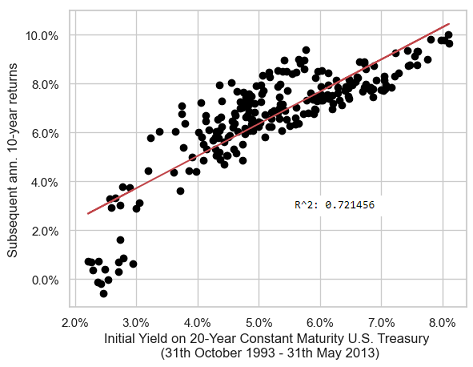Figure 2. Initial yield on the 20-year constant maturity U.S. Treasury bond v.s. subsequent 10 years return, monthly data, 31th October 1993 - 31th May 2013.

The associated monthly correlation coefficient is ~0.849, which is consistent with the yearly correlation coefficient of ~0.709 determined by Bogle1.

### Out-of-sample forecasting performances for the 10-year constant maturity U.S. Treasury bond

Using the monthly 10-Year Treasury Constant Maturity Rates series from the Federal Reserve website, Figure 3 shows that the initial yield to maturity at the end of any given month over the period 31th October 1993 - 31th May 2013 explains ~85.2% of the variability of the subsequent 10-year annualized bond returns!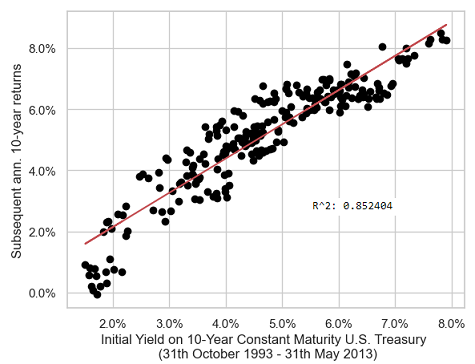Figure 3. Initial yield on the 10-year constant maturity U.S. Treasury bond v.s. subsequent 10 years return, monthly data, 31th October 1993 - 31th May 2013.

Such a value for the monthly $r^2$ coefficient is again consistent with the yearly $r^2$ coefficient of ~90% obtained by Bogle and Nolan2 and displayed in Figure 1.

### Conclusion of the out-of-sample study

The empirical conclusions of this section are that:

• The BSRM/B model is definitely applicable to 20-year and 10-year constant maturity U.S. government bonds
• The out-of-sample forecasting performances of this model are similar to its in-sample forecasting performances5 for the two considered maturities

But what about the forecasting performances of the BSRM/B model for other maturities? For example, for the 3-year constant maturity U.S. government bond, represented in Figure 4, or for the 30-year constant maturity U.S. government bond, represented in Figure 5?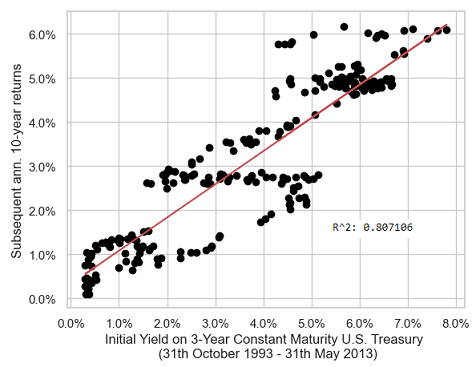Figure 4. Initial yield on the 3-year constant maturity U.S. Treasury bond v.s. subsequent 10 years return, monthly data, 31th October 1993 - 31th May 2013.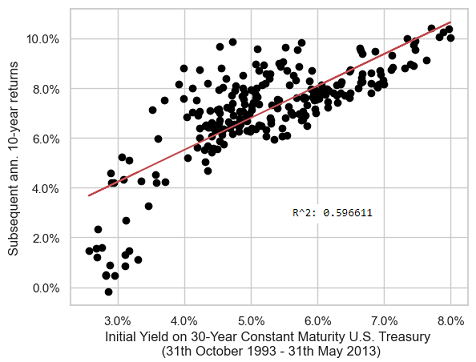Figure 5. Initial yield on the 30-year constant maturity U.S. Treasury bond v.s. subsequent 10 years return, monthly data, 31th October 1993 - 31th May 2013.

From Figure 2 to Figure 5, another empirical conclusion is that the BSRM/B model is best suited to a 10-year constant maturity U.S. government bond, because the more the deviation from a maturity of 10 years the more the degradation in forecasting performances6.

## Forecasting performances, theoretical justification

Surprinsingly, all empirical conclusions of the previous section, and especially the last one, are backed up by theoretical results.

Indeed, Leibowitz et al.7 analyze the behaviour of constant duration bond funds and establish that multi-year […] returns […] converge in both mean and volatility around the starting yield7, with a convergence horizon of about $2D - 1$ years for a bond fund whose duration is $D$ years, regardless of interim changes in yields8 which only impact this convergence by widening the distribution of returns around the mean return7.

These results allow to understand the behaviour of the BSRM/B model when applied to the 10-year constant maturity U.S. government bond9:

• From its investable counterpart, the iShares 7-10 Year Treasury Bond ETF (IEF ETF), we can assume that its (constant-ish) duration is ~7.5 years at the date of publication of this post10
• From Leibowitz et al.7, we can then conclude that the initial yield to maturity of the 10-year constant maturity U.S. government bond should be predictive of its annualized returns over the subsequent ~2*7.5 - 1 = ~14 years, which is confirmed by Figure 6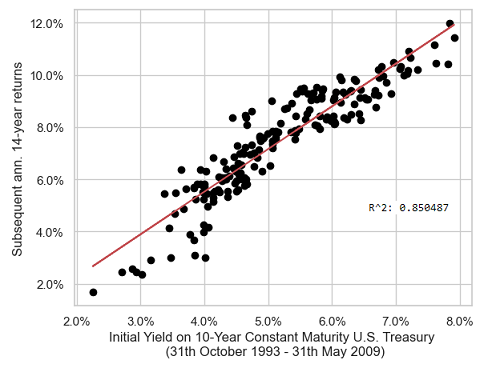Figure 6. Initial yield on the 10-year constant maturity U.S. Treasury bond v.s. subsequent 14 years return, monthly data, 31th October 1993 - 31th May 2009.
• Nevertheless, again from Leibowitz et al.7, it can happen that the convergence horizon is much shorter in practice than in theory11, and it seems that this is exactly what happens for the 10-year constant maturity U.S. Treasury bond, with an effective convergence horizon of ~10 years (as confirmed by the very good forecasting performances depicted in Figure 3) v.s. a theoretical convergence horizon of ~14 years

These results also allow to understand the behaviour of the BSRM/B model when applied to the the 3-year, 20-year and 30-year constant maturity U.S. government bonds, as the initial yield to maturity of these bonds should then NOT be predictive of their annualized returns over the subsequent 10 years, but should rather be predictive of their annualized returns over the subsequent $2 D_3 - 1$, $2 D_{20} - 1$ and $2 D_{30} - 1$ years, with $D_3$, $D_{20}$ and $D_{30}$ their respective durations12.

This theoretical behaviour is (somewhat) confirmed in practice, with for example Figure 7 illustrating that the initial yield to maturity of the 3-year constant maturity U.S. government bond is highly predictive of the annualized returns of this bond over the subsequent 3 years over the period 28th February 1962 - 31th May 2020.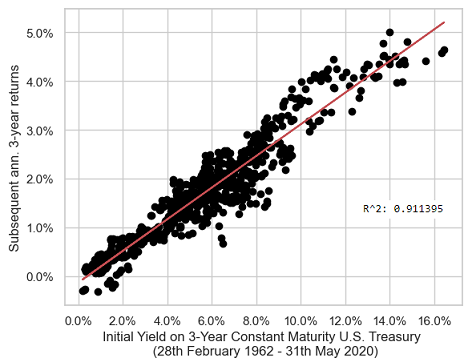Figure 7. Initial yield on the 3-year constant maturity U.S. Treasury bond v.s. subsequent 3 years return, monthly data, 28th February 1962 - 31th May 2020.

## Implementation in Portfolio Optimizer

A proprietary variation of Bogle’s BSRM/B model is implemented through the Portfolio Optimizer endpoint /markets/indicators/bsrmb/us to compute

• The initial yield of the U.S. 10-year constant maturity governement bond (i.e., the value of the BSRM/B model for this bond), over the 121 past months
• The forecasted 10-year annualized return for the U.S. 10-year constant maturity governement bond (investable asset - IEF ETF) and a 95% confidence interval around it, over the 121 future months

## Examples of usage

Typical examples of usage for the BSRM/B model are similar to the ones described in a previous blog post about a predictor of long-term stock market returns called the AIAE.

For example:

• Setting long-term capital market assumptions for U.S. government bonds

As an illustration, Figure 8 displays the “path” of expected long-term returns for the 10-year constant maturity U.S. government bond over the period 28th February 1962 - 31th May 2023.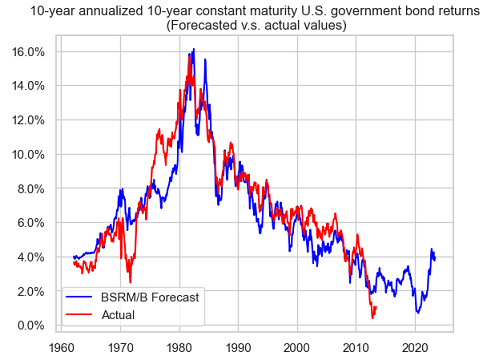Figure 8. 10-year annualized U.S. 10-year constant maturity Treasury bond returns, forecasted v.s. actual values, Portfolio Optimizer forecasts, 28th February 1962 - 31th May 2023.

From Figure 8, and at the date of publication of this post13, a buy-and-hold investment in the 10-year constant maturity U.S. government bond is expected to yield an annualized return of ~4% over the next 10 years.

• Generating future price scenarios

Here, Figure 9 displays the price scenario corresponding to Figure 8 in the case of the IEF ETF, with a 95% confidence interval added.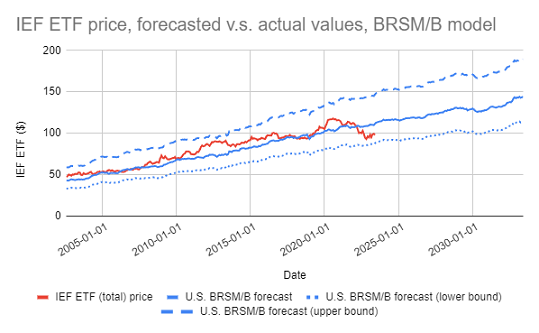Figure 9. IEF ETF price, forecasted v.s. actual values with 95% confidence interval, Portfolio Optimizer forecasts, 28th February 1962 - 31th May 2023.

A less typical example of usage would be to combine14 the forecasts produced by the BSRM/B model with estimations of the equity risk premium in order to predict future stock market returns.

A good starting place to find such estimations of the equity risk premium is the website of Aswath Damodaran, who maintains estimates of the historical implied equity risk premiums for the U.S. with plenty of details in his yearly-updated paper Equity Risk Premiums (ERP): Determinants, Estimation and Implications15.

### Conclusion

Bogel’s bond model has proved very effective at predicting long-term U.S. governement bonds returns over more than thirty years after its initial publication, which confirms what Bogle1 wrote in his original paper:

when we know the current coupon, we know most of what we need to know to forecast [government] bond returns in the coming decade

I find this model is another interesting addition to one’s forecasting toolbox on top of the AIAE indicator!

For more forecasts, feel free to connect with me on LinkedIn or to follow me on Twitter.

1. All bond returns considered in this blog post are total returns, so that I will omit “total”.

2. More data is needed to support this claim here; for example, the in-sample monthly $r^2$ coefficient for the 10-year constant maturity U.S. Treasury bond is equal to ~83.9%, so that there is no apparent degradation of the BSRM/B model.

3. This is especially visible in Figure 5, with an $r^2$ coefficient of only ~59.7%.

4. That is, whatever the pace of yield changes or the magnitude of yield changes. As a matter of fact, Leibowitz et al.7 show that what is important is the standard deviation of the yield change distribution.

5. Viewing a constant maturity bond as a constant duration bond fund might seem as a leap of faith, but the analysis of constant duration bond funds done in Leibowitz et al.7 shows that they should not differ much in practice.

6. The duration of the 10-year constant maturity U.S. Treasury bond/IEF ETF is not constant over time, so that this is a kind of first-order approximation.

7. In Leibowitz et al.7, the effective convergence horizon of a 5-year constant duration bond fund is shown to 6 years instead of 9 years.

8. Again, assumed to be constant in Leibowitz et al.7, which is not the case in practice.

9. More precisely, assuming the investment starts on 31th May 2023.

10. Of course, in a non-circular way.

]]>
Roman R.
The Single Greatest Predictor of Future Stock Market Returns, Ten Years After2023-05-22T00:00:00+00:002023-05-22T00:00:00+00:00https://portfoliooptimizer.io/blog/the-single-greatest-predictor-of-future-stock-market-returns-ten-years-afterIn his 2013 post The Single Greatest Predictor of Future Stock Market Returns, Jesse Livermore1 from the blog Philosophical Economics introduced an indicator to forecast long-term U.S. stock market returns and empirically demonstrated that it outperformed all the commonly used stock market valuation metrics like the Shiller CAPE2.

This indicator, called the Aggregate Investor Allocation to Equities (AIAE), has been further analyzed by Raymond Micaletti in his paper Towards a Better Fed Model3, with the conclusion that it indeed has superior equity-return forecasting ability compared to other well-known indicators (such as the CAPE ratio, Tobin’s Q, Market Cap-to-GDP, etc.)3.

In this post, I will describe in details the AIAE indicator, come back on nearly ten years of out-of-sample performances and show how to use the forecast procedure proposed by Micaletti3 in order to set long-term capital assumptions for the U.S. stock market.

## The AIAE indicator

### Definition

Livermore4 defines the AIAE indicator as the total amount of stocks that investors are holding in aggregate divided by the total amount of stocks plus bonds plus cash that these same investors are holding in aggregate, that is

$AIAE = \frac{TMV_s}{TMV_s + TMV_b + C}$

, where:

• $TMV_s$ is the total market value of stocks
• $TMV_b$ is the total market value of bonds
• $C$ is the total market value of cash

By definition, this indicator represents the average investor allocation to stocks5, hence its name.

### Computation of the U.S. AIAE indicator

Through some approximations, Livermore4 shows that it is possible to compute the AIAE indicator for the U.S. thanks to economic data published in the quarterly Federal Reserve release Financial Accounts of the United States - Z.1.

In details:

• The total market value of stocks $TMV_s$ is approximated by the sum of
• The market value of non-financial corporate businesses - Fred data series Nonfinancial Corporate Business; Corporate Equities; Liability, Level (NCBEILQ027S)
• The market value of financial corporate businesses - Fred data series Domestic Financial Sectors; Corporate Equities; Liability, Level (FBCELLQ027S)
• The total market value of bonds plus cash $TMV_b + C$ is approximated by the sum of the total liabilities of real economic borrowers, that is
• The liabilities of the federal government - Fred data series Federal Government; Debt Securities and Loans; Liability, Level (FGSDODNS)
• The liabilities of households and nonprofit organizations - Fred data series Households and Nonprofit Organizations; Debt Securities and Loans; Liability, Level (CMDEBT)
• The liabilities of non-financial corporate businesses - Fred data series Nonfinancial Corporate Business; Debt Securities and Loans; Liability, Level (BCNSDODNS)
• The liabilities of the rest of the world - Fred data series Rest of the World; Debt Securities and Loans; Liability, Level (DODFFSWCMI)
• The liabilities of the state and local governments - Fred data series State and Local Governments; Debt Securities and Loans; Liability, Level (SLGSDODNS)

The U.S. AIAE indicator computed using the above Fred data series is available -> here.

### Forecasting performances of the U.S. AIAE indicator

Figure 1, adapted from Livermore4, compares the value of the U.S. AIAE indicator at the end of any given quarter over the period 31th December 1951 - 30th September 20036 with the subsequent 10-year annualized S&P 500 total7 returns.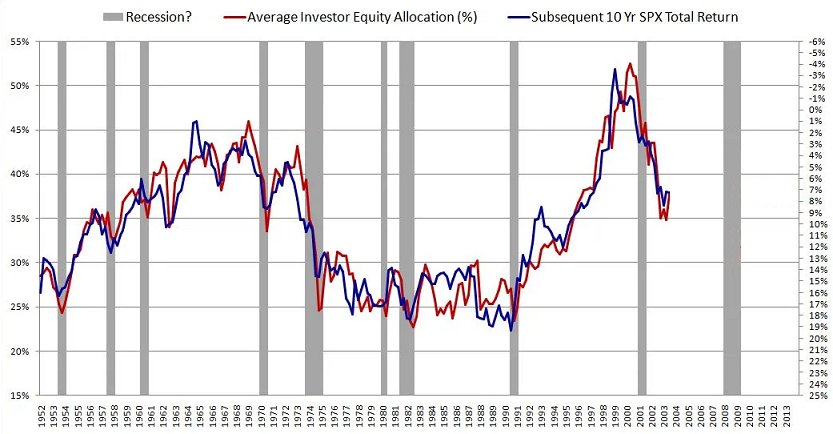Figure 1. 10-year annualized U.S. stock market returns, forecasted v.s. actual values, 31th December 1951 - 30th September 2003. Source: Livermore.

It appears that this indicator is doing an impressive job at predicting future U.S. stock market returns!

This can be confirmed more formally through an ordinary least square regression.

Figure 2, directly reproduced from Livermore4, shows that the value of the U.S. AIAE indicator at the end of any given quarter over the period 31th December 1951 - 30th September 20036 explains ~91.3% of the variability of the subsequent 10-year annualized S&P 500 returns.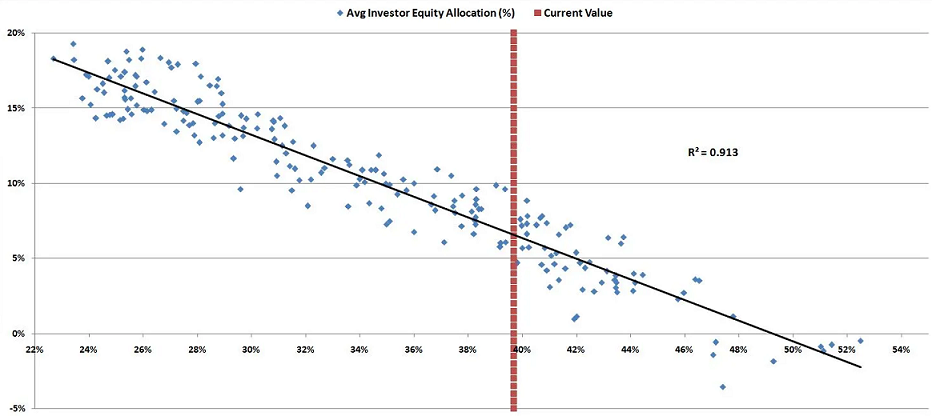Figure 2. U.S. AIAE indicator v.s. subsequent 10-year annualized S&P 500 returns, 31th December 1951 - 30th September 2003. Source: Livermore.

These compelling forecasting performances need nevertheless to be taken with a grain of salt, because they are not exactly achievable in real life due to two problems:

• First, the implicit hindsight bias associated to Figure 1 and Figure 2

This problem is discussed more in details for example in Asness et al.8 in the case of the CAPE ratio, but it suffices to say that many equity valuation indicators usually present both encouraging in-sample long-horizon [performance]8 and directionally right but weak and disappointing out-of-sample performance8.

One solution to this problem is to evaluate the forecasting performances of equity valuation indicators in a kind of walk-forward fashion.

For the U.S. AIAE indicator, this is done in Micaletti3, who conclude that

the Aggregate Investor Allocation to Equities (AIAE) has superior equity-return forecasting ability compared to other well-known indicators (such as the CAPE ratio, Tobin’s Q, Market Cap-to-GDP, etc.)

More on this in the next section.

• Second, Fred data revisions

After the initial release of economic data (unemployment, GDP, etc.), it is usual to see these data being revised a couple of weeks, months, or quarters later.

So, because the AIAE indicator is based on economic data, its value on a given past date as computed today v.s. as computed just after the initial release of the associated economic data might be different.

Hopefully, there are some hints in Micaletti3 that the impact of this problem might be neglictible in practice.

### Rationale of the U.S. AIAE indicator

Livermore4 argues that, under reasonable assumptions, long-term stock market returns must be driven by dynamics in equities supply v.s. bonds plus cash supply, dynamics that are precisely captured by the AIAE indicator.

I will not repeat his whole reasoning here9, but it shares some similarities with the reasoning of Sharpe in his paper The Arithmetic of Active Management10 in that it uses arithmetic arguments to model the behaviour of an imaginary “aggregate investor”.

## Forecasting performances of the U.S. AIAE indicator, revisited

In this section, I will study the forecasting performances of the U.S. AIAE indicator since its publication.

• Fred economic data for the period 31th December 1951 - 30th September 2013
• S&P 500 return data up to 20th December 2013

, which allowed him to analyze the 10-year forcecasting performances of the U.S. AIAE indicator over the period 31th December 1951 - 30th September 2003.

On my side, at the date of publication of this post, I have access to

• Fred economic data for the period 31th December 1951 - 31th December 2022
• S&P 500 return data up to 22th May 2023

, which allows me to analyze the 10-year forcecasting performances of the U.S. AIAE over the additional out-of-sample period 31th December 2003 - 31th March 2013.

I will use two methodologies:

• The methodology of Livermore4, which consists in computing all pairs (U.S. AIAE indicator, realized subsequent 10-year annualized U.S. stock market returns) over the period 31th December 1951 - 31th March 2013
• The methodology of Micaletti3, which consists in
• Computing all pairs (U.S. AIAE indicator, realized subsequent 10-year annualized U.S. stock market returns) over the expanding period 31th December 1951 - $t$, $t$ = 31th December 1961,…, 31th March 2003
• Fitting a linear least square regression line on these pairs
• Extracting the associated linear regression coefficients $\alpha_t$ and $\beta_t$
• Forecasting the 10-year annualized U.S. stock market return from $t + 10$ years to $t + 20$ years by the formula $\alpha_t + \beta_t AIAE_t$, where $AIAE_t$ is the value of the U.S. AIAE indicator on date $t$

### Data

The data sources for this study are the following:

To be noted that it is possible to access Alfred economic data through a well-documented Web API.

### Livermore’s methodology (in-sample forecasting performances)

#### 31th December 1951 - 30th September 2003

Figure 3 is my reproduction of Figure 2 from Livermore4.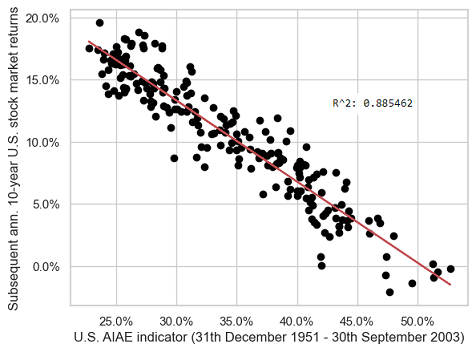Figure 3. U.S. AIAE indicator v.s. subsequent 10-year annualized U.S. stock market returns, 31th December 1951 - 30th September 2003.

Although there is a slight difference in the $r^2$ coefficients (~91.3% in Figure 2 v.s. ~88.5% in Figure 3), probably related to differences in both Fred data12 and U.S. stock market return data13, these two figures look very much alike.

This validates my reproduction of Livermore’s methodology.

#### 31th December 2003 - 31th March 2013

Figure 4 is the same as Figure 3, with the data points corresponding to the out-of-sample period 31th December 2003 - 31th March 2013 added.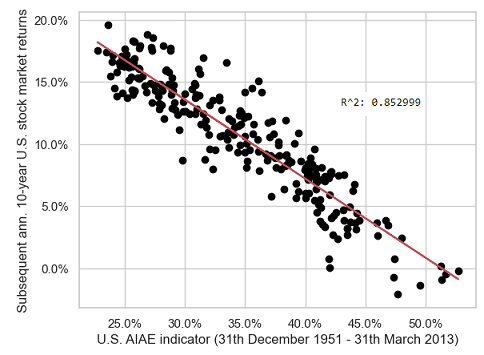Figure 4. U.S. AIAE indicator v.s. subsequent 10-year annualized U.S. stock market returns, 31th December 1951 - 31th March 2013.

Unfortunately, the $r^2$ coefficient has decreased (from ~88.5% in Figure 3 to ~85.2% in Figure 4), which implies that forecasting performances have degraded over the most recent period.

This is confirmed by Figure 5, which displays only the data points corresponding to the out-of-sample period.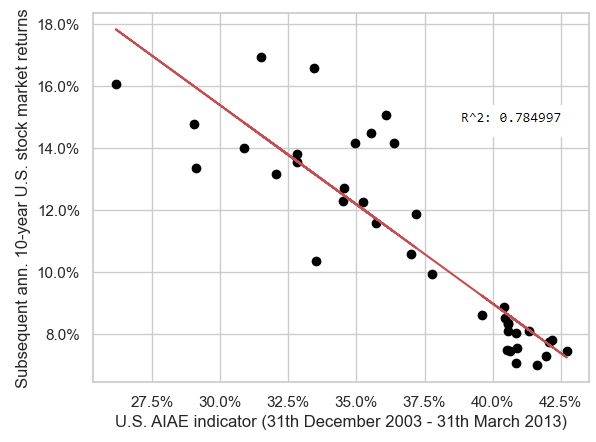Figure 5. U.S. AIAE indicator v.s. subsequent 10-year annualized U.S. stock market returns, 31th December 2003 - 31th March 2013.

On this figure, it is clearly visible that the relationship between the U.S. AIAE indicator and the subsequent 10-year annualized U.S. stock market returns is linear-ish, but with a high variability.

### Micaletti’s methodology (walk-forward forecasting performances)

#### 31th December 1951 - 30th September 2003

Figure 6 empirically demonstrates that the forecasts of the 10-year annualized U.S. stock market returns obtained using Micaletti’s methodology3 match extremely well with their actual counterparts over the period 31th December 1951 - 30th September 2003.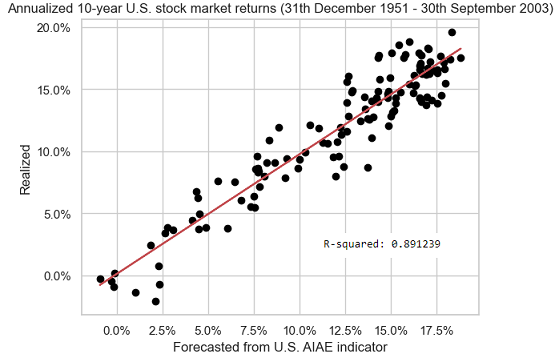Figure 6. 10-year annualized U.S. stock market returns, forecasted v.s. actual values, 31th December 1951 - 30th September 2003.

As a side note, the $r^2$ coefficient obtained with the “real-time” methodology of Micaletti (~89.1% in Figure 6) is a little bit higher than the $r^2$ coefficient obtained using the “hindsight-biased” methodology of Livermore (~88.5% in Figure 3).

This might be linked to the usage of an expanding window in Micaletti’s methodology, which allows to account for dynamics in the evolution of the linear regression coefficients, or this might more probably just be noise.

#### 31th December 2003 - 31th March 2013

Figure 7 is the same as Figure 6, with the data points corresponding to the out-of-sample period 31th December 2003 - 31th March 2013 added.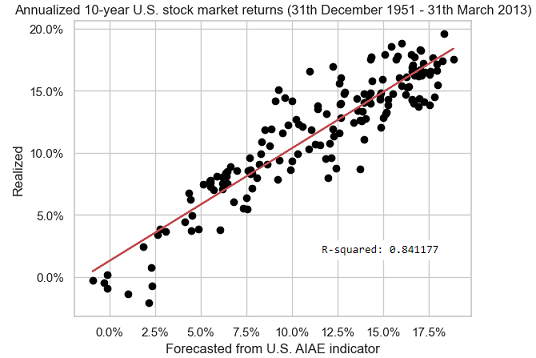Figure 7. 10-year annualized U.S. stock market returns, forecasted v.s. actual values, 31th December 1951 - 31th March 2013.

The situation here is the same as with Livermore’s methodology, that is, the $r^2$ coefficient has decreased over the most recent period (from ~89.1% in Figure 6 to ~84.1% in Figure 7).

The associated degradation in forecasting performances is confirmed in Figure 8, which displays only the data points corresponding to the out-of-sample period.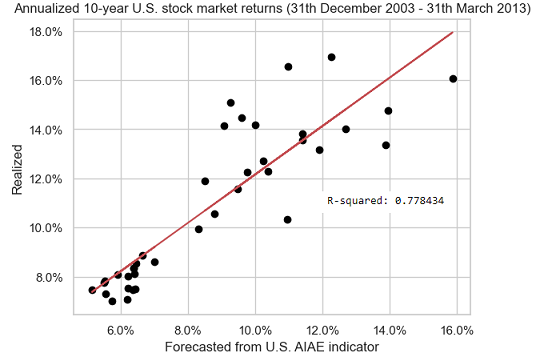Figure 8. 10-year annualized U.S. stock market returns, forecasted v.s. actual values, 31th December 2003 - 31th March 2013.

### Conclusion of the out-of-sample study

The bottom line of what precedes is that the forecasting performances of the U.S. AIAE indicator have indubitably decreased since its publication by Livermore4, which is materialized by a lower $r^2$ coefficient.

While the COVID crisis and recovery certainly played a role, it is actually not the first time in history that such a decrease in forecasting performances occurs.

Figure 9 displays the rolling $r^2$ coefficient, over a prior period of 10 years, of the 10-year annualized U.S. stock market returns forecasts v.s. actual values.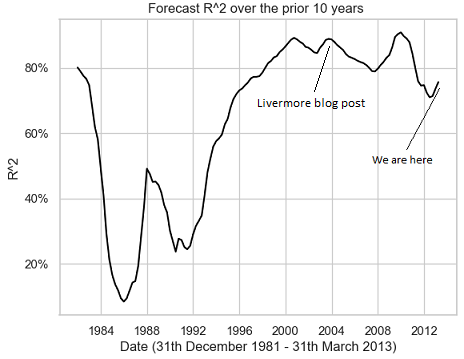Figure 9. 10-year annualized U.S. stock market returns, forecasted v.s. actual values $r^2$ coefficient over the prior 10 years, 31th December 1981 - 31th March 2013.

On this figure, there are many 10-year periods with an $r^2$ coefficient much lower than ~77.8%, with even 10-year periods whose $r^2$ coefficient is much lower than 20%!!

So, as glimmers of hope, 1) there have been much worse underperforming periods in history than the current one (relative hope) and 2) the current $r^2$ coefficient is still very high for an equity valuation indicator4 (absolute hope).

## Implementation in Portfolio Optimizer

A proprietary variation of Micaletti’s methodology3 is implemented through the Portfolio Optimizer endpoint /markets/indicators/aiae/us to compute:

• The U.S. AIAE, over the 41 past quarters
• The forecasted 10-year annualized U.S. stock market return (investable asset - SPY ETF) and a 95% confidence interval around it, over the 41 future quarters

## Examples of usage

### Setting (better) long-term capital market assumptions for the U.S. stock market

Every year, major financial institutions publish their long-term capital market assumptions based on their internal valuation models (BlackRock, J.P.Morgan…).

The U.S. AIAE indicator enables individual investors to have access to such a valuation model in the case of the U.S. stock market.

Even better, the U.S. AIAE indicator also enables individual investors to have access to the “path” of expected long-term U.S. stock market returns, as for example regularly published by Micaletti on his twitter account through images like Figure 10.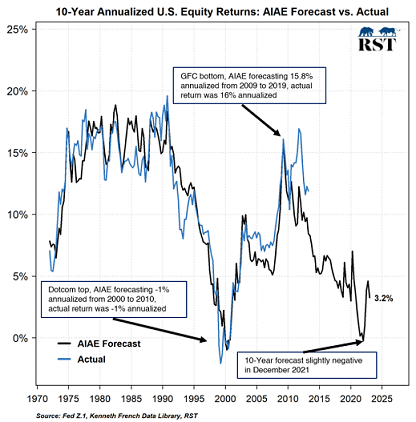Figure 10. 10-year annualized U.S. stock market returns, forecasted v.s. actual values, 31th December 1971 - ?. Source: Micaletti.

For comparison (shameless plug):

• The path equivalent to Figure 10, but generated by Portfolio Optimizer, is depicted in Figure 11.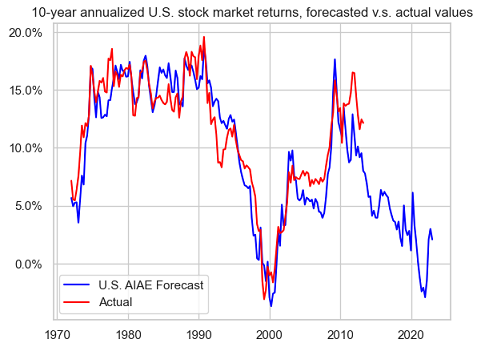Figure 11. 10-year annualized U.S. stock market returns, forecasted v.s. actual values, Portfolio Optimizer forecasts, 31th December 1971 - 31th December 2022.
• The same path as in Figure 11, with 95% confidence interval and using the SPY ETF returns, is depicted in Figure 12.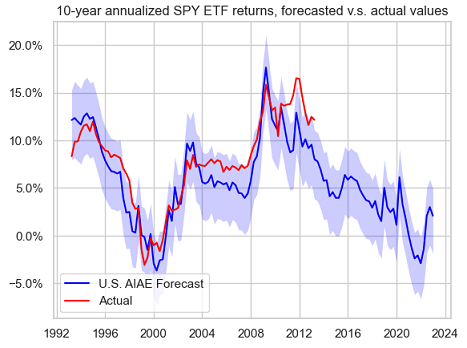Figure 12. 10-year annualized SPY ETF returns, forecasted v.s. actual values with 95% confidence interval, Portfolio Optimizer forecasts, 31th March 1993 - 31th December 2022.

Thanks to these forecasts, it becomes possible to contextualize the long-term capital market assumptions of financial institutions.

For example:

• BlackRock forecast14 a 10-year annualized U.S. stock market return from 31th December 2022 to 31th December 2032 of ~7.9%.

This forecast can be compared to the U.S. AIAE forecast of ~2.1% in Figure 12 and of not a much higher value in Figure 11.

• J.P.Morgan comment15 on December 2022 that

Lower valuations and higher yields mean that markets today offer the best potential long-term returns since 2010

This comment can be put into perspective by looking at the U.S. AIAE forecasts around 2010 in Figure 12 and Figure 11.

### Generating future price scenarios for the SPY ETF

The U.S. AIAE forecasts of long-term U.S. stock market returns can be converted into future price scenarios for misc. traded instruments.

In the case of the SPY ETF, the price scenarios corresponding to Figure 12 are represented in Figure 13.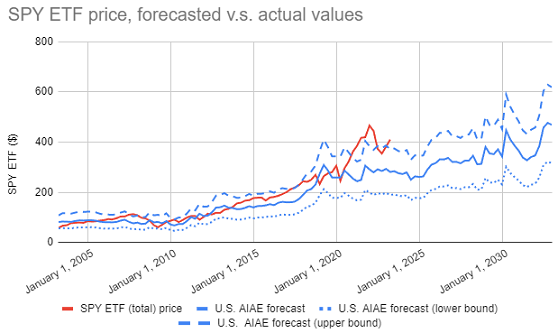Figure 13. SPY ETF price, forecasted v.s. actual values with 95% confidence interval, Portfolio Optimizer forecasts, 31th March 2003 - 31th December 2022.

Such price scenarios are sometimes more easy to grasp, or to describe to customers, than forecasts of pure returns.

Looking at Figure 13, it is for example clear that the price of the SPY ETF is currently not where it “should” be, and that, assuming the U.S. AIAE indicator continues to be reliable, we might be in for at best ~2 years of flat returns and at worst for a moderate to severe U.S. market correction anytime soon…

### Tactical asset allocation

The forecasts generated by the U.S. AIAE indicator can also be used within a tactical asset allocation framework, as detailed for example in Micaletti3, who note that:

[…] over the last 43 years and across various subperiods, the AIAE-based TAA strategy delivered the most consistently high-level performance relative to its competitors.

Alternatively, they could also be integrated in any other tactical asset allocation framework that already uses an equity valuation indicator, like the framework described in Asness et al.8.

I will not go into more details here, though.

## Conclusion

Nearly one decade of out-of-sample forecasting performances validates that the AIAE indicator introduced by Livermore4 has been very impressive in the U.S.

Now, whether the current period of (relative) underperformance will continue or stop in the future is of course open to debate, but in any cases, I hope that this blog post shed some light on this little-known equity valuation indicator.

For more uncommon quantitative nuggets, feel free to connect with me on LinkedIn or to follow me on Twitter.

1. This is a pseudonym.

2. Livermore4 makes the working assumption that an investor can only invest in stocks, bonds or cash as far as financial assets are concerned; whether the development of alternative financial asset classes like cryptocurrencies will at some point impact this assumption remains to be seen.

3. Although there is no reference in Livermore4 to the exact period used for his graphs, my best guess is 31th December 1951 - 30th September 2003.  2

4. All stock market returns considered in this blog post are total returns, so that I will omit “total”.

5. Micaletti3 includes a summary of Livermore4

6. The Alfred website is a point in time version of the Fred website, which allows to access initial releases or more generally specific point in time versions of economic data.

7. There is no reference in Livermore4 to whether the Fred data are initial releases, but my best guess is that they are not.

8. I used the stock market returns provided on the Kenneth French’s website, while Livermore4 used the S&P 500 returns.

]]>
Roman R.
The Gerber Statistic: A Robust Co-Movement Measure for Correlation Matrix Estimation2023-05-08T00:00:00+00:002023-05-08T00:00:00+00:00https://portfoliooptimizer.io/blog/the-gerber-statistic-a-robust-co-movement-measure-for-correlation-matrix-estimationThe Gerber statistic is a measure of co-movement similar in spirit to the Kendall’s Tau coefficient that has been introduced in Gerber et al.1 to estimate correlation matrices within the Markowitz’s mean-variance framework.

In this post, after providing the necessary definitions, I will reproduce the empirical study of Gerber et al.1 which highlights the superiority of the Gerber correlation matrix relative to the sample correlation matrix and I will discuss some practical aspects associated to the usage of the Gerber statistic (how to choose the Gerber threshold…).

Notes:

• A Google sheet corresponding to this post is available here

## Mathematical preliminaries

### The Gerber statistic

#### Definition

Let be two assets $i$ and $j$ observed over $T$ time periods, with:

• Returns $r_{i,t}$ and $r_{j,t}$, $t=1..T$
• (Sample) Standard deviation of returns $\sigma_i$ and $\sigma_j$

Let then be the scatter plot of the standardised joint returns $\left( \frac{r_{i,t}}{\sigma_1}, \frac{r_{j,t}}{\sigma_2} \right), t=1..T$ of these two assets, as depicted for example in Figure 1, slightly adapted from Flint and Polakow2.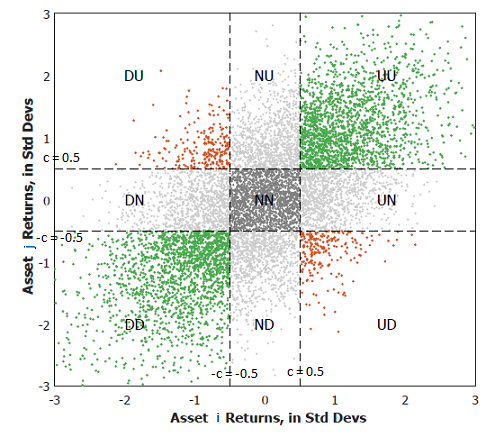Figure 1. Example of scatter plot of standardised joint returns of two assets. Source: Flint and Polakow.

By introducing the Gerber threshold $c \in [0,1]$, this scatter plot can be partitioned into nine different subsets:

• The subset $UU$, containing the standardised joint returns for which both asset returns are above $c$
• The subset $DD$, containing the standardised joint returns for which both asset returns are below $-c$
• The subset $NN$, containing the standardised joint returns for which both asset returns are above $-c$ and below $c$

The Gerber statistic $g_{i,j}$ is then defined as1

$g_{i,j} = \frac{n_{i,j}^{UU} + n_{i,j}^{DD} - n_{i,j}^{UD} -n_{i,j}^{DU}}{T - n_{i,j}^{NN}}$

, where:

• $n_{i,j}^{UU}$ (resp. $n_{i,j}^{DD}$) is the number of standardised joint returns belonging to the subset $UU$ (resp. $DD$), which are termed concordant pairs of returns3 and are coloured in green in Figure 1
• $n_{i,j}^{UD}$ (resp. $n_{i,j}^{DU}$) is the number of standardised joint returns belonging to the subset $UD$ (resp. $DU$), which are termed discordant pairs of returns3 and are coloured in red in Figure 1
• $n_{i,j}^{NN}$ is the number of standardised joint returns belonging to the subset $NN$, which are considered as noise and are coloured in grey in Figure 1

#### Interpretation

The definition of the Gerber statistic makes it a measure of co-movement more robust to outliers and to noise than the
Pearson correlation coefficient.

Indeed, as highlighted in Gerber et al.1:

• The Gerber statistic is insensitive to extreme[ly large or small] movements that distort [the Peason correlation coefficient]1, because the magnitude of asset returns above or below the Gerber threshold $c$ is not taken into account
• The Gerber statistic is insentitive to small movements that may simply be noise1, because a Gerber threshold of at least $c = 0.5$ is advocated in practice

From this perspective, the Gerber statistic is especially well suited for financial time series, which often exhibit extreme movements and a great amount of noise1.

#### Alternative definitions

Flint and Polakow2 reference three existing variations of the Gerber statistic and note that these alternative definitions materially change[] the resultant measure, to the point that the three GS variants in press should arguably be viewed as entirely separate dependence measures2.

For the sake of clarity, this blog post will only discuss the variant termed the Gerber statistic in Gerber et al.1.

#### Example of computation

Gerber et al.1 illustrate the computation of the Gerber statistic using the $T = 24$ monthly returns4 of the asset pair S&P 500 (SPX) - Gold (XAU) over the period January 2019 - December 2020.

I propose to re-use the same example and to validate the computation thanks to the SPX - XAU returns available in the Google sheet associated to this post.

Figure 2 represents the scatter plot of the standardised joint returns of these two assets overlaid with the nine subsets corresponding to a Gerber threshold of 0.5.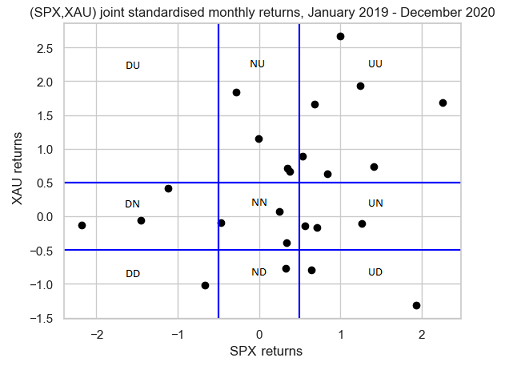Figure 2. Scatter plot of standardised joint returns of SPX - XAU partitioned into nine subsets by a Gerber threshold equal to 0.5, January 2019 - December 2020.

Figure 2 is exactly the same as the figure in panel A of exhibit A2 of Gerber et al.1, so that the computation of the Gerber statistic should result in a value of ~0.286.

Let’s double check this.

From Figure 2, we have:

• $n_{SPX,XAU}^{UU} = 7$, $n_{SPX,XAU}^{DD} = 1$
• $n_{SPX,XAU}^{UD} = 0$, $n_{SPX,XAU}^{DU} = 2$
• $n_{SPX,XAU}^{NN} = 3$

So that:

$g_{SPX,XAU} = \frac{7 + 1 - 0 - 2}{24 - 3} = \frac{2}{7} \approx 0.286$

All good!

### The Gerber correlation matrix

Let be:

• $n$, the number of assets in a universe of assets
• $r_{i,1},…,r_{i,T}$, the return of the asset $i=1..n$ over each time period $t=1..T$
• $c \in [0,1]$, the Gerber threshold

The asset Gerber correlation matrix $G \in \mathcal{M}(\mathbb{R}^{n \times n})$, also called the Gerber matrix, is then defined by:

$G_{i,j} = g_{i,j}, i=1..n, j=1..n$

, where $g_{i,j}$ is the Gerber statistic between asset $i$ and asset $j$.

### The Gerber covariance matrix

Let be:

• $n$, the number of assets in a universe of assets
• $r_{i,1},…,r_{i,T}$, the return of the asset $i=1..n$ over each time period $t=1..T$
• $\sigma_1,…,\sigma_n$, the asset standard deviations (i.e., volatilities)
• $c \in [0,1]$, the Gerber threshold

The asset Gerber covariance matrix $\Sigma_G \in \mathcal{M}(\mathbb{R}^{n \times n})$ is then defined by:

$\left( \Sigma_{G} \right)_{i,j} = g_{i,j} \, \sigma_i \, \sigma_j, i=1..n, j=1..n$

, where $g_{i,j}$ is the Gerber statistic between asset $i$ and asset $j$.

## Implementation in Portfolio Optimizer

Portfolio Optimizer implements two endpoints related to the Gerber statistic:

## Empirical performances

Gerber et al.1 analyze the empirical performance of the Gerber covariance matrix within the Markowitz’s mean-variance framework.

For this, they consider a universe of nine asset classes:

• US large cap stocks, represented by the S&P 500 index
• US small cap stocks, represented by the Russell 2000 index
• Developed markets ex. US and Canada large and mid cap stocks, represented by the MSCI EAFE index
• Emerging markets large and mid cap stocks, represented by the MSCI Emerging Markets index
• US treasuries, government-related and corporate bonds, represented by the Bloomberg Barclays US Aggregate Bond index
• US high yield corporate bonds, represented by the Bloomberg Barclays US Corporate High Yield Bond index
• US real estate, represented by the FTSE NAREIT all equity REITS index
• Gold
• Commodities, represented by the S&P GSCI Goldman Sachs Commodity index

, inside which they backtest the following portfolio investment strategy over the period January 1988 - December 2020:

1. At the end of each month, compute mean-variance input estimates over the past 24 months of returns
• The average return vector $\mu = \left( \mu_{SPX},…,\mu_{SPGSCI} \right)$
• The sample covariance matrix $\Sigma$
• The Ledoit-Wolf shrunk covariance matrix $\Sigma_{LW}$5
• The Gerber covariance matrix $\Sigma_{G}$
2. Then, compute no-short-sales constrained mean-variance efficient portfolios6 with an annualized volatility target of 3%, 5%, …, 15%
• Using $\mu$ and $\Sigma$
• Using $\mu$ and $\Sigma_{LW}$
• Using $\mu$ and $\Sigma_{G}$

Figure 3, reproduced from Gerber et al.1, illustrates the resulting three ex post mean-variance efficient frontiers in the case of a Gerber threshold equal to 0.5.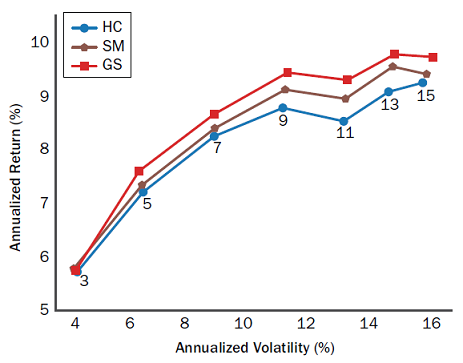Figure 3. Nine-asset universe, ex post mean-variance efficient frontiers with a Gerber threshold equal to 0.5, January 1990 - December 2020. Source: Gerber et al.

On this figure, it is pretty clear that the efficient frontier corresponding to the Gerber covariance matrix dominates the two other efficient frontiers7.

Based on these empirical findings, using the Gerber covariance matrix as an alternative to both [the sample covariance matrix] and to the shrinkage estimator of Ledoit and Wolf1 seems really compelling.

Nevertheless, some practicalities must be discussed first.

## Practical considerations

### Robustness of the empirical study of Gerber et al.1

In order to determine whether the empirical performances reported in Gerber et al.1 are robust to slight changes in implementation details8, I propose to reproduce the backest of the portfolio investment strategy detailled in the previous section using Portfolio Optimizer9.

To be noted that because Portfolio Optimizer does not support Ledoit-Wolf type shrinkage at the date of publication of this post10, I am only able to compare the Gerber covariance matrix with the historical covariance matrix.

The two reproduced ex post mean-variance efficient frontiers are displayed in Figure 4 in the case of a Gerber threshold equal to 0.5.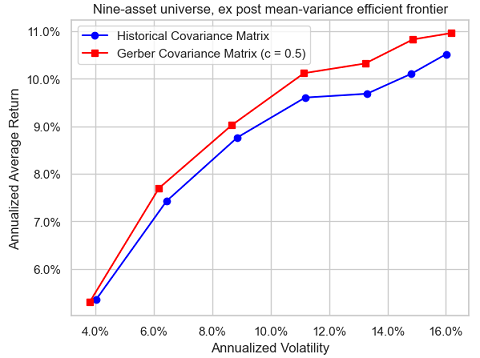Figure 4. Reproduced nine-asset universe, ex post mean-variance efficient frontiers with a Gerber threshold equal to 0.5, January 1990 - December 2020.

Figure 3 and Figure 4 are pretty close11, except really for the mean-variance efficient portfolio with an annualized volatility target of 11%, which confirms the robustness in terms of reproducibility of the empirical study of Gerber et al.1.

### Influence of the Gerber threshold

The Gerber statistic, the Gerber correlation matrix and the Gerber covariance matrix all depend on the Gerber threshold, so that it is important to understand the impact of varying the Gerber threshold on these quantities.

In the case of two assets, this impact is extensively studied in Flint and Polakow2 thanks to numerical simulations of joint normal and joint non-normal return distributions. Their conclusion is that the dependency of the Gerber statistic on the Gerber threshold is highly non trivial…

On my (less ambitious) side, I will study the impact of varying the Gerber threshold from 0 to 1 in increments of 0.1 on the backest of the portfolio investment strategy detailled in the previous section.

Figure 5 (resp. Figure 6) illustrates the evolution of the resulting portfolio investment strategy equity curves for an ex ante annualized volatility target of 5% (resp. 10%).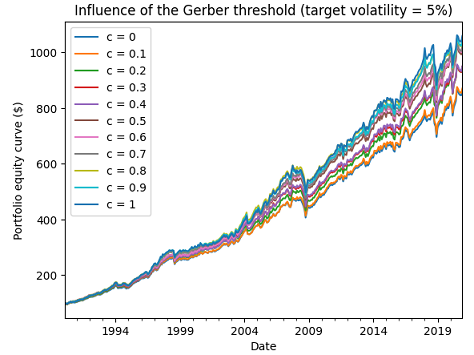Figure 5. Reproduced nine-asset universe, influence of the Gerber threshold, ex ante annualized volatility target of 5%, January 1990 - December 2020.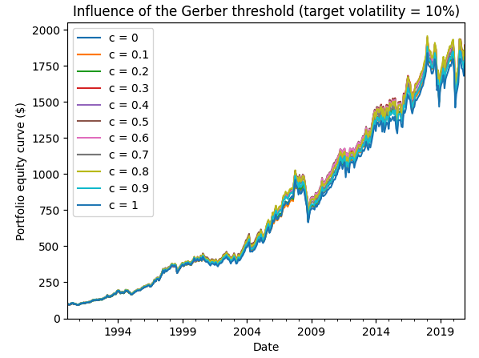Figure 6. Reproduced nine-asset universe, influence of the Gerber threshold, ex ante annualized volatility target of 10%, January 1990 - December 2020.

Figure 6 shows that the influence of the Gerber threshold on performances can be neglictible, which is very good news.

Unfortunately, Figure 5 shows on the contrary that the influence of the Gerber threshold on performances can be non-neglictible at all, which is bad news.

This leads to the question of how to “best” chose the Gerber threshold in practice.

### How to choose the Gerber threshold?

From the definition of the Gerber statistic, the higher the Gerber threshold:

• The smaller the size of the “signal” subsets $UU$, $DD$, $UD$ and $DU$
• The greater the size of the “noise” subset $NN$

As a consequence, it would make sense to choose the Gerber threshold dynamically, as a function of the “signal-to-noise ratio” of the considered universe of assets.

In the context of the portfolio investment strategy detailled in the previous section, I experimented with a simple approach based on past risk-adjusted performances:

1. At the end of each month, compute mean-variance input estimates over the past 24 months of returns
• The average return vector $\mu = \left( \mu_{SPX},…,\mu_{SPGSCI} \right)$
2. Then, for each annualized volatility target $v\%$
• Compute the Gerber threshold $c^*$ which maximizes the Sharpe ratio of the associated portfolio investment strategy over the past 24 months12 of returns
• Compute the Gerber covariance matrix $\Sigma_{G^*}$ using the Gerber threshold $c^*$
• Compute the no-short-sales constrained mean-variance efficient portfolio with an annualized volatility target of $v\%$, using $\mu$ and $\Sigma_{G^*}$

Figure 7 illustrates the evolution of the resulting portfolio investment strategy equity curve for an ex ante annualized volatility target of 5%.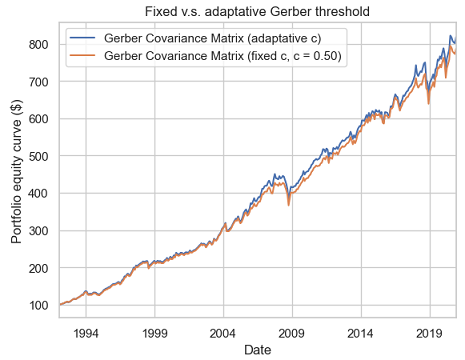Figure 7. Reproduced nine-asset universe, fixed v.s. adaptative Gerber threshold, ex ante annualized volatility target of 5%, January 1992 - December 2020.

From Figure 7, it appears that this simple data-driven method to choose the Gerber threshold is able to match the performances of the best Gerber threshold chosen in hindsight13 ($c = 0.5$).

Some (annualized) statistics to support this observation:

Gerber portfolio (fixed c = 0.50) Gerber portfolio (adaptative c)
Average return 7.6% 7.9%
Volatility 6.2% 6.2%
Sharpe ratio 1.26 1.23

Of course, no generic conclusion can be drawn from this example, but I do think that the adaptative computation of the Gerber threshold would be an interesting research topic.

### Positive semidefinitess of the Gerber correlation matrix

Gerber et al.1 mention that

In the empirical studies performed, and for all cases of Gerber thresholds $c$ considered, we always observe the […] Gerber matrix G to be positive semidefinite

, but give no formal proof that the Gerber correlation matrix is positive semidefinite in general.

It is thus natural to wonder whether this is the case, all the more because positive semidefinitess is usually lacking in other correlation matrices built from robust pairwise scatter estimates1415.

Hopefully, the Gerber correlation matrix is indeed positive semidefinite, as established in the paper Proofs that the Gerber Statistic is Positive Semidefinite from Gerber et al.16

Note:

• The initial version of this post stated that there were no proof that the Gerber correlation matrix was a positive semidefinite matrix; this was incorrect, as a proof was available on Mr Enrst website.

### Usage of the Gerber statistic beyond mean-variance

In their paper, Gerber et al.1 confine [their] analysis to the mean–variance optimization (MVO) framework of Markowitz.

What about other portfolio allocation frameworks, though?

Would the Gerber statistic be somewhat taylored to the mean-variance framework, for example because of a hidden relationship with quadratic utility?

To answer this question, I propose to adapt the portfolio investment strategy detailled inthe previous section to the risk parity framework, and more precisely to the equal risk contributions framework17, as follows:

1. At the end of each month, compute risk parity input estimates over the past 24 months of returns
• The sample covariance matrix $\Sigma$
• The Gerber covariance matrix $\Sigma_{G}$
2. Then, compute the no-short-sales constrained equal risk contributions portfolio
• Using $\Sigma$
• Using $\Sigma_{G}$

Figure 8 illustrates the resulting portfolio investment strategy equity curves in the case of a Gerber threshold equal to 0.5.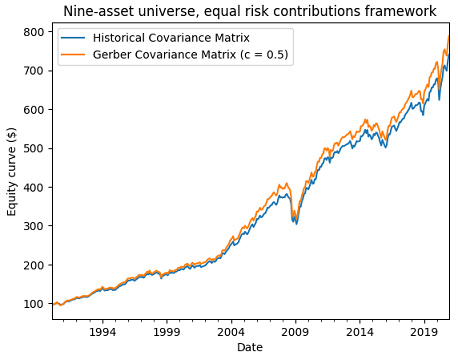Figure 8. Reproduced nine-asset universe, equal risk contributions with a Gerber threshold equal to 0.5, January 1990 - December 2020.

Figure 8 also empirically confirms that the Gerber covariance matrix also behaves properly in a non mean–variance framework.

## Caveats

### Non-uniform domination of the Gerber covariance matrix

Figure 3 and Figure 4 might give the wrong impression that the Gerber covariance matrix always dominates the sample covariance matrix in terms of ex post risk-return within the Markowitz’s mean-variance framework18.

In order to illustrate that this is not the case, I will backtest the same portfolio investment strategy as detailled in one of the previous sections, but this time with the ten-asset universe of the Adaptative Asset Allocation strategy1920 from ReSolve Asset Management:

• U.S. stocks (SPY ETF)
• European stocks (EZU ETF)
• Japanese stocks (EWJ ETF)
• Emerging markets stocks (EEM ETF)
• U.S. REITs (VNQ ETF)
• International REITs (RWX ETF)
• U.S. 7-10 year Treasuries (IEF ETF)
• U.S. 20+ year Treasuries (TLT ETF)
• Commodities (DBC ETF)
• Gold (GLD ETF)a universe of nine asset classes:

This universe is very similar to the nine-asset universe used in Gerber et al.1, because it is also well-diversified in terms of asset classes.

Unfortunately, with this universe, the ex post efficient frontier corresponding to the Gerber covariance matrix does not always dominate anymore the ex post efficient frontier corresponding to the sample covariance matrix, as can be seen in Figure 9, Figure 10 and Figure 11.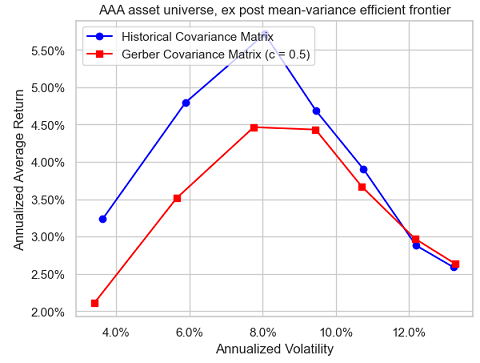Figure 9. AAA universe, ex post mean-variance efficient frontiers with a Gerber threshold equal to 0.5, January 2007 - April 2023.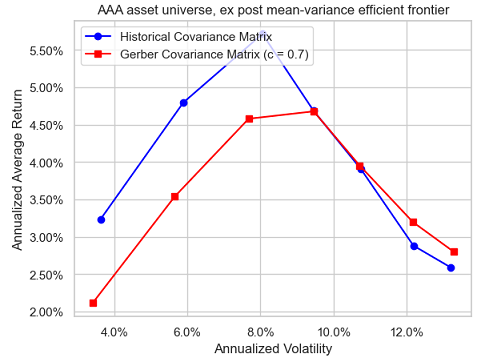Figure 10. AAA universe, ex post mean-variance efficient frontiers with a Gerber threshold equal to 0.7, January 2007 - April 2023.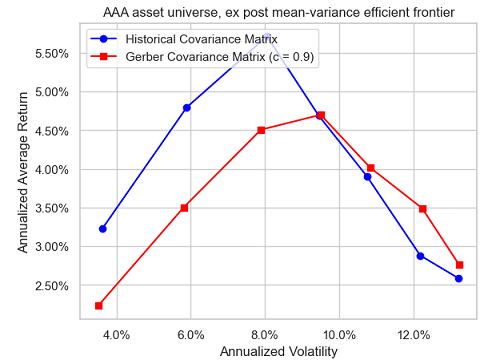Figure 11. AAA universe, ex post mean-variance efficient frontiers with a Gerber threshold equal to 0.9, January 2007 - April 2023.

### Influence of the number of observations

Because of its definition, the Gerber statistic must intuitively be more sensitive than the sample covariance matrix to measurement error21.

Still, in my own testing, I did not notice any excessive sensitivity22.

For example, Figure 12 is the equivalent of Figure 9 when daily asset returns are used instead of monthly returns.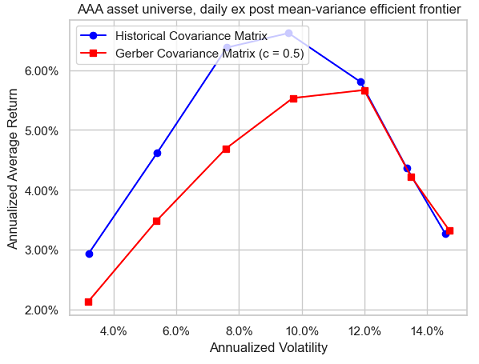Figure 12. AAA universe, ex post mean-variance efficient frontiers with a Gerber threshold equal to 0.5, January 2007 - April 2023, daily returns.

Comparing these two figures, it is hard to conclude that the Gerber covariance matrix is dramatically more sensitive to the number of observations than the sample covariance matrix23.

That being said, Flint and Polakow2 investigate the sensitivity of the Gerber statistic to estimation error more rigourously, and do find that there is considerable variation in the GS when estimated with limited observations2.

So, better to err on the side of caution here.

## Conclusion

I hope that thanks to this post you now have a good overview of the Gerber statistic, along with some of the practical concerns associated with its usage.

As Flint and Polakow2 put it:

Overall, the GS is an interesting conditional dependence metric, but not without its flaws or caveats.

If you have any questions, or if you would like to discuss further, feel free to connect with me on LinkedIn or to follow me on Twitter.

1. More precisely, Gerber et al.1 define a concordant pair of returns as a pair which both components pierce their thresholds while moving in the same direction and a discordant pair of returns as a pair whose components pierce their thresholds while moving in opposite directions 2

2. All asset returns considered in this blog post are total returns.

3. The exact implementation details used by Gerber et al.1 can be found in the Python code associated to their paper; one important detail to note is that when there is no mean-variance efficient portfolio with the desired volatility, the minimum variance portfolio or the maximum return portfolio is used instead.

4. The same conclusion applies for the two other values of the Gerber threshold, c.f. Gerber et al.1

5. In particular, when there is no mean-variance efficient portfolio with a desired volatility because the desired volatility is too low, it might be more in line with the mean-variance framework to use a partially invested portfolio v.s. the minimum variance portfolio as in Gerber et al.1

6. I would like to thank Mr William Smyth24 for providing me returns data for the nine-asset universe.

7. It’s definitely on the to do list, though.

8. In addition to the difference in managing the portfolio volatility constraint8, there are other subtle differences in my reproduction of the backtest of Gerber et al.1; for example, I do not consider any transaction cost, I use the arithmetic average return of indexes and not their geometric average return, etc.

9. The performances of the method seem to be robust w.r.t. the lookback period; to be noted that a lookback period of 12 months results in the best performances, but I chose 24 months to be consistent with the lookback period used to compute mean-variance input estimates.

10. In terms of the Sharpe ratio of the resulting portfolio investment strategy, the best Gerber threshold among the thresholds displayed in Figure 5 is equal to 0.5.

11. Which, to be clear, is not at all the conclusion of Gerber et al.1

12. The associated returns data have been retrieved using Tiingo

13. In this context, the measurement error is due to the short length of the time series of asset returns that are typically used for covariance matrix estimation.

14. To be noted that I only used the Gerber statistic with well diversified universes of assets and not with let’s say a universe of stocks (S&P 500…).

15. Or at the very least, if it really is, this does not translate into dramatically different risk-return performances, which is what ultimately matters from a portfolio management perspective.

]]>
Roman R.
Corrected Cornish-Fisher Expansion: Improving the Accuracy of Modified Value-at-Risk2023-04-13T00:00:00+00:002023-04-13T00:00:00+00:00https://portfoliooptimizer.io/blog/corrected-cornish-fisher-expansion-improving-the-accuracy-of-modified-value-at-riskModified Value-at-Risk (mVaR) is a parametric approach to computing Value-at-Risk introduced by Zangari1 that adjusts Gaussian Value-at-Risk for asymmetry and fat tails present in financial asset returns2 through a mathematical technique called Cornish–Fisher expansion.

Since its publication, mVaR has been widely adopted by academic researchers, financial regulators3 and practitioners, who typically highlight its straightforward numerical implementation and its ease of interpretation thanks to its explicit form4.

Nevertheless, it has been observed in practice that mVaR only works well for non-normal distributions that are close to the Gaussian distribution and for tail probabilities which are not too small5.

In this post, I will explain why in the light of the results of Maillard6 and Lamb et al.7, who show that mVaR accuracy is related to the mathematics of the Cornish-Fisher expansion.

I will also empirically demonstrate, using Bitcoin and the SPY ETF, that the method proposed by Maillard6 to improve mVaR accuracy makes it usable for moderately to highly non-normal distributions as well as for small tail probabilities8.

## Mathematical preliminaries

### Value-at-Risk

The (percentage) Value-at-Risk (VaR) of a portfolio of financial assets corresponds to the percentage of portfolio wealth that can be lost over a certain time horizon and with a certain probability9.

More formally, the Value-at-Risk $VaR_{\alpha}$ of a portfolio over a time horizon $T$ (1 day, 10 days…) and at a confidence level $\alpha$% $\in ]0,1[$ (95%, 97.5%, 99%…) can be defined5 as the opposite of the lower $1 - \alpha$ quantile of the portfolio return10 distribution over the time horizon $T$

$\text{VaR}_{\alpha} (X) = - \inf_{x} \left\{x \in \mathbb{R}, P(X \leq x) \geq 1 - \alpha \right\}$

, where $X$ is a random variable representing the portfolio return over the time horizon $T$.

This formula is also equivalent11 to

$\text{VaR}_{\alpha} (X) = - F_X^{-1}(1 - \alpha)$

, where $F_X^{-1}$ is the inverse cumulative distribution function, also called the quantile function, of the random variable $X$.

### Gaussian Value-at-Risk

The previous definition of VaR is not directly usable, because it requires to specify the portfolio return distribution.

One possible approach is to approximate the portfolio return distribution by its empirical distribution, in which case the associated VaR is called historical Value-at-Risk (HVaR).

Another possible approach is to approximate the portfolio return distribution by a given probability distribution, in which case the associated VaR is called parametric Value-at-Risk.

When this distribution is chosen to be the Gaussian distribution $\mathcal{N}_{\mu, \sigma^2}$, that is, when $X \sim \mathcal{N} \left( \mu, \sigma^2 \right)$ with $\mu$ the location parameter and $\sigma$ the scale parameter, the associated VaR is called Gaussian Value-at-Risk (GVaR) and is computed through the formula12

$\text{GVaR}_{\alpha} (X) = - \mu - \sigma z_{1 - \alpha}$

, where:

• The location parameter $\mu$ and the scale parameter $\sigma$ are usually2 estimated by their sample counterparts computed from past portfolio returns
• $z_{1 - \alpha}$ is the $1 - \alpha$ quantile of the standard normal distribution

### Modified Value-at-Risk

Approximating a portfolio return distribution by a Gaussian distribution might be appropriate in some cases, depending on the assets present in the portfolio and on the time horizon13, but generally speaking, financial assets exhibit skewed and fat-tailed return distributions2, so that it makes more sense to also consider higher moments than just the first two.

For this reason, Zangari1 proposed to approximate the $1 - \alpha$ quantile of the portfolio return distribution by a fourth order Cornish–Fisher expansion of the $1 - \alpha$ quantile of the standard normal distribution, which allows to take into account skewness and kurtosis present in the portfolio return distribution.

The resulting VaR, called modified Value-at-Risk or sometimes Cornish-Fisher Value-at-Risk (CFVaR), is computed through the formula12

$\text{mVaR}_{\alpha} (X) = - \mu - \sigma \left[ z_{1-\alpha} + (z_{1-\alpha}^2 - 1) \frac{\kappa}{6} + (z_{1-\alpha}^3-3z_{1-\alpha}) \frac{\gamma}{24} -(2z_{1-\alpha}^3-5z_{1-\alpha})\frac{\kappa^2 }{36} \right]$

, where the location parameter $\mu$, the scale parameter $\sigma$, the skewness parameter $\kappa$ and the excess kurtosis parameter $\gamma$ are usually2 estimated by their sample counterparts computed from past portfolio returns

To be noted that using this formula to compute VaR is equivalent to making the assumption that the portfolio return distribution follows what could be called a Cornish-Fisher distribution7 $\mathcal{CF}_{\mu, \sigma, \kappa, \gamma}$, whose inverse cumulative distribution function is given by

$F_X^{-1}(u) = \mu + \sigma \left[ z_u + (z_u^2 - 1) \frac{\kappa}{6} + (z_u^3-3z_u) \frac{\gamma}{24} -(2z_u^3-5z_u)\frac{\kappa^2}{36} \right]$

, where:

• $\mu$ is a location parameter
• $\sigma$ is a scale parameter
• $\kappa$ is a skewness parameter
• $\gamma$ is an excess kurtosis parameter
• $u \in ]0,1[$
• $z_u = \Phi^{-1}(u)$, with $\Phi$ the standard normal distribution function

, which is also equivalent7 to making the assumption that

$X \sim \mu + \sigma \left[ Z + (Z^2 - 1) \frac{\kappa}{6} + (Z^3-3Z) \frac{\gamma}{24} -(2Z^3-5Z)\frac{\kappa^2}{36} \right]$

, where:

• $\mu$ is a location parameter
• $\sigma$ is a scale parameter
• $\kappa$ is a skewness parameter
• $\gamma$ is an excess kurtosis parameter
• $Z$ is a standard normal random variable, i.e. $Z \sim \mathcal{N} \left( 0, 1 \right)$

## The lack of accuracy of modified Value-at-Risk

### Illustration

Figure 1 compares, over the period 01 February 1993 - 04 April 2023, the empirical distribution of the SPY ETF daily returns14 to the Cornish-Fisher distribution $\mathcal{CF}_{\mu_s, \sigma_s, \kappa_s, \gamma_s}$ with parameters:

• $\mu_s \approx 0.000367$, the sample mean of the SPY ETF returns over the considered period
• $\sigma_s \approx 0.011921$, the sample standard deviation of the SPY ETF returns over the considered period
• $\kappa_s \approx -0.287409$, the sample skewness of the SPY ETF returns over the considered period
• $\gamma_s \approx 10.898897$, the sample excess kurtosis of the SPY ETF returns over the considered period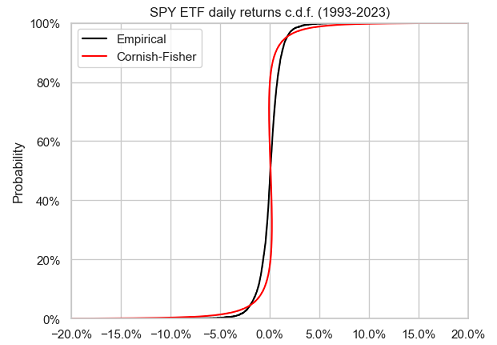Figure 1. SPY ETF daily returns, empirical c.d.f. v.s. Cornish-Fisher c.d.f., 1993-2023.

On this figure, it is visible that the Cornish-Fisher distribution does not accurately approximate the empirical distribution of the SPY ETF returns.

The same also applies to the left tail of the empirical distribution of the SPY ETF returns, as can be seen in Figure 2.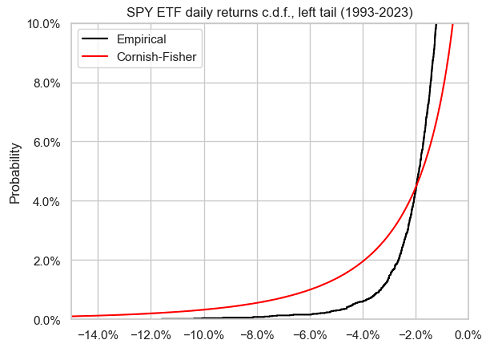Figure 2. SPY ETF daily returns, empirical c.d.f. v.s. Cornish-Fisher c.d.f., left tail, 1993-2023.

On top of this poor approximation accuracy, and maybe even worse, taking a closer look at Figure 1 also reveals that the Cornish-Fisher distribution does not seem to be monotonous. For example, quantiles between 20% and 40% are positive while quantiles between 60% and 80% are negative! This means that the Cornish-Fisher distribution is not a proper probability distribution15.

What could explain these observations, while the Cornish-Fisher expansion is supposed, by construction, to be able to approximate the quantiles of any distribution?

Let’s dig in Maillard6!

### The domain of validity of the Cornish-Fisher expansion

Maillard6 notes that in order for the Cornish-Fisher expansion to result in a well-defined quantile function, the skewness parameter $\kappa$ and the excess kurtosis parameter $\gamma$ must satisfy the constraints

$| \kappa | \leq 6 \left( \sqrt{2} - 1 \right)$ $27 \gamma^2 - (216 + 66 \kappa^2) \gamma + 40 \kappa^4 + 336 \kappa^2 \leq 0$

These two constraints define the domain of validity of the Cornish-Fisher expansion, represented in Figure 3.

When used outside of its domain of validity, the Cornish-Fisher expansion is known to have several issues impacting its accuracy16, among which non-monotonous quantiles.

And as can be seen in Figure 4, this is exactly what happens in the case of the SPY ETF, with the parameters $\left( \kappa, \gamma \right) \approx (-0.28740, 10.898897)$ clearly outside of the domain of validity of the Cornish-Fisher expansion.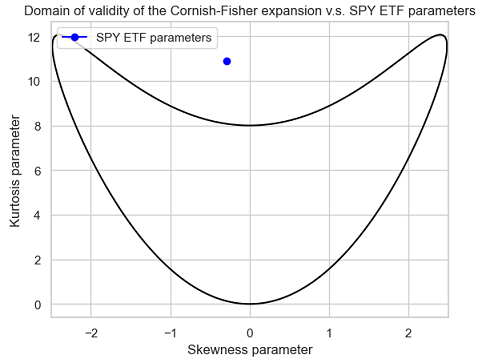Figure 4. Domain of validity of the Cornish-Fisher expansion v.s. SPY ETF parameters $\left( \kappa, \gamma \right)$.

Hopefully, there is a way to circumvent the relative narrowness of the domain of validity of the Cornish-Fisher expansion thanks to a regularization procedure called increasing rearrangement17 and described in details in Chernozhukov et al.18

The impact of this procedure is illustrated in Figure 5, which compares the same two distributions as in Figure 1, except that the Cornish-Fisher distribution has been rearranged.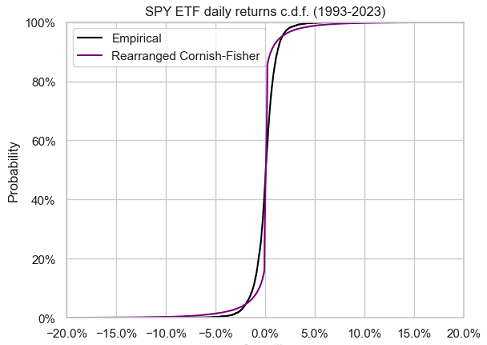Figure 5. SPY ETF daily returns, empirical c.d.f. v.s. rearranged Cornish-Fisher c.d.f., 1993-2023.

The rearranged Cornish-Fisher distribution is now monotonous, as it should be, but unfortunately, it only marginally better approximates the empirical distribution of the SPY ETF returns.

So, either all hope is lost w.r.t. using mVaR with moderately non-normal return distributions or there is another problem hidden somewhere waiting to be found…

Let’s dig a little bit further in Maillard6!

### Cornish-Fisher parameters v.s. actual moments

Maillard6 also notes that the scale, skewness and excess kurtosis parameters $\sigma$, $\kappa$ and $\gamma$ do not match the actual standard deviation $\sigma_{CF}$, skewness $\kappa_{CF}$ and excess kurtosis $\gamma_{CF}$ of the Cornish-Fisher distribution $\mathcal{CF}_{\mu, \sigma, \kappa, \gamma}$.

More precisely, he establishes the following relationships

\begin{align} \mu_{CF} &= \mu \\ \sigma_{CF} &= \sigma \sqrt{ 1 + \frac{1}{96} \gamma^2 + \frac{25}{1296} \kappa^4 - \frac{1}{36} \gamma \kappa^2 } \\ \kappa_{CF} &= f_1(\kappa, \gamma) \\ \gamma_{CF} &= f_2(\kappa, \gamma) \\ \end{align}

, where:

• $\mu_{CF}$, $\sigma_{CF}$, $\kappa_{CF}$ and $\gamma_{CF}$ are the actual mean, standard deviation, skewness and excess kurtosis of the Cornish-Fisher distribution $\mathcal{CF}_{\mu, \sigma, \kappa, \gamma}$
• $\mu$, $\sigma$, $\kappa$ and $\gamma$ are the location, scale, skewness and excess kurtosis parameters of the Cornish-Fisher distribution $\mathcal{CF}_{\mu, \sigma, \kappa, \gamma}$
• $f_1$ and $f_2$ are non linear functions, whose explicit formulas are provided in Maillard6

As a consequence, when the sample moments of a return distribution are used as plug-in estimators for the Cornish-Fisher parameters, the actual moments of the resulting Cornish-Fisher distribution differ from these sample moments!

Do they differ enough to create a real problem, though?

Re-using the SPY ETF example:

• The sample moments of the SPY ETF empirical return distribution are:
• $\mu_s \approx 0.000367$
• $\sigma_s \approx 0.011921$
• $\kappa_s \approx -0.287409$
• $\gamma_s \approx 10.898897$
• The actual moments of the Cornish-Fisher distribution $\mathcal{CF}_{\mu_s, \sigma_s, \kappa_s, \gamma_s}$, computed with Maillard’s relationships (1)-(4), are:
• $\mu_{CF} = \mu_s \approx 0.000367$
• $\sigma_{CF} \approx 0.017732$
• $\kappa_{CF} \approx −0.639885$
• $\gamma_{CF} \approx 62.437532$

So, yes, they do differ a lot, especially the excess kurtosis!

This subtlety is the hidden problem explaining19 the observed lack of accuracy of modified Value-at-Risk when return distributions are not close to normal5. Indeed, it cannot be expected from a “wrong” Cornish-Fisher distribution to accurately approximate anything useful.

The solution to this problem consists in inverting the relationships (1)-(4) between the actual moments and the parameters of the Cornish-Fisher distribution $\mathcal{CF}_{\mu, \sigma, \kappa, \gamma}$.

In other words, we need to determine the value of the parameters $\mu$, $\sigma$, $\kappa$ and $\gamma$ of the Cornish-Fisher distribution $\mathcal{CF}_{\mu, \sigma, \kappa, \gamma}$ so that its actual moments $\mu_{CF}$, $\sigma_{CF}$, $\kappa_{CF}$ and $\gamma_{CF}$ are equal to the sample moments $\mu_{s}$, $\sigma_{s}$, $\kappa_{s}$ and $\gamma_{s}$ of the empirical return distribution, c.f. Lamb et al.7.

More on how to do this numerically later.

The resulting Cornish-Fisher distribution is called the corrected Cornish-Fisher distribution $\mathcal{cCF}_{\mu_s, \sigma_s, \kappa_s, \gamma_s}$ and the underlying Cornish-Fisher expansion the corrected Cornish-Fisher expansion4.

Re-using one last time the SPY ETF example, we have:

• $\mu \approx 0.000367$
• $\sigma \approx 0.011217$
• $\kappa \approx -0.152059$
• $\gamma \approx 3.556476$

, and Figure 6 compares the resulting corrected Cornish-Fisher distribution to the two distributions of Figure 5.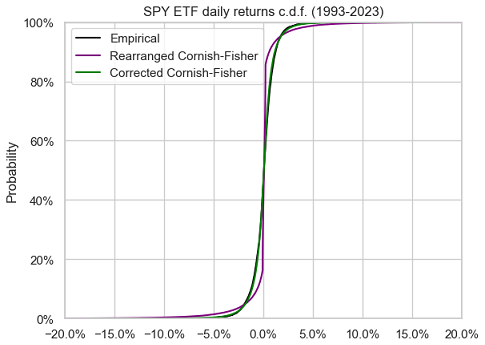Figure 6. SPY ETF daily returns, empirical c.d.f. v.s. rearranged Cornish-Fisher c.d.f. and corrected Cornish-Fisher c.d.f., 1993-2023.

The approximation of the empirical return distribution by the corrected Cornish-Fisher distribution is so accurate that these two distributions are nearly indistinguishable in this figure.

Figure 7, Figure 8 and Figure 9 compare the left tail of the three distributions from Figure 6.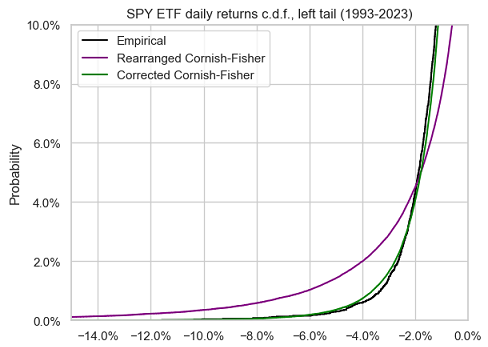Figure 7. SPY ETF daily returns, empirical c.d.f. v.s. rearranged Cornish-Fisher c.d.f. and corrected Cornish-Fisher c.d.f., left tail, 1993-2023.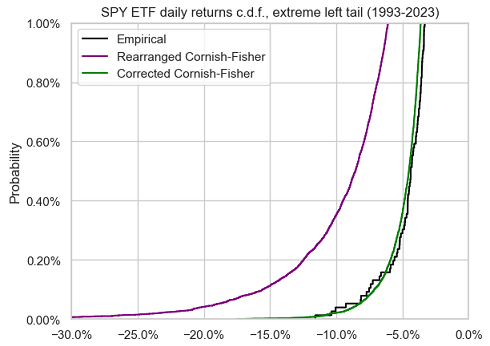Figure 8. SPY ETF daily returns, empirical c.d.f. v.s. rearranged Cornish-Fisher c.d.f. and corrected Cornish-Fisher c.d.f., extreme left tail, 1993-2023.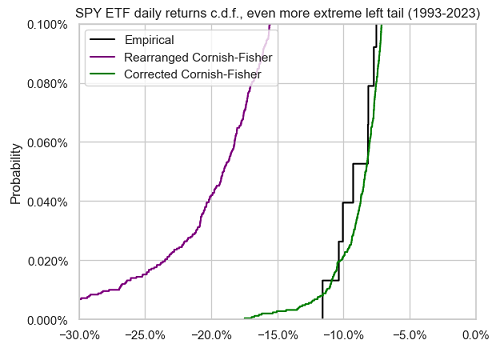Figure 9. SPY ETF daily returns, empirical c.d.f. v.s. rearranged Cornish-Fisher c.d.f. and corrected Cornish-Fisher c.d.f., even more extreme left tail, 1993-2023.

A nearly perfect fit again between the empirical return distribution and the corrected Cornish-Fisher distribution.

This example empirically demonstrates that modified Value-at-Risk, when corrected using Maillard6 results, works well for moderately non-normal distributions and for very small tail probabilities.

## Computing the corrected Cornish-Fisher distribution

As mentioned in the previous section, computing the corrected Cornish-Fisher distribution requires to invert the relationships (1)-(4) between the actual moments and the parameters of the Cornish-Fisher distribution $\mathcal{CF}_{\mu, \sigma, \kappa, \gamma}$.

Because the location parameter $\mu$ is invariant by (1), and because the scale parameter $\sigma$ is easily computed thanks to (2) once the skewness parameter $\kappa$ and the excess kurtosis parameter $\gamma$ have been computed, the main mathematical challenge is to invert the system of non-linear equations (3)-(4).

### The domain of validity of the corrected Cornish-Fisher expansion

Before thinking about how to invert these equations numerically, we first need to make sure that they are invertible theoretically.

Lamb et al.7 prove that this is the case when the actual skewness $\kappa_{CF}$ and the actual excess kurtosis $\gamma_{CF}$ belong20 to what could be called the domain of validity of the corrected Cornish-Fisher expansion21, represented in Figure 10.

Lamb et al.7 also establish that the resulting skewness parameter $\kappa$ and excess kurtosis parameter $\gamma$ belong to the domain of validity of the Cornish-Fisher expansion, which ensures that the resulting corrected Cornish-Fisher distribution is a proper distribution.

To be noted that the domain of validity of the corrected Cornish-Fisher expansion (Figure 10) is much wider than the domain of validity of the Cornish-Fisher expansion (Figure 3).

This is extremely important in applications, because the actual skewness $\kappa_{CF}$ and the actual excess kurtosis $\gamma_{CF}$ of the corrected Cornish-Fisher distribution typically correspond to the sample skewness $\kappa_s$ and to the sample excess kurtosis $\gamma_s$ of a given distribution22, so that the corrected Cornish-Fisher distribution is valid in practice for a much wider range of skewness and excess kurtosis than the non-corrected Cornish-Fisher distribution.

### The inversion procedure

At least two algorithms have been analyzed in the literature to compute the corrected Cornish-Fisher parameters from the actual moments:

### Implementation in Portfolio Optimizer

Portfolio Optimizer implements a proprietary algorithm to compute the parameters of the corrected Cornish-Fisher distribution, whose general description is:

• Determine the actual mean $\mu_s$, standard deviation $\sigma_s$, skewness $\kappa_s$ and excess kurtosis $\gamma_s$ of the corrected Cornish-Fisher distribution

These are either directly provided in input of the endpoint (e.g. /assets/returns/simulation/monte-carlo/cornish-fisher/corrected) or computed from an empirical distribution of returns (e.g. /portfolio/analysis/value-at-risk/cornish-fisher/corrected).

• If the skewness $\kappa_s$ and the excess kurtosis $\gamma_s$ belong to the domain of validity of the corrected Cornish-Fisher expansion, a robust iterative numerical method is then used to compute the skewness and excess kurtosis parameters $\kappa$ and $\gamma$.

Once these parameters are known, the relationships (1)-(4) allow to determine the resulting corrected Cornish-Fisher distribution $\mathcal{cCF}_{\mu_s, \sigma_s, \kappa_s, \gamma_s}$.

• Otherwise, a robust iterative numerical method is used to tentatively23 compute the skewness and excess kurtosis parameters $\kappa$ and $\gamma$
• If this computation is successful, the increasing rearrangement procedure of Chernozhukov et al.18 is applied to the resulting corrected Cornish-Fisher distribution $\mathcal{cCF}_{\mu_s, \sigma_s, \kappa_s, \gamma_s}$ in order to transform it into a valid distribution
• Otherwise, an error is raised

## Example of usage - Computing the modified Value-at-Risk of Bitcoin

Bitcoin is an example of asset exhibiting strong non-normal characteristics24, for which the standard measures of Value-at-Risk like Gaussian Value-at-Risk or modified Value-at-Risk would be inaccurate.

But what about modified Value-at-Risk based on the corrected Cornish-Fisher expansion?

In order to investigate the accuracy of this measure, that I will call corrected Cornish-Fisher Value-at-Risk (cCFVaR), Figure 11 compares, over the period 20 August 2011 - 06 April 2023, the empirical distribution of Bitcoin daily returns14 to the corrected Cornish-Fisher distribution $\mathcal{cCF}_{\mu_s, \sigma_s, \kappa_s, \gamma_s}$ with actual moments:

• $\mu_s \approx 0.001863$, the sample mean of Bitcoin returns over the considered period
• $\sigma_s \approx 0.047369$, the sample standard deviation of Bitcoin returns over the considered period
• $\kappa_s \approx -1.368879$, the sample skewness of Bitcoin returns over the considered period
• $\gamma_s \approx 24.594523$, the sample excess kurtosis of Bitcoin returns over the considered period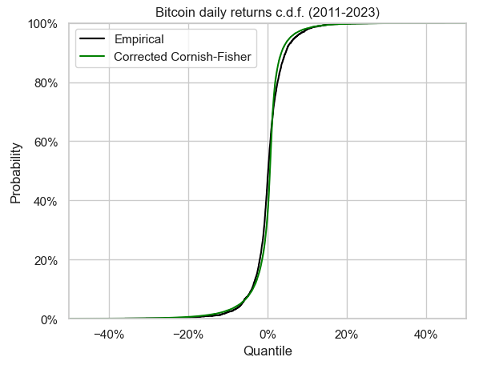Figure 11. Bitcoin daily returns, empirical c.d.f. v.s. corrected Cornish-Fisher c.d.f., 2011-2023.

It seems that the corrected Cornish-Fisher distribution does a pretty good job in approximating the empirical return distribution of Bitcoin, except in the right tail though.

Figure 12 and Figure 13 compare the left tail of these two distributions.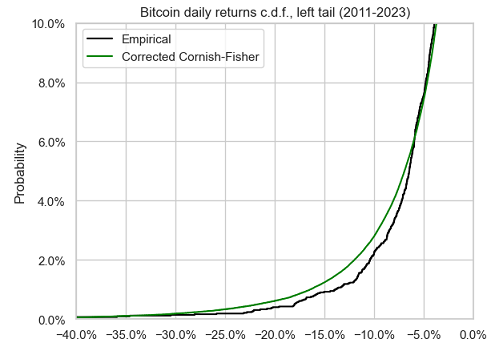Figure 12. Bitcoin daily returns, empirical c.d.f. v.s. corrected Cornish-Fisher c.d.f., left tail, 2011-2023.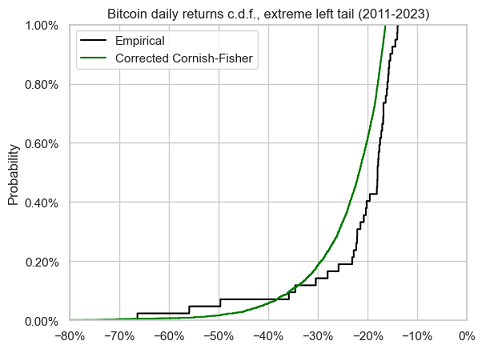Figure 13. Bitcoin daily returns, empirical c.d.f. v.s. corrected Cornish-Fisher c.d.f., extreme left tail, 2011-2023.

There figures confirm that the corrected Cornish-Fisher distribution accurately approximates the empirical return distribution of Bitcoin down to a confidence level of $\approx 95\%$, but no lower.

This can also be confirmed numerically, with a comparison between historical Value-at-Risk and corrected Cornish-Fisher Value-at-Risk at different confidence levels:

Confidence level $\alpha$ $\text{HVaR}_{\alpha}$ $\text{cCFVaR}_{\alpha}$
95% 6.90% 6.86%
97.5% 9.53% 10.63%
99% 13.36% 16.51%
99.5% 15.92% 21.56%
99.9% 27.04% 35.08%

All in all, this example empirically demonstrates that modified Value-at-Risk, when corrected following Maillard6 results, works well for highly non-normal distributions with not too small tail probabilities.

## Conclusion

The goal of this post was to highlight that accuracy issues reported by practitioneers with modified Value-at-Risk have been understood since more than ten years, but that, as Amedee-Manesme et al.4 put it:

this point […] does not seem to have received sufficient attention

If you are such a practitioneer, I hope that this post will encourage you to double check how modified Value-at-Risk is computed by your internal risk management software.

1. For example, European financial regulators require to use mVaR in order to compute the Summary Risk Indicator (SRI), i.e. the risk score, of Packaged Retail Investment and Insurance Products (PRIIPs) starting 1st January 2023, c.f. regulatory Technical Standards on the content and presentation of the KIDs for PRIIPs

2. Like 1% quantile or even less.

3. In this post, returns are assumed to be logarithmic returns.

4. This is the case when the portfolio return cumulative distribution function is strictly increasing and continuous; otherwise, a similar formula is still valid, with $F_X^{-1}$ the generalized inverse distribution function of $X$, but these subtleties - important in mathematical proofs and in numerical implementations - are out of scope of this post.

5. Asset returns have a tendency to follow a distribution closer and closer to a Gaussian distribution the more the time period over which they are computed increases; this empirical property is called aggregational Gaussianity, c.f. Cont25

6. The associated adjusted prices have been retrieved using Tiingo 2

7. This also means that it is possible to have $\text{mVaR}_{95\%} > \text{mVaR}_{99\%}$, which requires some funny arguments to be explained…

8. I will not enter into the mathematical details in this post, but it suffices to say that this procedure allows to correct the behavior of the Cornish-Fisher expansion when used outside of its domain of validity thanks to a sorting operator.

9. In addition, Maillard6 mentions that when the skewness and excess kurtosis parameters are small enough, in a loose sense, they coincide with the actual skewness and excess kurtosis of the Cornish-Fisher distribution, which perfectly explains the behavior of the modified Value-at-Risk observed in practice with return distributions close to normal5

10. Actually, the result of Lamb et al.7 is a little bit more generic: they establish that the system of non-linear equations is invertible on a region which includes the domain of validity of the Cornish-Fisher expansion.

11. The domain of validity of the corrected Cornish-Fisher expansion is the mathematical image, by the functions $f_1$ and $f_2$, of the domain of validity of the Cornish-Fisher expansion.

12. In the context of this blog post, the given distribution is a return distribution (asset, portfolio, strategy…).

13. This tentative computation is theoretically justified by the results from Lamb et al.7

]]>
Roman R.
The Mathematics of Bonds: Simulating the Returns of Constant Maturity Government Bond ETFs2023-03-19T00:00:00+00:002023-03-19T00:00:00+00:00https://portfoliooptimizer.io/blog/the-mathematics-of-bonds-simulating-the-returns-of-constant-maturity-government-bond-etfsWith more than $1.2 trillion under management in the U.S. as of mid-July 20221, investors are more and more using bond ETFs as building blocks in their asset allocation. One issue with such instruments, though, is that their price history dates back to at best 20021, which is problematic in some applications like trading strategy backtesting or portfolio historical stress-testing. In this post, which builds on the paper Treasury Bond Return Data Starting in 1962 from Laurens Swinkels2, I will show that the returns of specific bond ETFs - those seeking a constant maturity exposure to government-issued bonds - can be simulated using standard textbook formulas2 together with appropriate yields to maturity. This allows in particular to extend the price history of these ETFs by several tens of years thanks to publicly available yield to maturity series published by governments, government-affiliated agencies, researchers… Notes: • A Google sheet corresponding to this post is available here ## Mathematical preliminaries ### Bond yield formula In what comes next, I will make heavy use of the formula expressing the price of a bond as a function of its yield to maturity. This formula can be found in the appendix A3.1 Yield to maturity for settlement dates other than coupon payment dates of Tuckman and Serrat3, and is reproduced below for convenience. Le be a bond4 at a date$t$, with a remaining maturity equal to$T$, a yield to maturity equal to$y_t$and a coupon rate equal to$c_t$. Then, its price$P_t(c_t,y_t,T)$per 100 face amount is equal to $\left( 1 + \frac{y_t}{2} \right)^{1 - \tau_{t}} \left[ \frac{100 c_{t}}{y_t} \left( 1 - \frac{1}{\left( 1 + \frac{y_t}{2} \right)^{2T}} \right) + \frac{100}{\left( 1 + \frac{y_t}{2} \right)^{2T}} \right] - 1$ , where$\tau_{t}$is the fraction of a semiannual period until the next coupon payment. ## Par bond total return formula Using the bond yield formula, it is possible to approximate the total return$TR$of a par bond over a specific period using only its remaining maturity at the beginning of the period, its yield to maturity at the beginning of the period and its yield to maturity at the end of the period. ### Par bond total return formula for a monthly period In the case of a monthly period, let be a bond such that: • Its remaining maturity at the end of the month$t-1$is equal to$T$• Its yield to maturity at the end of the month$t-1$, for a remaining maturity equal to$T$, is$y_{t-1}$• Its yield to maturity at the end of the month$t$, for a remaining maturity equal to$T$, is$y_{t}$Then, assuming that • The bond trades at par at the end of the month$t-1$• The bond yield curve at the end of the month$t$is flat for remaining maturities between$T - \frac{1}{12}$and$T$, the total return$TR_t$of this bond from the end of the month$t-1$to the end of the month$t$can be approximated by $\frac{y_{t-1}}{12} + \frac{y_{t-1}}{y_t} \left( 1 - \frac{1}{\left( 1 + \frac{y_t}{2} \right)^{2(T-\frac{1}{12})}} \right) + \frac{1}{\left( 1 + \frac{y_t}{2} \right)^{2(T-\frac{1}{12})}} - 1$ ### Demonstration of the par bond total return formula for a monthly period A possible demonstration for the previous formula goes as follows. At the end of the month$t-1$, the bond has the following characteristics: • Its remaining maturity is equal to$T$• Its coupon rate$c_{t-1}$is equal to its yield to maturity$y_{t-1}$, because of the assumption that the bond trades at par at the end of the month$t-1$Its price$P_{t-1}(c_{t-1},y_{t-1},T)$is then equal, through the bond yield formula, to $100 \left( 1 + \frac{y_{t-1}}{2} \right)^{1 - \tau_{t-1}}$ , with$\tau_{t-1}$the fraction of a semiannual period until the next coupon payment at the end of month$t-1$. At the end of the month$t$, the bond has the following characteristics: • Its remaining maturity is equal to$T - \frac{1}{12}$, one month short of its initial remaining maturity$T$• Its coupon rate$c_{t}$is equal to$c_{t-1}$, that is, its initial yield to maturity$y_{t-1}$• Its yield to maturity is equal to$y_{t}$, because of the assumption on the bond yield curve at the end of the month$t$Its price$P_t(c_{t},y_{t},T - \frac{1}{12})$is then equal, through the bond yield formula, to $\left( 1 + \frac{y_t}{2} \right)^{1 - \tau_{t}} \left[ \frac{100 y_{t-1}}{y_t} \left( 1 - \frac{1}{\left( 1 + \frac{y_t}{2} \right)^{2(T-\frac{1}{12})}} \right) + \frac{100}{\left( 1 + \frac{y_t}{2} \right)^{2(T-\frac{1}{12})}} \right]$ , with$\tau_{t}$the fraction of a semiannual period until the next coupon payment at the end of month$t$. The total return$TR_t$of this bond from the end of the month$t-1$to the end of the month$t$is then by definition equal to $\frac{P_t(c_{t},y_{t},T - \frac{1}{12})}{P_{t-1}(c_{t-1},y_{t-1},T)} - 1$ , that is $\frac{\left( 1 + \frac{y_t}{2} \right)^{1 - \tau_{t}}}{\left( 1 + \frac{y_{t-1}}{2} \right)^{1 - \tau_{t-1}}} \left[ \frac{y_{t-1}}{y_t} \left( 1 - \frac{1}{\left( 1 + \frac{y_t}{2} \right)^{2(T-\frac{1}{12})}} \right) + \frac{1}{\left( 1 + \frac{y_t}{2} \right)^{2(T-\frac{1}{12})}} \right] - 1$ The first term of this expression corresponds to the re-investment of the accrued interest. Under the practical assumptions that • There is only a single rate for accrued interest, chosen equal to$y_{t-1}$5 • The accrued interest is not re-invested6 and noticing that$ \tau_{t} = \tau_{t-1} - \frac{1}{6}$7, this expression becomes $TR_t \approx \left[ \left( 1 + \frac{y_{t-1}}{2} \right)^{\frac{1}{6}} - 1 \right] + \left[ \frac{y_{t-1}}{y_t} \left( 1 - \frac{1}{\left( 1 + \frac{y_t}{2} \right)^{2(T-\frac{1}{12})}} \right) + \frac{1}{\left( 1 + \frac{y_t}{2} \right)^{2(T-\frac{1}{12})}} \right] - 1$ Finally, by linearizing the accrued interest through the first-order Taylor approximation$ \left( 1 + \frac{y_{t-1}}{2} \right)^{\frac{1}{6}} \approx \frac{y_{t-1}}{12} $, this expression becomes $TR_t \approx \frac{y_{t-1}}{12} + \left[ \frac{y_{t-1}}{y_t} \left( 1 - \frac{1}{\left( 1 + \frac{y_t}{2} \right)^{2(T-\frac{1}{12})}} \right) + \frac{1}{\left( 1 + \frac{y_t}{2} \right)^{2(T-\frac{1}{12})}} \right] - 1$ Remark: • The formula above is based on a suggestion by Dr Winfried Hallerbach to improve the accuracy of the initial formula used in Swinkels2 which is based on a second-order Taylor approximation of the bond yield formula, c.f. Swinkels8. ## Constructing return series for constant maturity government bonds ### Methodology Thanks to a variation9 of the par bond total return formula established in the previous section, Swinkels2 describes how to construct long (total) return series for government bonds using publicly available constant maturity government rates10. These rates correspond to the yields to maturity of (fictitious) government bonds whose maturity is kept constant and are typically estimated by governments or government-affiliated agencies, which explains why they are publicly available. For example: • In the U.S., they are called Constant Maturity Treasury Rates (CMTs), or Treasury Par Yield Curve Rates, and are published on: • In France, they are called CNO-TEC and are published on the Banque de France website. As a side note, long return series for government bonds are usually commercially licensed (Global Financial Data, Bloomberg…), so that the methodology of Swinkels2 participates to have a high-quality public alternative to commercially available data2 for research purposes. ### Illustration As an illustration of the methodology of Swinkels2, below are yields to maturity for 3 consecutive months taken from the FRED 10-Year Treasury Constant Maturity Rates series: Date Yield to maturity 31 Dec 2022 3.880% 31 Jan 2023 3.520% 28 Feb 2023 3.920% The total return series$ \left( TR_1, TR_2 \right) $of the fictitious 10-year constant maturity government bond associated to these yields to maturity is then constructed by: • Computing the total return$TR_1$from 31 Dec 2022 to 31 Jan 2023 thanks to the par bond total return formula, with$T = 10$,$y_{t-1} = 3.880\%$and$y_t=3.520\%$. This gives $TR_1 \approx \frac{0.0388}{12} + \frac{0.0388}{0.0352} \left( 1 - \frac{1}{\left( 1 + \frac{0.0352}{2} \right)^{2(10-\frac{1}{12})}} \right) + \frac{1}{\left( 1 + \frac{0.0352}{2} \right)^{2(10-\frac{1}{12})}} - 1$ That is $TR_1 \approx 3.31\%$ • Computing the total return$TR_2$from 31 Jan 2023 to 28 Feb 2023 thanks again to the par bond total return formula, but with this time$T = 10$11,$y_{t-1} = 3.520\%$and$y_t=3.920\%$. This gives $TR_2 \approx \frac{0.0352}{12} + \frac{0.0352}{0.0392} \left( 1 - \frac{1}{\left( 1 + \frac{0.0392}{2} \right)^{2(10-\frac{1}{12})}} \right) + \frac{1}{\left( 1 + \frac{0.0392}{2} \right)^{2(10-\frac{1}{12})}} - 1$ That is $TR_2 \approx -2.97\%$ ### Implementation in Portfolio Optimizer The Portfolio Optimizer endpoint /bonds/returns/par/constant-maturity implements the methodology of Swinkels2 using the par bond total return formula established in the previous section. ## Simulating the returns of constant maturity government bond ETFs ### Rationale Many government bond ETFs target a specific maturity, a specific average maturity or a specific maturity range for their underlying portfolio of government bonds. For example, the iShares 7-10 Year Treasury Bond ETF seeks to track the investment results of an index composed of U.S. Treasury bonds with remaining maturities between seven and ten years12. Intuitively, such ETFs should more or less behave like a constant maturity government bond, so that it should be possible to simulate their (total) returns using the methodology of Swinkels2 detailed in the previous section. Nevertheless, and especially because these ETFs need to frequently rebalance their holdings13, such simulated returns might not be accurate enough to be of any practical use… Let’s dig in. ### Theory v.s. reality In order to illustrate the quality of the simulated returns discussed above, Figure 1 through Figure 5 compare the actual returns of the members of the iShares family of U.S. Treasury bond ETFs to the theoretical returns simulated using the methodology of Swinkels2. The theoretical returns of this ETF are simulated with the FRED 3-Year Treasury Constant Maturity Rates.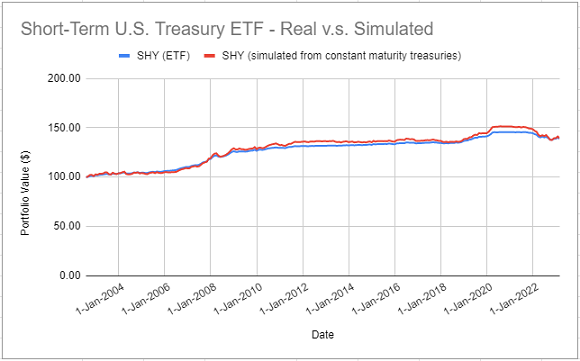Figure 1. Actual SHY ETF returns v.s. simulated returns with 3-year Treasury constant maturity rates, August 2002 - February 2023. The theoretical returns of this ETF are simulated with the FRED 7-Year Treasury Constant Maturity Rates.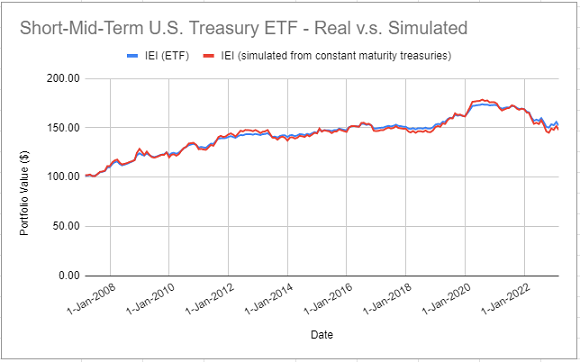Figure 2. Actual IEI ETF returns v.s. simulated returns with 7-year Treasury constant maturity rates, February 2007 - February 2023. The theoretical returns of this ETF are simulated with the FRED 10-Year Treasury Constant Maturity Rates.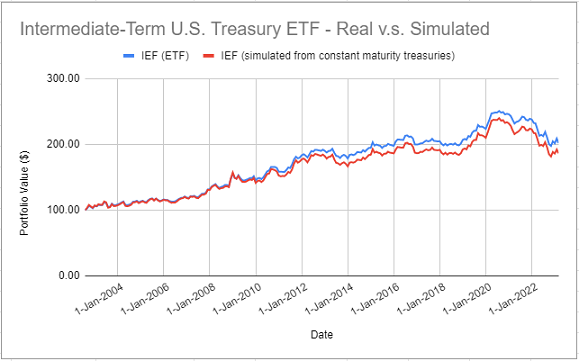Figure 3. Actual IEF ETF returns v.s. simulated returns with 10-year Treasury constant maturity rates, August 2002 - February 2023. The theoretical returns of this ETF are simulated with the FRED 20-Year Treasury Constant Maturity Rates.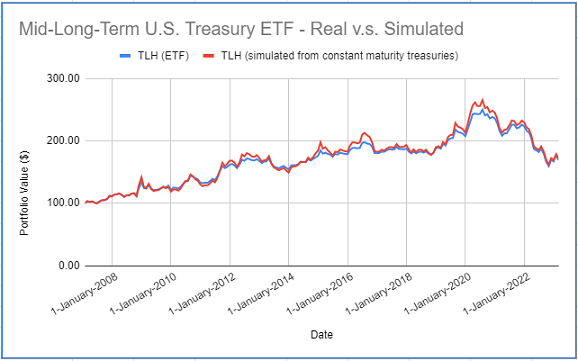Figure 4. Actual TLH ETF returns v.s. simulated returns with 20-year Treasury constant maturity rates, February 2007 - February 2023. The theoretical returns of this ETF are simulated with the FRED 30-Year Treasury Constant Maturity Rates.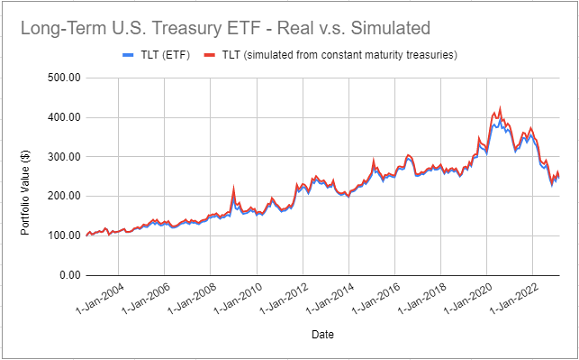Figure 5. Actual TLT ETF returns v.s. simulated returns with 30-year Treasury constant maturity rates, August 2002 - February 2023. On all these figures, it is clear that simulated returns are closely matching actual returns. The IEF ETF is an exception, though, because several simulated returns were significantly different from their actual counterparts over the period 2012 - 2014. Nevertheless, for all the five ETFs, correlations between actual and simulated returns are greater than ~97%, which confirms that it is possible to accurately simulate the returns of constant maturity government bond ETFs14 using the methodology of Swinkels2. ## Simulating the returns of non-constant maturity government bond ETFs Each of the five ETFs analyzed in the previous section invests over a given segment of the U.S. Treasury yield curve (1-3 years, 3-7 years, 10-20 years…). This segment is sometimes wide, like in case of the TLH ETF, but this characteristic allows these ETFs to be considered as constant maturity government bonds. Now, what about non-constant maturity government bond ETFs? To answer this question empirically, Figure 6 compares the actual returns of the iShares U.S. Treasury Bond ETF (GOVT ETF) to the theoretical returns simulated using the methodology of Swinkels2 with a weighted average of 3-year, 7-year, 10-year, 20-year and 30-year Treasury constant maturity rates15.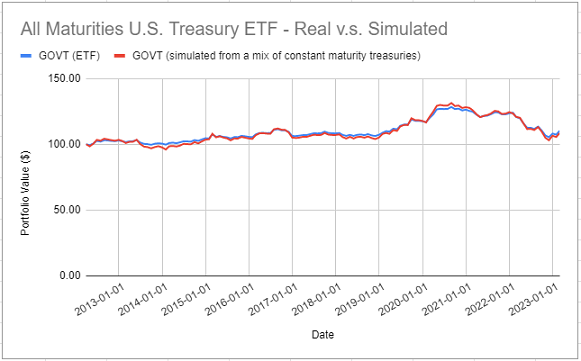Figure 6. Actual GOVT ETF returns v.s. simulated returns with a mix of Treasury constant maturity rates, March 2012 - February 2023. Once again, it appears that simulated returns are closely matching actual returns16. This example shows that, at least in some cases, it should be possible to accurately simulate the (total) returns of non-constant maturity government bond ETFs using the methodology of Swinkels2, provided that these ETFs are considered as a weighted average of constant maturity government bonds instead of a single constant maturity government bond. ## Extending the price history of constant maturity government bond ETFs The previous sections demonstrated that it is possible to simulate quite accurately the returns of constant maturity government bond ETFs. This opens the door to extending their price history. I will use the TLT ETF as an example. Figure 5 showed that the actual returns of the TLT ETF are devilishly close to the theoretical returns simulated using the methodology of Swinkels2 with the FRED 30-Year Treasury Constant Maturity Rates series. As a consequence, because the FRED provides the historical values of these rates back to February 1977, the price history of the TLT ETF can be extended by ~25 years. This extended history is depicted in Figure 7.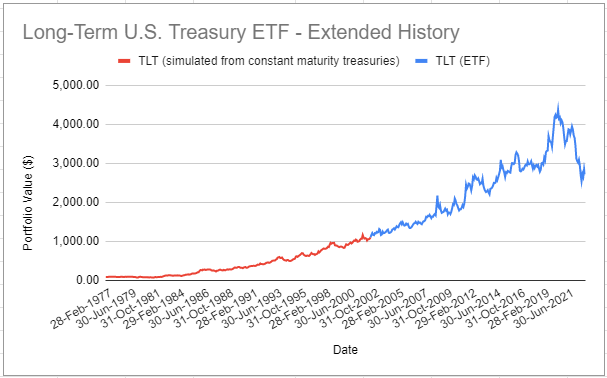Figure 7. Actual TLT ETF returns and extended TLT returns simulated with 30-year Treasury constant maturity rates, March 1977 - February 2023. ## Conclusion This blog post described how to use the methodology of Swinkels2 to simulate present and past returns of constant maturity government bond ETFs. One possible next step is to also use this methodology to simulate future returns of such ETFs, from views on future yields to maturity. Maybe the subject of another post. Meanwhile, feel free to connect with me on LinkedIn or follow me on Twitter to discuss about Portfolio Optimizer or about how to best approximate bond ETFs returns :-) ! 1. In this post, I use the same conventions as in Tuckman and Serrat3: bonds are assumed to be paying semiannual coupons, their coupon rate is assumed to be annual, their yield to maturity is assumed to be provided as semiannually compounded and their maturity is assumed to be expressed in years. 2. Another sensible choice would be to use a rate equal to$\frac{y_{t-1} + y_{t}}{2}$. 3. Since bonds with semi-annual coupons are paying coupons every six months, these coupons are anyway hardly collected and re-invested every month in practice, so that this is a sensible simplifying assumption. 4. C.f. Tuckman and Serrat3 for explanations about the term$\frac{1}{6}$. 5. C.f. the remark at the end of the previous section. 6. Constant yield to maturity rates are frequently estimated from government bonds that trade close to par, even though interest rates have changed since their original issuance, which justifies the usage of this formula, c.f. Swinkels2 7. The maturity$T$did not change because the bond is supposed to have a constant maturity. 8. For example, in order to target a specific maturity range, a government bond ETF must replace its holdings whose remaining maturity has become too short. As a side note, this behaviour explains the crazy annual portfolio turnover rate of these ETFs, with for example a turnover rate of 114% for the iShares 7-10 Year Treasury Bond ETF in 202217 9. Or at the very least, to accurately simulate the (total) returns of some constant maturity government bond ETFs. 10. The weights correspond to the percentage breakdown of the GOVT ETF portfolio per maturity, retrieved from the iShares U.S. Treasury Bond ETF website on 19 March 2023. 11. Numerically, correlation between actual and simulated returns is ~98%. ]]> Roman R. The Turbulence Index: Regime-based Partitioning of Asset Returns2023-03-07T00:00:00+00:002023-03-07T00:00:00+00:00https://portfoliooptimizer.io/blog/the-turbulence-index-regime-based-partitioning-of-asset-returnsThe turbulence index, introduced in the previous blog post, is a measure of statistical unusualness of asset returns popularized by Kritzman and Li1. It provides a way to measure how much the behavior of a group of assets differs from its historical pattern. In this post, based on the paper Optimal Portfolios in Good Times and Bad by Chow et al.2, I will describe how the turbulence index can be used to partition a set of asset returns into different subsets, each of them corresponding to a specific market risk regime. I will also provide two examples of usage, one in portfolio optimization and one in the modeling of asset returns. ## Mathematical preliminaries ### Definitions Let be: •$n$, the number of assets in a given universe of assets •$T$, the number of time periods •$y_t \in \mathbb{R}^{n}$, the asset returns observed over the time period$t$,$t=1..T$•$\mu \in \mathbb{R}^{n}$, the mean vector of the asset returns over the$T$periods •$\Sigma \in \mathcal{M}(\mathbb{R}^{n \times n})$, the covariance matrix of the asset returns over the$T$periods The (raw) turbulence index$d(y_t)$for the universe of assets and for a given period$t=1..T$is defined as the squared Mahalanobis distance2: $d(y_t) = (y_t - \mu) {}^t \Sigma^{-1} (y_t - \mu)$ ### Mathematical properties The main mathematical property of the turbulence index relevant for this post3 is the following2: Property 1: If the asset returns$y_t$follow a multivariate Gaussian distribution, that is,$y_t \sim \mathcal{N} \left( \mu, \Sigma \right)$, the turbulence index$d(y_t)$follows a chi-square distribution with$n$degrees of freedom, that is,$d(y_t) \sim \mathcal{X}^2(n)$. ## A method to partition asset returns by regimes based on the turbulence index ### Description Chow et al.2 describe how to use the turbulence index to identify multivariate outliers from a series of asset returns. These outliers, that are characterized by the unusual performance of an individual asset or from the unusual interaction of a combination of assets, none of which are necessarily unusual in isolation2 are representative of a [turbulent4 market] risk regime while the inliers are representative of a quiet market risk regime2. In more details, the method of Chow et al.2 partitions a set of multivariate asset returns$y_t \in \mathbb{R}^{n}, t=1..T$into two subsets corresponding to these two regimes as follows: • Compute the mean vector$\mu$of the asset returns • Compute the covariance matrix$\Sigma$of the asset returns • Choose a turbulence threshold$tt$%, which represents the percentage of asset returns desired to be classified as quiet, with typical value 70%, 80%, 95%5 • Convert the turbulence threshold$tt$% into a turbulence score$ts$• For each asset return vector$y_t, t=1..T$: • Compute the turbulence index value$d(y_t)$• If$d(y_t) \leq ts$,$y_t$is classified as belonging to the quiet regime; otherwise, if$d(y_t) > ts$,$y_t$is classified as belonging to the turbulent regime ### How to convert the turbulence threshold into a turbulence score? The turbulence threshold$tt$% is not directly comparable to the turbulence index values$d(y_t), t=1..T$because they are not expressed in the same units6. As a consequence,$tt$% needs to be converted into a turbulence score$ts$. Under the assumption that the asset returns$y_t$follow a multivariate Gaussian distribution, and based on Property 1, this conversion can be done by thanks to the computation of the$tt$-th percentile of the chi-square distribution with$n$degrees of freedom, that is, $ts = \left( \mathcal{X}^2(n) \right)^{-1} (tt)$ Nevertheless, because asset returns do not follow a multivariate Gaussian distribution in practice7, this conversion will result in a proportion of asset returns classified as quiet that is different from the desired proportion. This problem is highlighted in Chow et al.2, in which a turbulence threshold of 75% is used to separate outliers from inliers while the actual proportion of asset returns classified as quiet v.s. turbulent is equal to 79.1%. A possible solution is to convert the turbulence threshold$tt$% into a turbulence score thanks to the computation of the$tt$-th empirical percentile of the turbulence index distribution89, with the caveat that this solution requires a long enough series of asset returns. ### Illustration I will illustrate the method of Chow et al.2 with a simple two-asset universe made of: • U.S. stocks (SPY ETF) • U.S. 20+ year Treasuries (TLT ETF) The turbulence index for this universe of assets, computed using monthly returns over the period August 2002 - January 202310, is represented in Figure 1.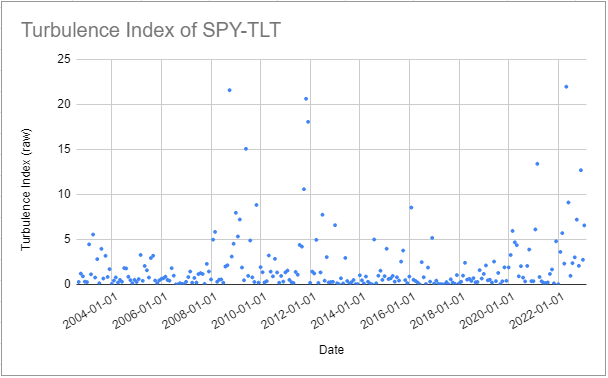Figure 1. SPY-TLT turbulence index, August 2002 - January 2023. Then, using for example a turbulence threshold$tt$% of 80%, converted into a turbulence score$ts$of ~3.2211: • All pairs of SPY-TLT returns whose turbulence index is below the red line depicted in Figure 2 are classified as belonging to the quiet regime • All pairs of SPY-TLT returns whose turbulence index is strictly above the red line depicted in Figure 2 are classified as belonging to the turbulent regime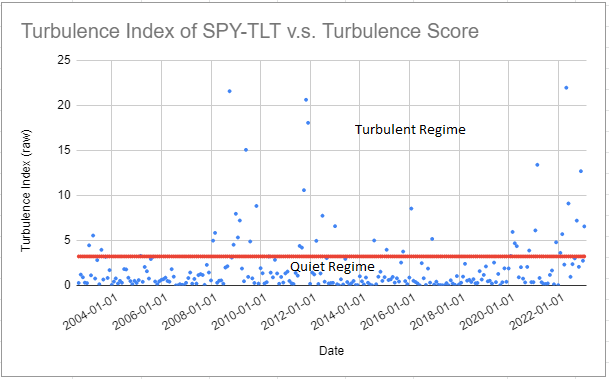Figure 2. SPY-TLT turbulence index v.s. turbulence score of 80%, August 2002 - January 2023. This partitioning of the SPY-TLT returns seems to make some sense, as several periods of market stress are identifiable within the turbulent regime: the Global Financial Crisis, the COVID-19 pandemic, the Russian invasion of Ukraine ### Implementation in Portfolio Optimizer Portfolio Optimizer implements the method from Chow et al.2 through the endpoint /assets/returns/turbulence-partitioned, with two extensions: • Several turbulence thresholds$tt_i$%,$i=1..m$,$m \geq 1$can be provided, in which case the initial set of asset returns is partitioned into (at most)$m+1$subsets12 • Turbulence threshold(s) can be converted into turbulence score(s) thanks to the computation of the empirical percentiles of the turbulence index distribution, as described in Kritzman et al.89 ## Examples of application Being able to partition asset returns into different market risk regimes has several applications. For example, it allows to analyze the potential behavior of a given portfolio during periods of market stress, which is of utmost importance for long-term investing. As Chow et al.2 puts it: [a] portfolio may not survive to generate long-term performance if [it] cannot withstand exceptional periods of market turbulence. I will not insist on this specific example, though, but I will provide one example related to portfolio optimization and one example related to the modeling of asset returns. ### Building mean-variance optimal and regime-sensitive portfolios Under the Markowitz’s mean-variance framework, building an optimal portfolio within a universe of assets requires an estimation of the asset covariance matrix. Because [the] typical risk-estimation procedure […] is to weight a sample [of asset returns]’ observations equally in order to estimate risk parameters2, the expected volatility of such a portfolio during periods of market stress, when asset returns typically become more volatile and more correlated, will be underestimated. This situation is illustrated in Figure 3, taken from Chow et al.2, in the case of a universe of assets made of eight distinct asset classes13.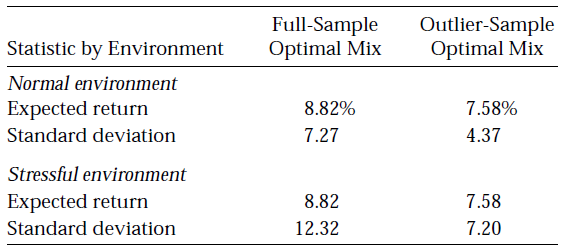Figure 3. Impact of full sample v.s. turbulent sample asset covariance matrix estimation on a mean-variance optimal portfolio. Source: Chow et al. In this figure, it can be seen that an optimal portfolio whose asset covariance matrix is estimated from a non-partitioned set of asset returns (Full-Sample Optimal Mix) sees its volatility skyrocket during periods of market stress (Stressful environment) when compared to periods of market stability (Normal environment). One solution to this issue is to estimate the asset covariance matrix from only the subset of asset returns corresponding to periods of market stress, but in this case, the expected portfolio return might be negatively impacted. Indeed, as can also be seen in Figure 3, an optimal portfolio whose asset covariance matrix is estimated from only the subset of asset returns corresponding to periods of market stress (Outlier-Sample Optimal Mix) sees its expected return diminished by$\approx 1.24$% compared to the previous optimal portfolio (Full-Sample Optimal Mix). Another solution, suggested by Chow et al2 and Kritzman et al.9, is to estimate a blended asset covariance matrix from both14: • The subset of asset returns corresponding to periods of market stress • The subset of asset returns corresponding to periods of market stability More specifically, these papers suggest to estimate an asset covariance matrix$\Sigma^*$equal to $\Sigma^* = \lambda_{i}^* p_{i} \Sigma_{i} + \lambda_{o}^* \left( 1 - p_{i} \right) \Sigma_{o}$ , where: •$\lambda_i^*$(resp.$\lambda_o^*$) is the relative15 risk aversion of investors to the quiet (resp. turbulent) regime •$p_{i}$(resp.$1 - p_{i}$16) is the probability that the next period’s regime is quiet (resp. turbulent) •$\Sigma_{i}$is the quiet regime asset covariance matrix, estimated from the subset of asset returns corresponding to the quiet regime •$\Sigma_{o}$is the turbulent regime asset covariance matrix, estimated from the subset of asset returns corresponding to the turbulent regime Such a blended covariance matrix enables investors to express their views about the likelihood of each risk regime and to differentiate their aversion to the regimes2. Now, in practice, the computation of$\Sigma^*$requires to estimate the probability$p_{i}$. This can be done in an ad-hoc fashion, or using one’s preferred forecast technique, like the hidden Markov model used in Kritzman et al.9. A couple of remarks to finish: • Under the Markowitz’s mean-variance framework, building an optimal portfolio within a universe of assets also requires an estimation of the expected asset returns. They are assumed to be regime-independent in Chow et al.2, but they could perfectly be made conditional on the regime like in Bruder et al.17. • In the specific case where investors are equally averse to both the quiet and the turbulent regime, the formula for$\Sigma^*$simplifies to $\Sigma^* = p_{i} \Sigma_{i} + \left( 1 - p_{i} \right) \Sigma_{o}$ ### Fitting the parameters of a multivariate Gaussian mixture distribution It has been known since the early 1960s that the (marginal) statistical distribution of asset returns is neither normal nor lognormal7, but more than sixty years later it is still an open question in financial mathematics to determine its exact nature. Empirically, though, it has been demonstrated that several distributions are able to capture most of the stylized facts18 of asset returns. One such distribution is the Gaussian mixture distribution, which is a convex combination of Gaussian distributions with different means and variances in the univariate case and a a convex combination of multivariate Gaussian distributions with different mean vectors and covariance matrices in the multivariate case. The main advantages of this distribution over other alternatives like the multivariate t distribution is that it is a non-elliptical distribution19 both numerically tractable20 and extremely flexible. For example, a univariate Gaussian mixture distribution can be unimodal, symmetric, skewed, multimodal, leptokurtic…21. As another example, a multivariate Gaussian mixture distribution with two components allows to approximate a multivariate jump-diffusion model driven by a standard Lévy process17. In this context, the method of Chow et al.2 can be applied to fit the parameters of a two-component22 multivariate Gaussian mixture distribution as detailed below23: • Set the weights of the two components respectively to the turbulence threshold$tt$%24 and its complement$1 - tt$% • Estimate the mean vector and the covariance matrix of the multivariate Gaussian distribution with component weight$tt$% by their sample counterparts computed on the quiet subset of asset returns • Estimate the mean vector and the covariance matrix of the multivariate Gaussian distribution with component weight$1 - tt$% by their sample counterparts computed on the turbulent subset of asset returns In order to illustrate the validity of this approach on the two-asset SPY-TLT universe introduced in the previous section, Figure 4 through Figure 6 compare the empirical distribution of the monthly SPY (log) returns with the first marginal of: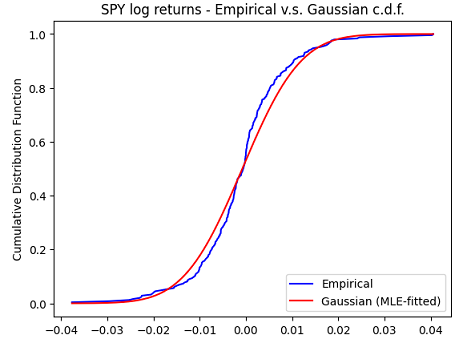Figure 4. Monthly SPY log returns, empirical c.d.f. v.s. Gaussian c.d.f. fitted with maximum likelihood estimation, August 2002 - January 2023. • A multivariate Gaussian mixture distribution fitted to the pairs of SPY-TLT returns with the turbulence index-based methodology above using a turbulence threshold of 80%25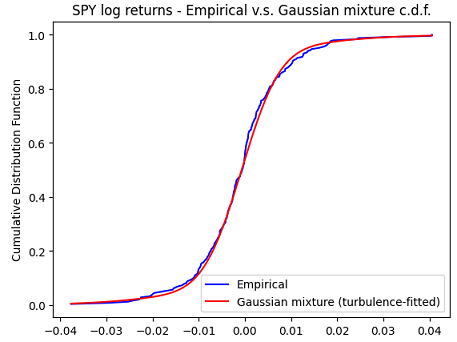Figure 5. Monthly SPY log returns, empirical c.d.f. v.s. Gaussian mixture c.d.f. fitted with the turbulence index-based methodology, August 2002 - January 2023.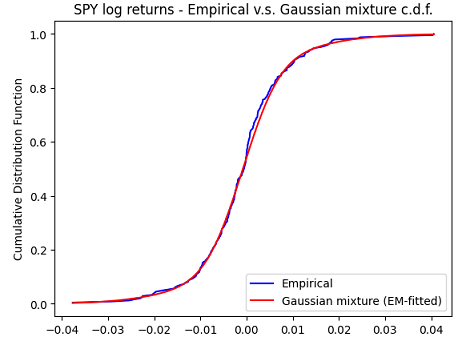Figure 6. Monthly SPY log returns, empirical c.d.f. v.s. Gaussian mixture c.d.f. fitted with the expectation–maximization algorithm, August 2002 - January 2023. It is clearly visible on these figures that both marginal Gaussian mixture distributions are much more appropriate than the marginal Gaussian distribution to model the SPY returns, with a slightly better fit obtained with the expectation–maximization algorithm. This is confirmed numerically by the Kolmogorov-Smirnov goodness of fit test27. Going beyond univariate marginals, a 2D Kolmogorov-Smirnov goodness of fit test28 also confirms that both multivariate Gaussian mixture distributions are much more appropriate than the multivariate Gaussian distribution to model the joint SPY-TLT returns29, with a slightly better fit again obtained with the expectation–maximization algorithm. This example shows that it is possible to fit the parameters of a multivariate Gaussian mixture distribution modeling joint asset returns through an easily interpretable procedure, with no local optima and no convergence issue to worry about30. ## Conclusion This concludes this second post on the turbulence index. As usual, feel free to connect with me on LinkedIn or follow me on Twitter to discuss about Portfolio Optimizer or quantitative finance in general. 1. For other properties, c.f. the first blog post of this series 2. The turbulent regime is called stressful in Chow et al.2 3. To be noted that$1 - tt$% is used in Chow et al.2 as the turbulence threshold, and not directly$tt$%. 4. The turbulence threshold is expressed as a percentage, while the turbulence index is expressed as a squared Mahalanobis distance. 5. I retrieved the monthly adjusted ETF prices over the period July 2002 - January 2023 using Tiingo 6. Using for example the Matlab function chi2inv, chi2inv(0.80,2) = 3.218875824868201. 7. For example, Kinlaw et al.31 use, although in a slightly different context, three subsets corresponding to the three market risk regimes calm, moderate and turbulent 8. The eight asset classes are Domestic equities, Foreign equities, Emerging market, Domestic bonds, Foreign bonds, High-yield bonds, Commodities and Cash. 9. Or from all the subsets of asset returns corresponding to all the market regimes in case more than two turbulence thresholds are used. 10. In Chow et al2, the relative risk aversion parameters are actually rescaled so that they sum to 2, that is, they must verify$\lambda_{i}^* + \lambda_{p}^* = 2$. 11. Because Chow et al2 consider only two regimes,$p_{o} = 1 - p_{i}\$.

12. Because it is “just” an extension of the Gaussian model, calculations with this distribution are usually similar to those using the Gaussian distribution.

13. Or of a multivariate Gaussian mixture distribution with more than two components in case more than two turbulence thresholds are used.

14. This approach is similar in spirit to the thresholding method of Bruder et al.17

15. Or, for more robustness in case the chi-square distribution is used to convert the turbulence threshold into a turbulence score, to the actual proportion of asset returns qualified as quiet v.s. turbulent

16. This turbulence threshold results in an actual proportion of asset returns that qualify as quiet v.s. turbulent equal to ~0.83%.

17. Thanks to the Python Scikit-Learn package.

18. The Kolmogorov-Smirnov statistic (resp. p-values) for the three marginal distributions are, in order ~0.0892, ~0.0544, ~0.0527 (resp. ~0.0373, ~0.4437, ~0.4852).

19. Using the Python library https://github.com/syrte/ndtest

20. The 2-sample 2D Kolmogorov-Smirnov p-values28 are usually ~< 0.01 for the multivariate Gaussian distribution and much greater than ~0.20 for both multivariate Gaussian mixture distributions, indicating that the joint SPY-TLT returns distribution is significantly different from the former and not significantly different from any of the latter.

]]>
Roman R.